# RD Sharma Solutions for Class 8 Maths Chapter 6 - Algebraic Expressions and Identities

As students are familiar with numbers, their problem solving and analytical skills have improved considerably. We all know that algebra plays an essential role in maths. The algebraic expressions used in algebra consists of variables, basic operations such as addition, subtraction, multiplication and division. These operations are performed using certain laws and basic formulas which have to be remembered. Here in RD Sharma Class 8 Maths Chapter 6 – Algebraic Expressions and identities such problems are solved. Our expert team have solved the questions in a step by step format, which helps students for better understanding. If you wish to secure an excellent score then practising RD Sharma Class 8 Solutions is a must.

Chapter 6- Algebraic Expressions and Identities contains seven exercises and the RD Sharma Solutions present in this page provide solutions for the questions in each exercise. Now, let us have a look at the concepts discussed in this chapter.

• Review of concepts and definitions.
• Addition, subtraction and multiplication of algebraic expressions.
• Multiplication of two monomials.
• Multiplication of a monomial and a binomial.
• Multiplication of two binomials.
• Identities.

## Download the pdf of RD Sharma Solutions For Class 8 Maths Chapter 6 Algebraic Expressions and Identities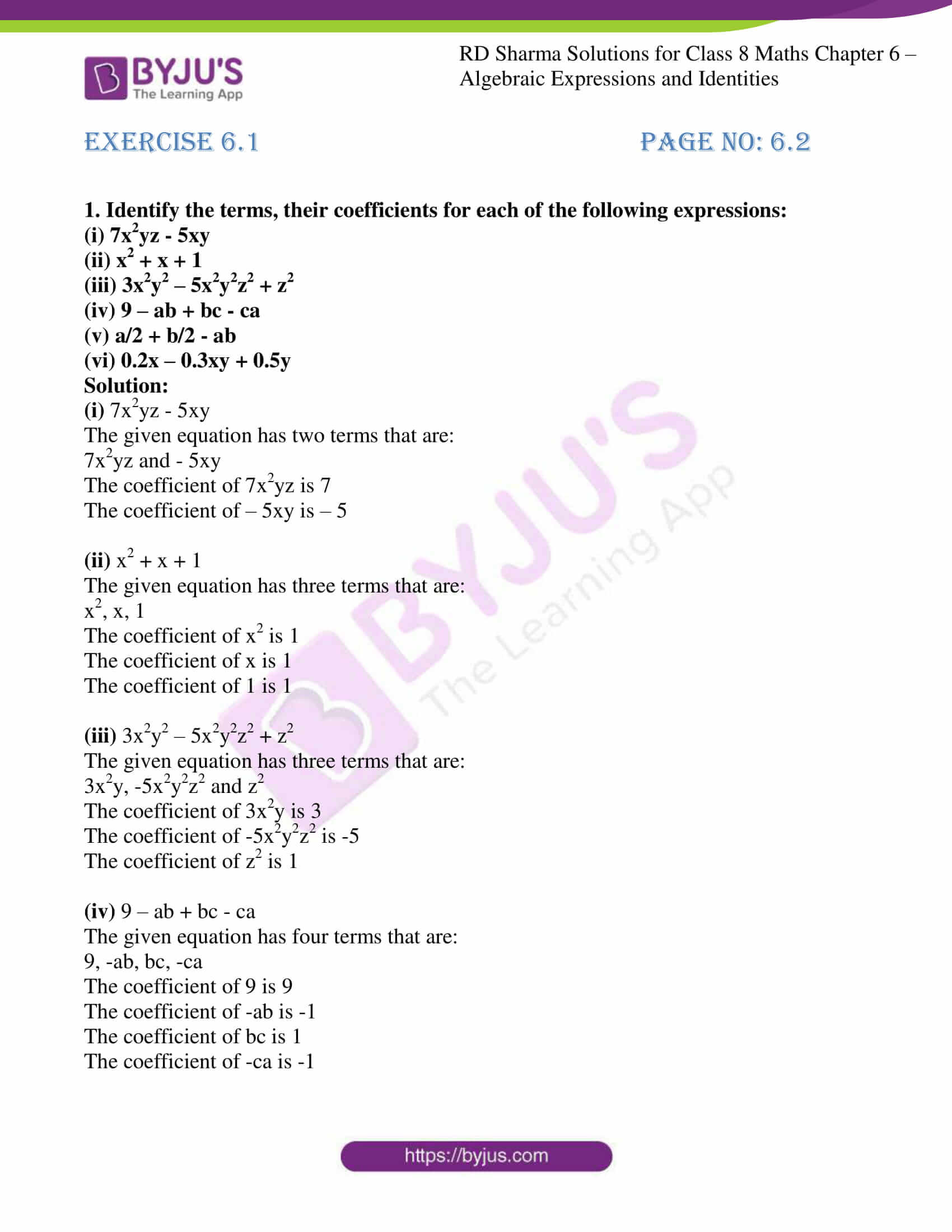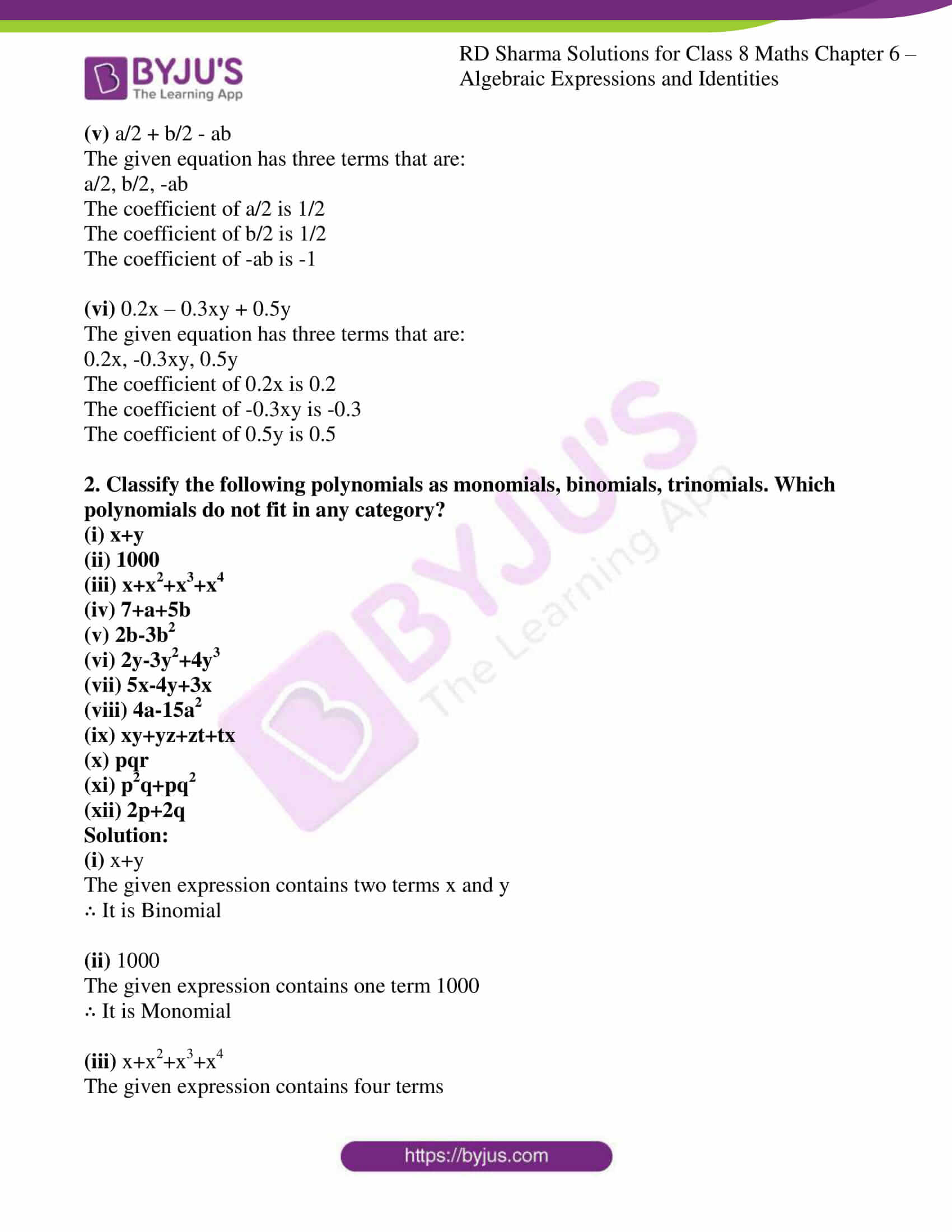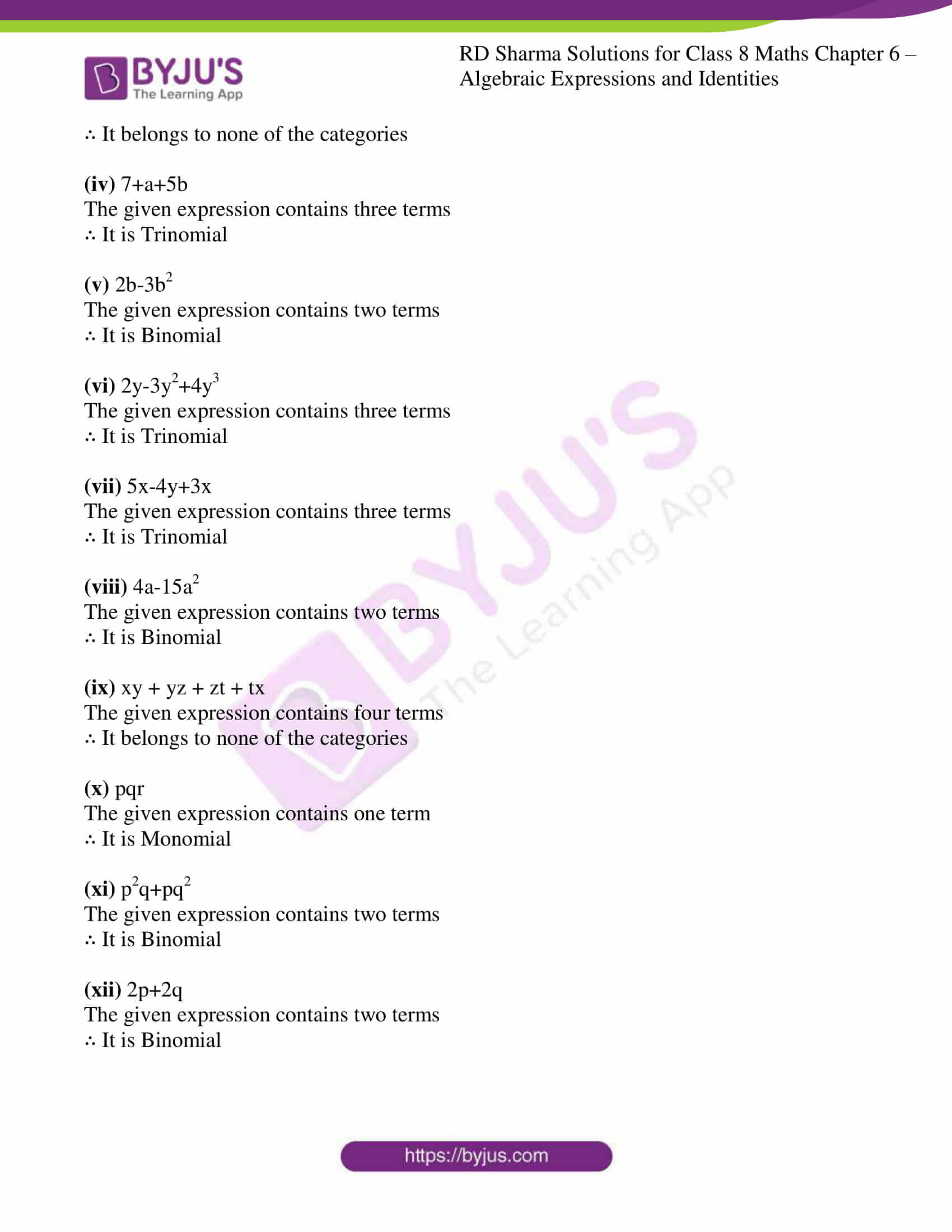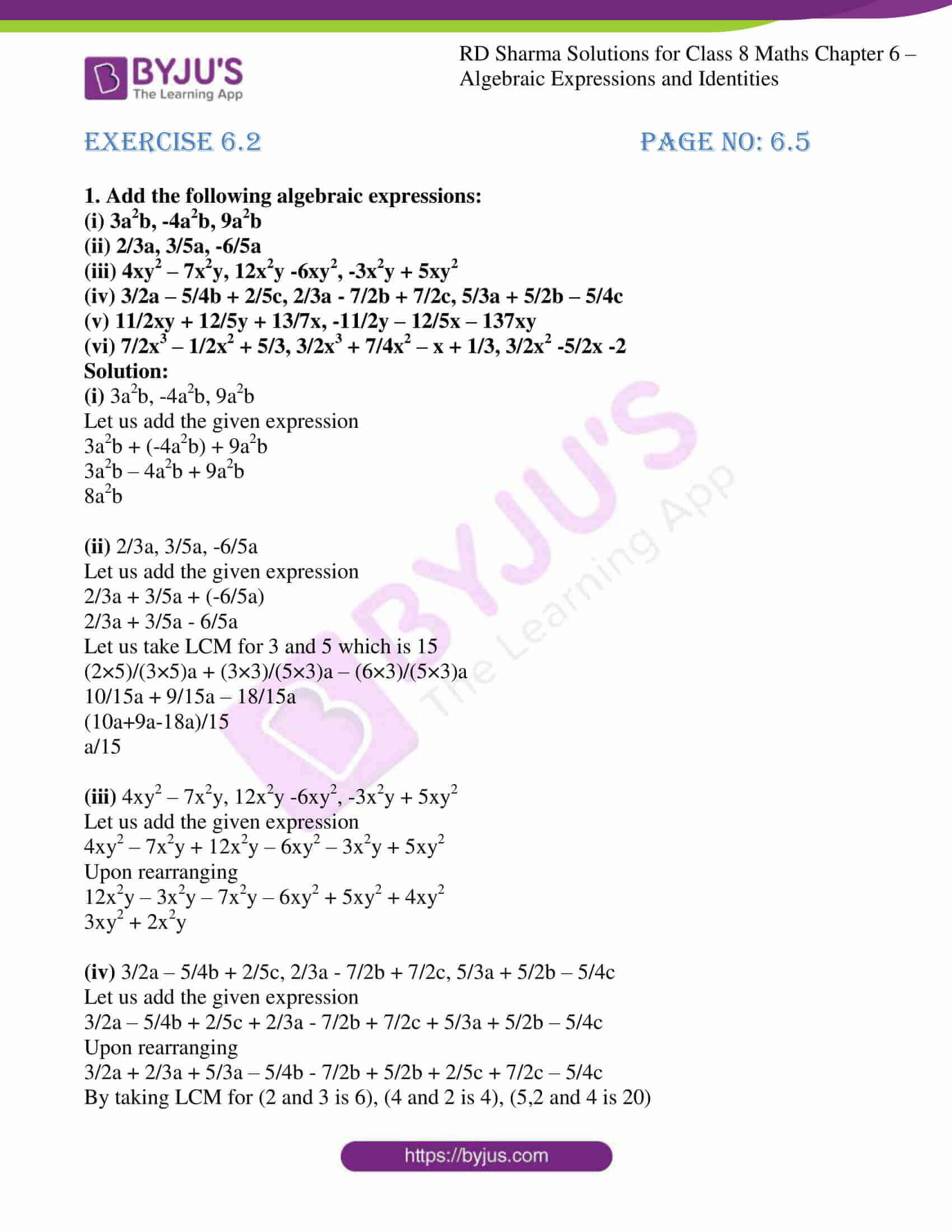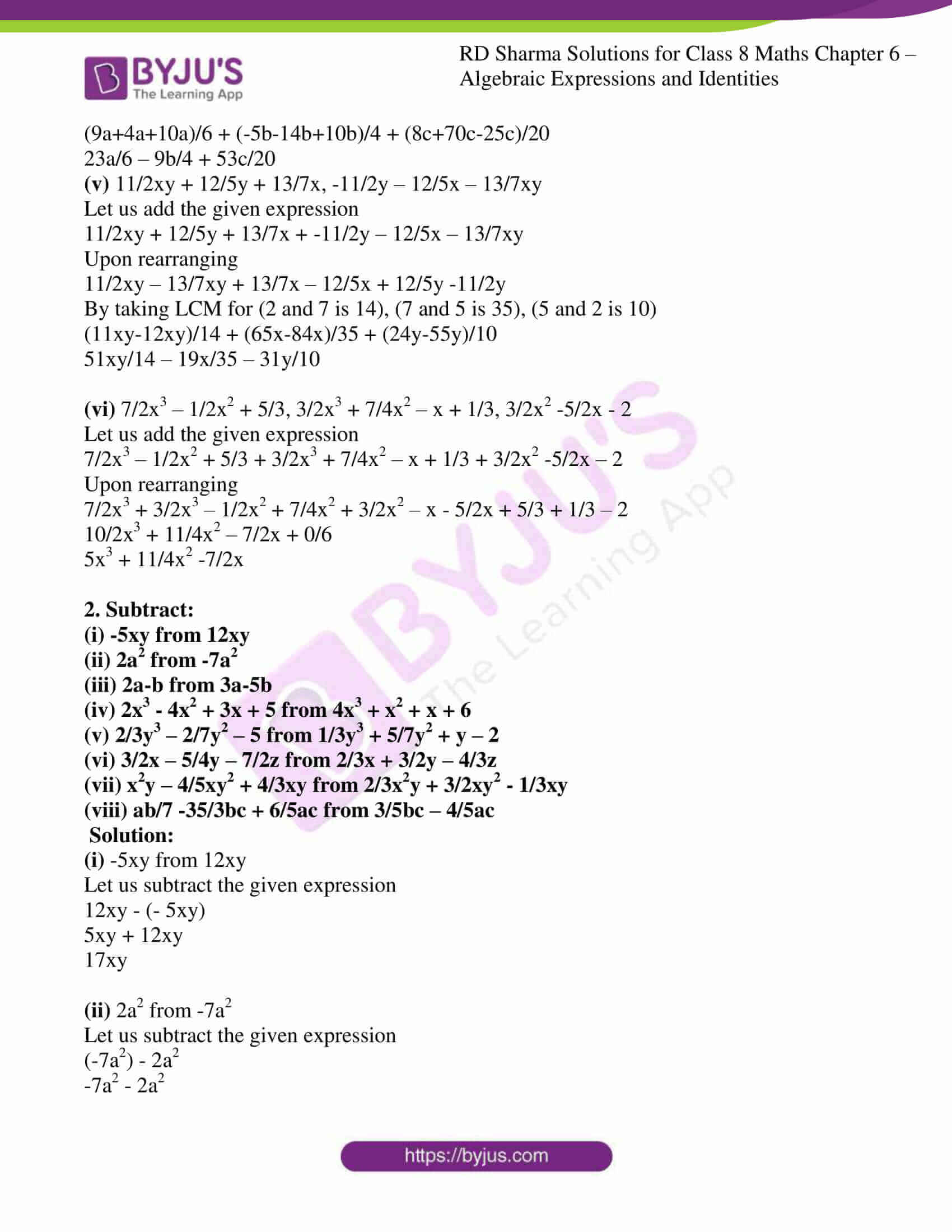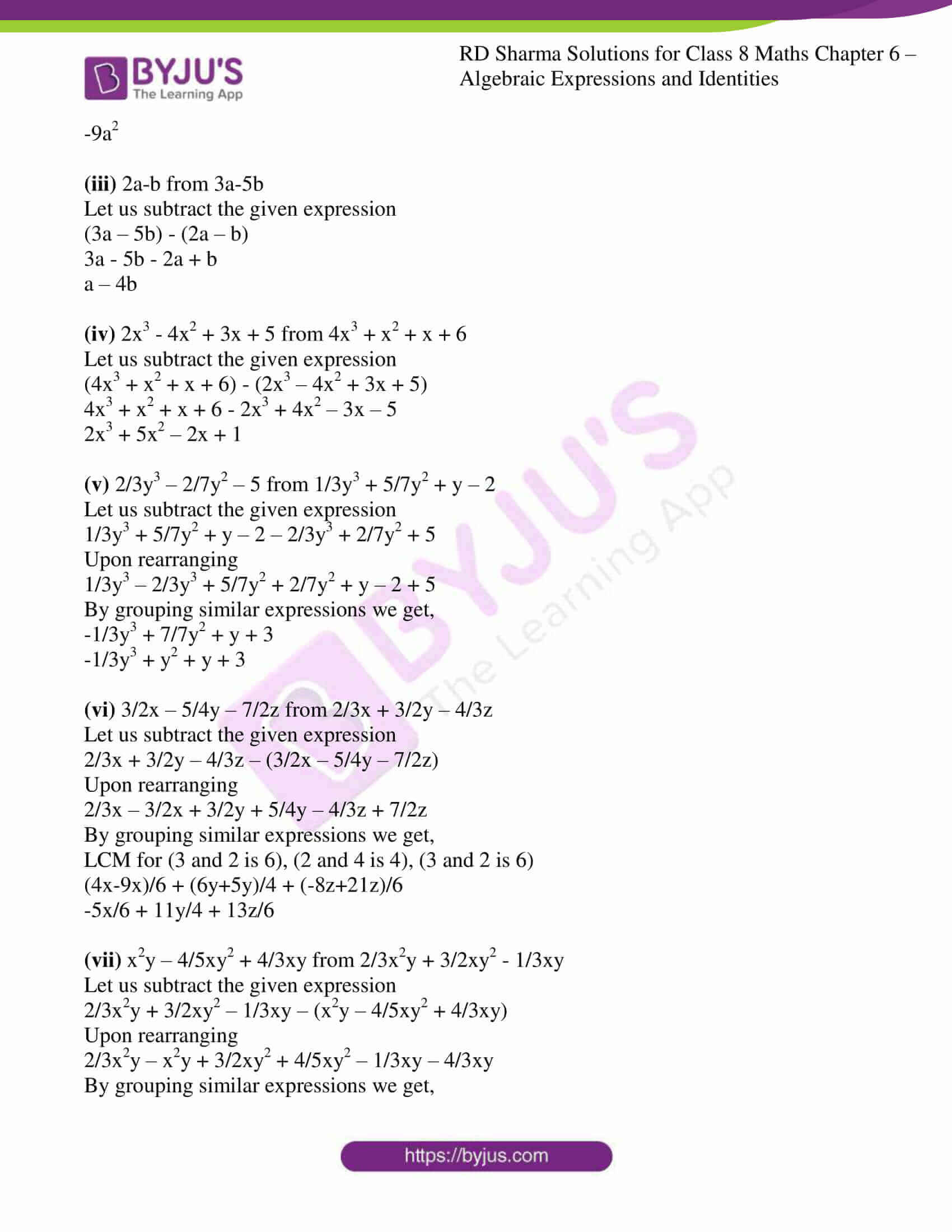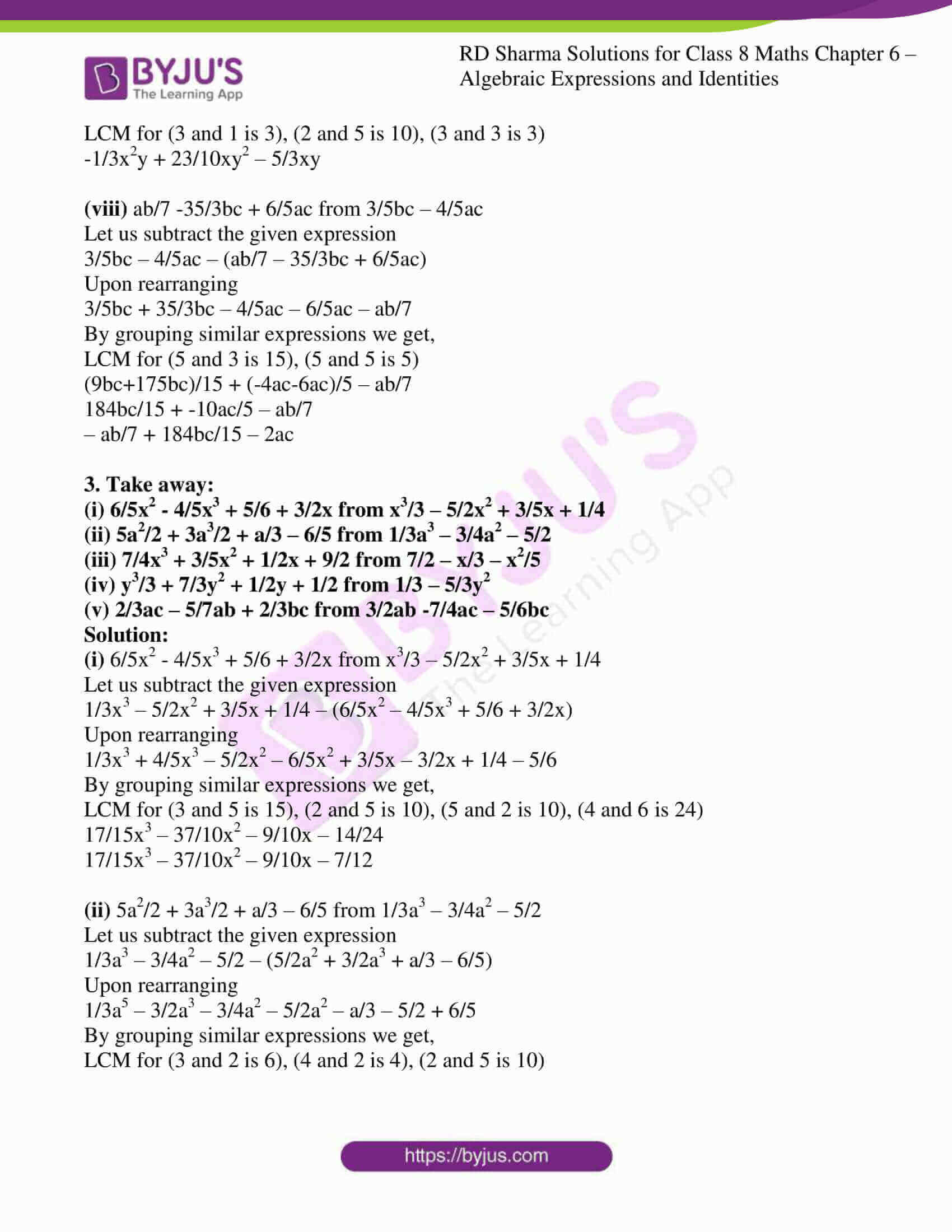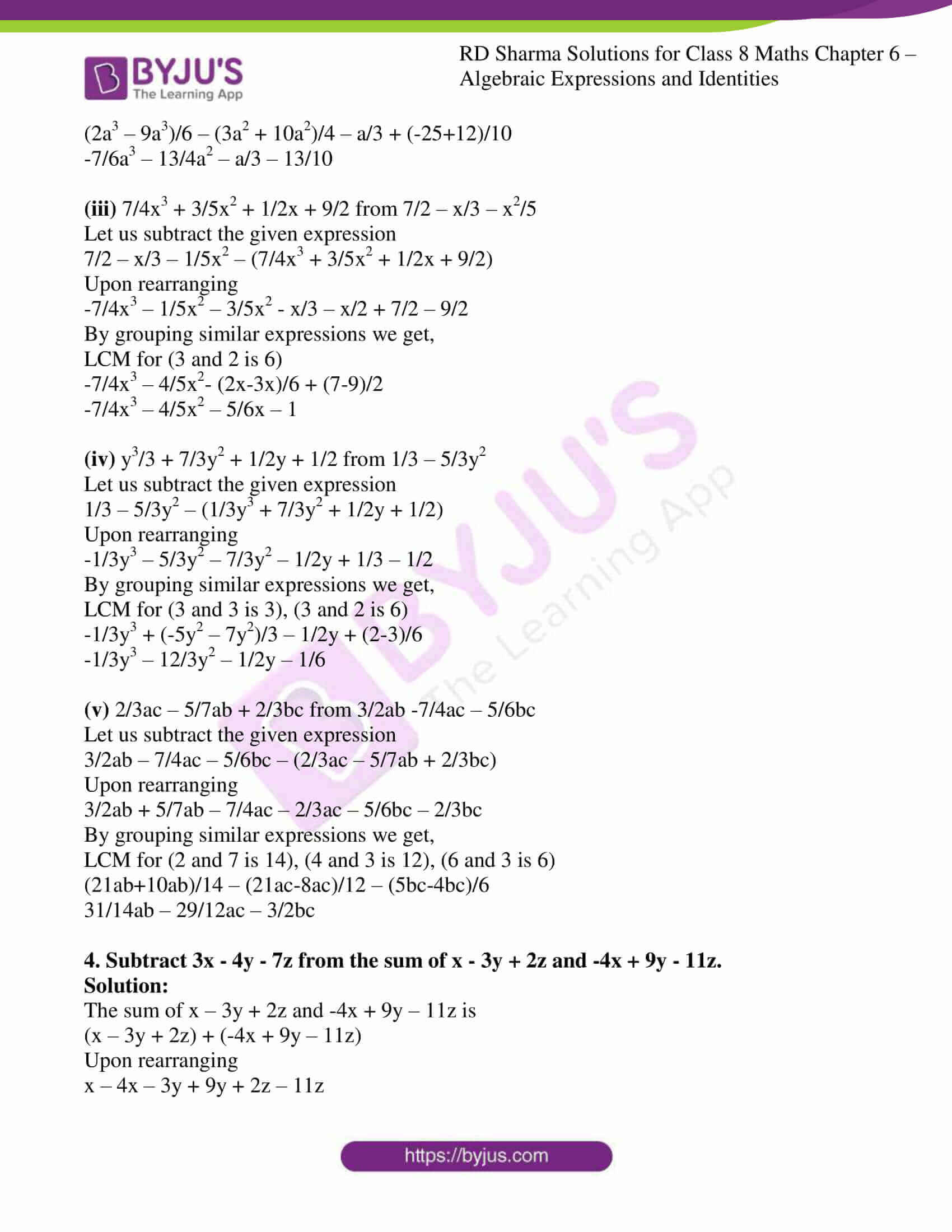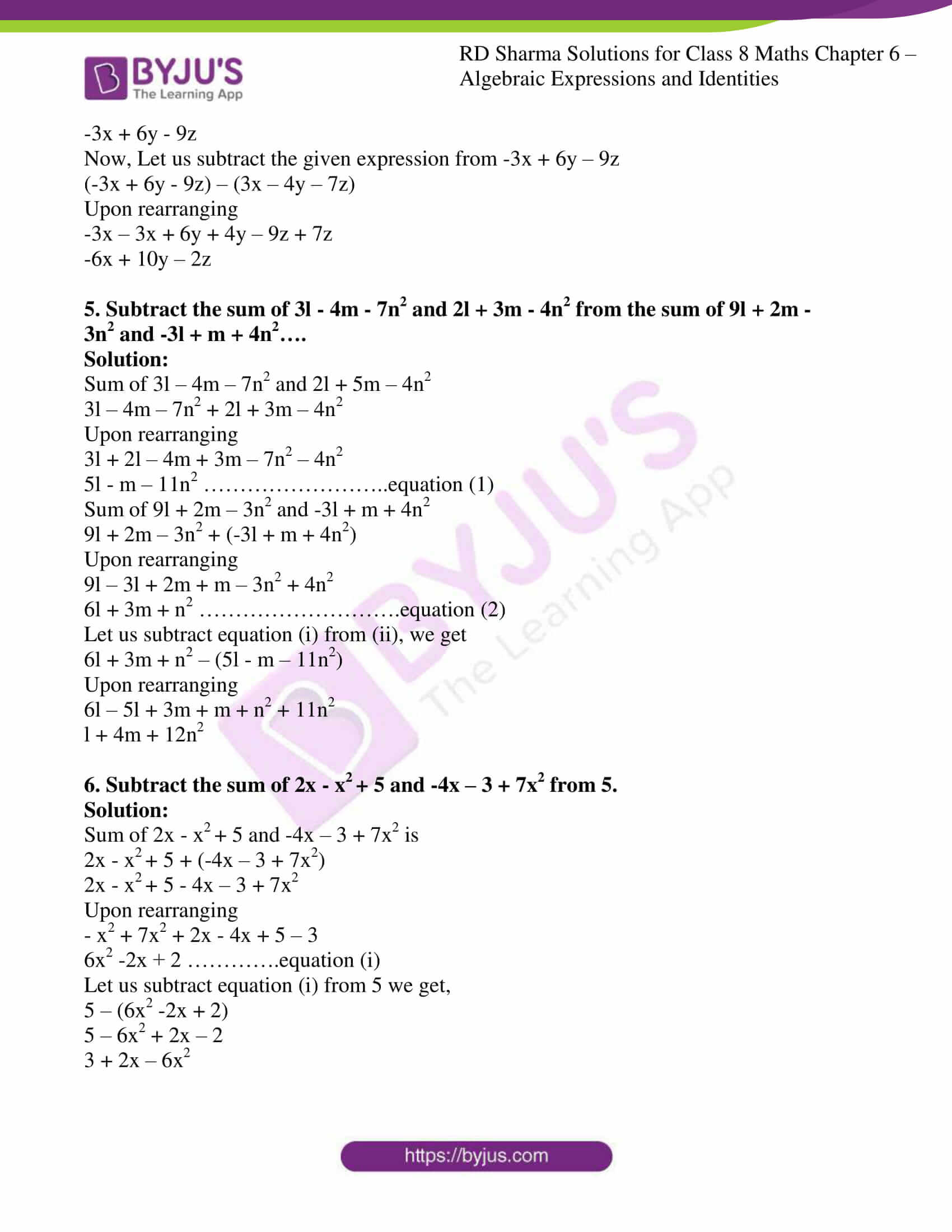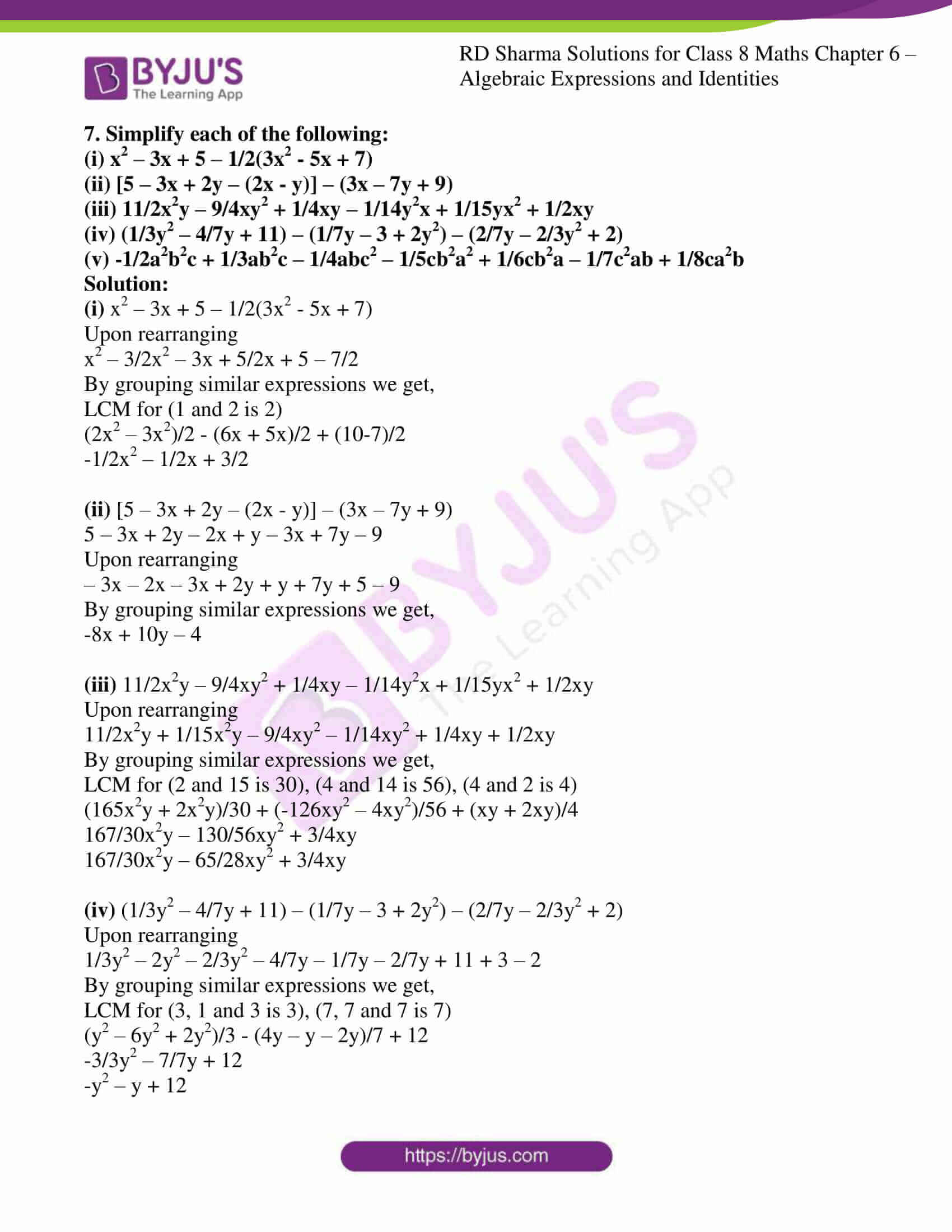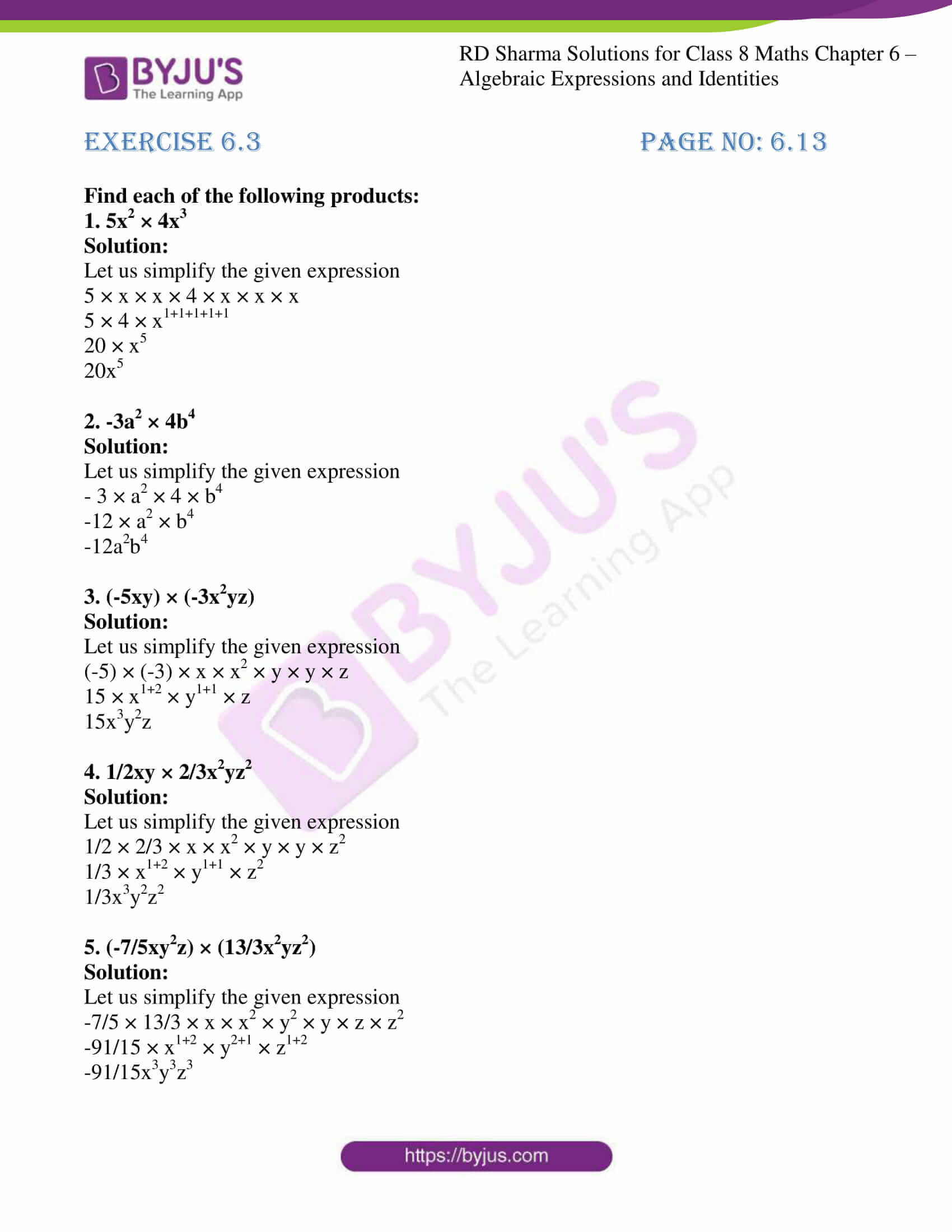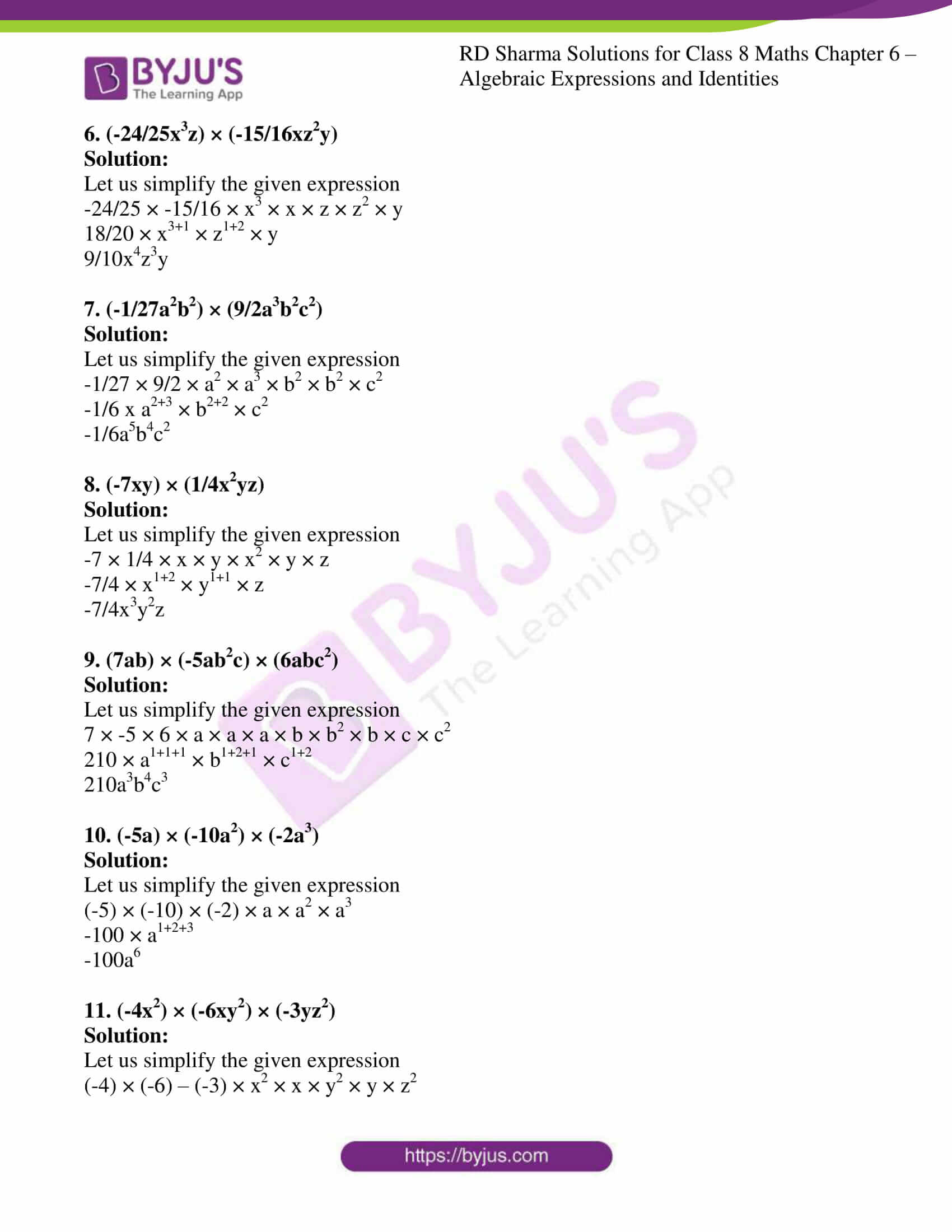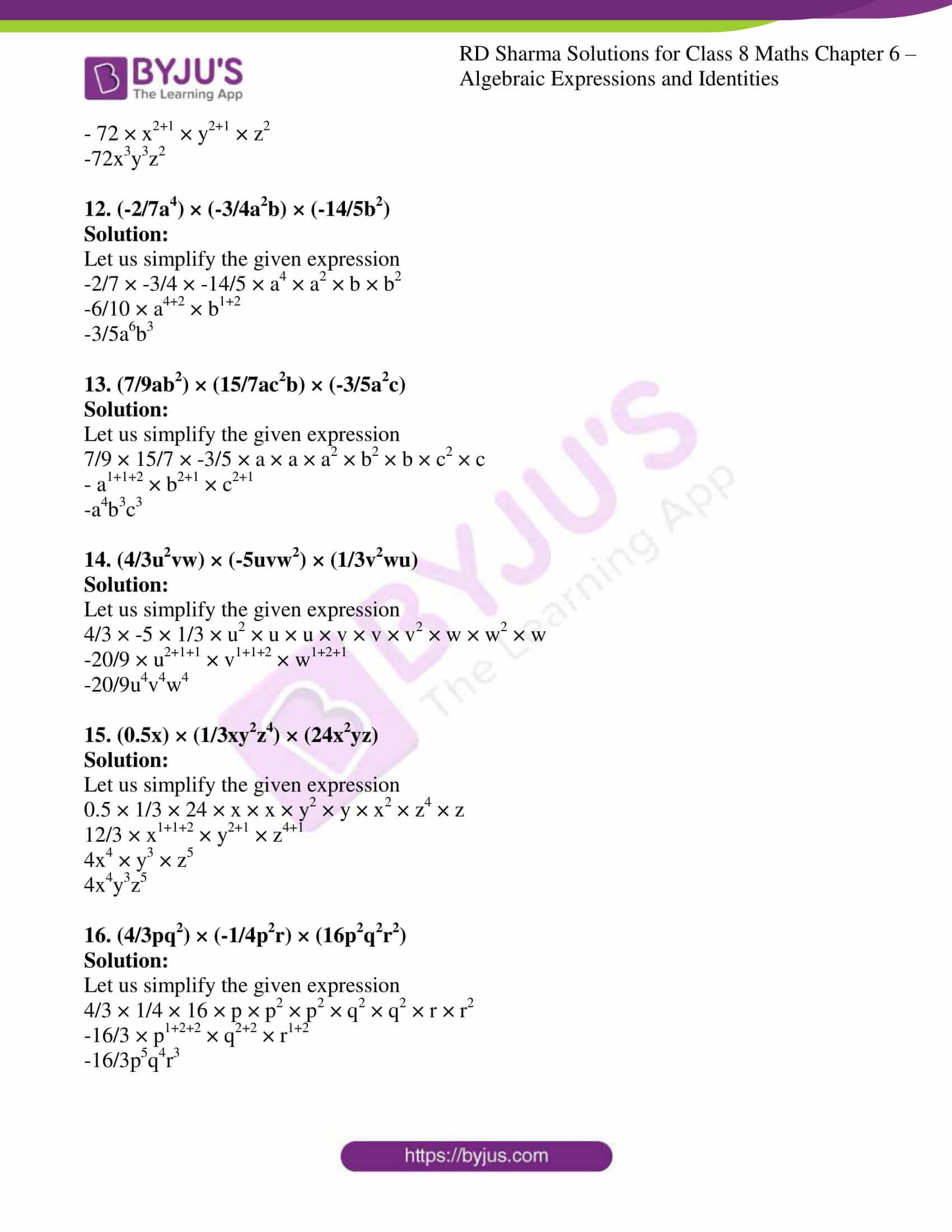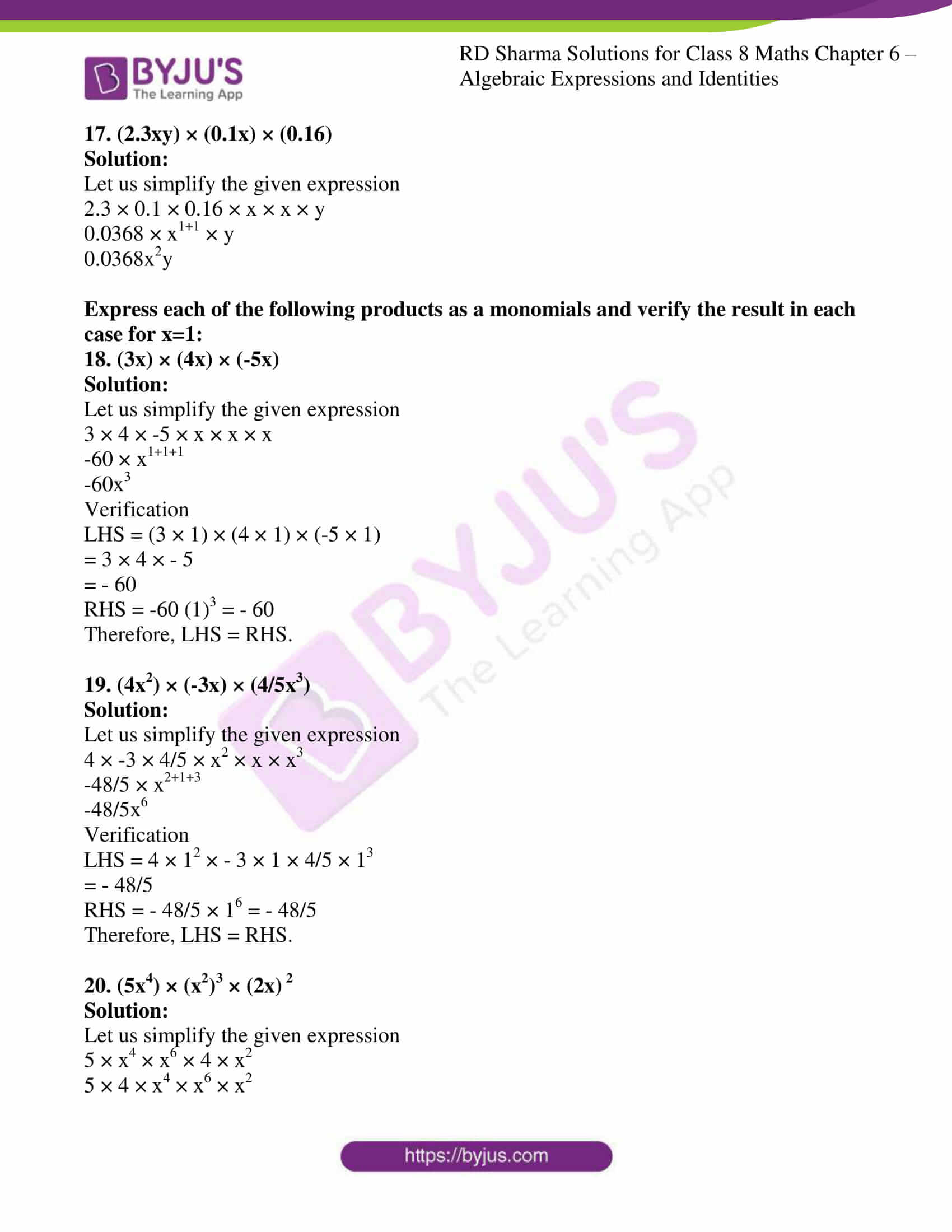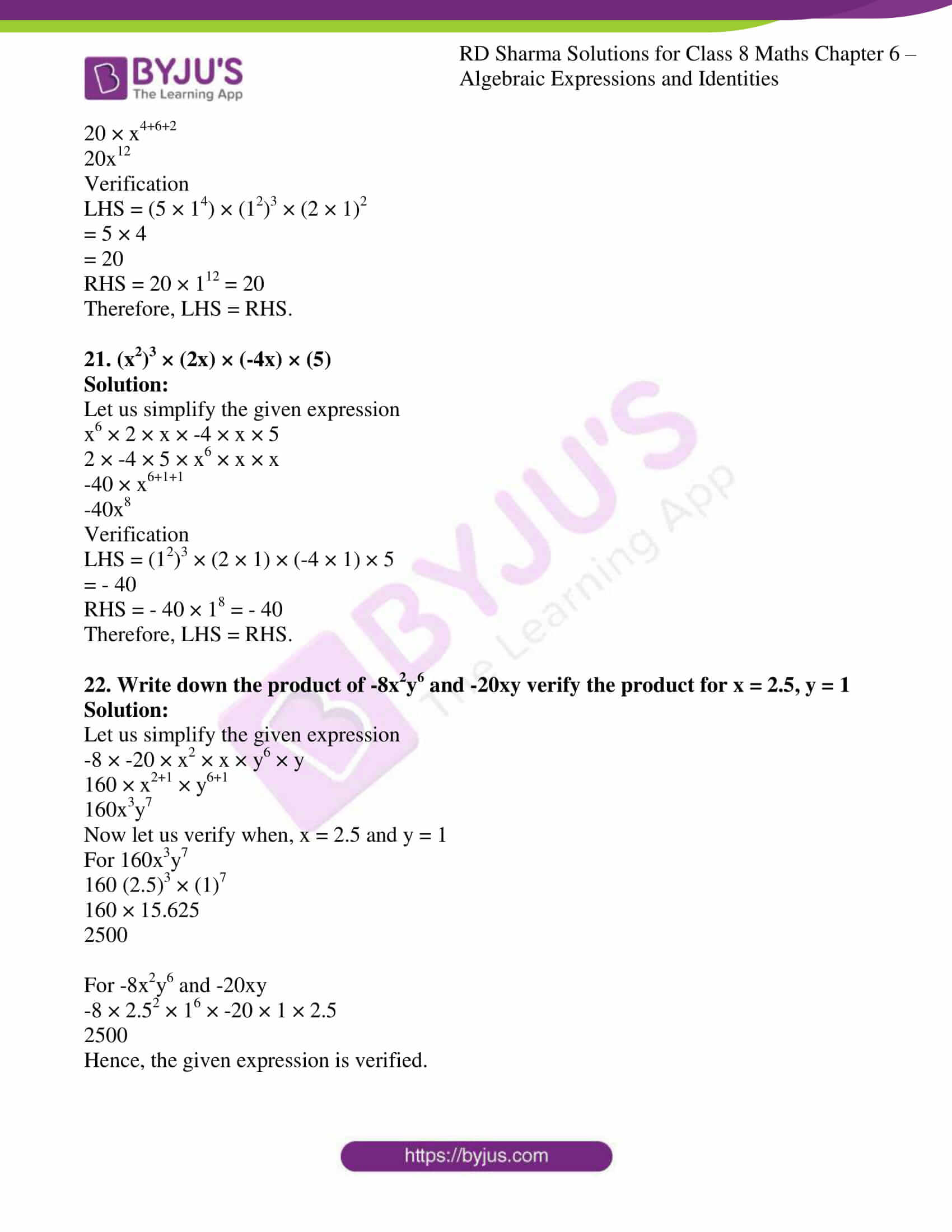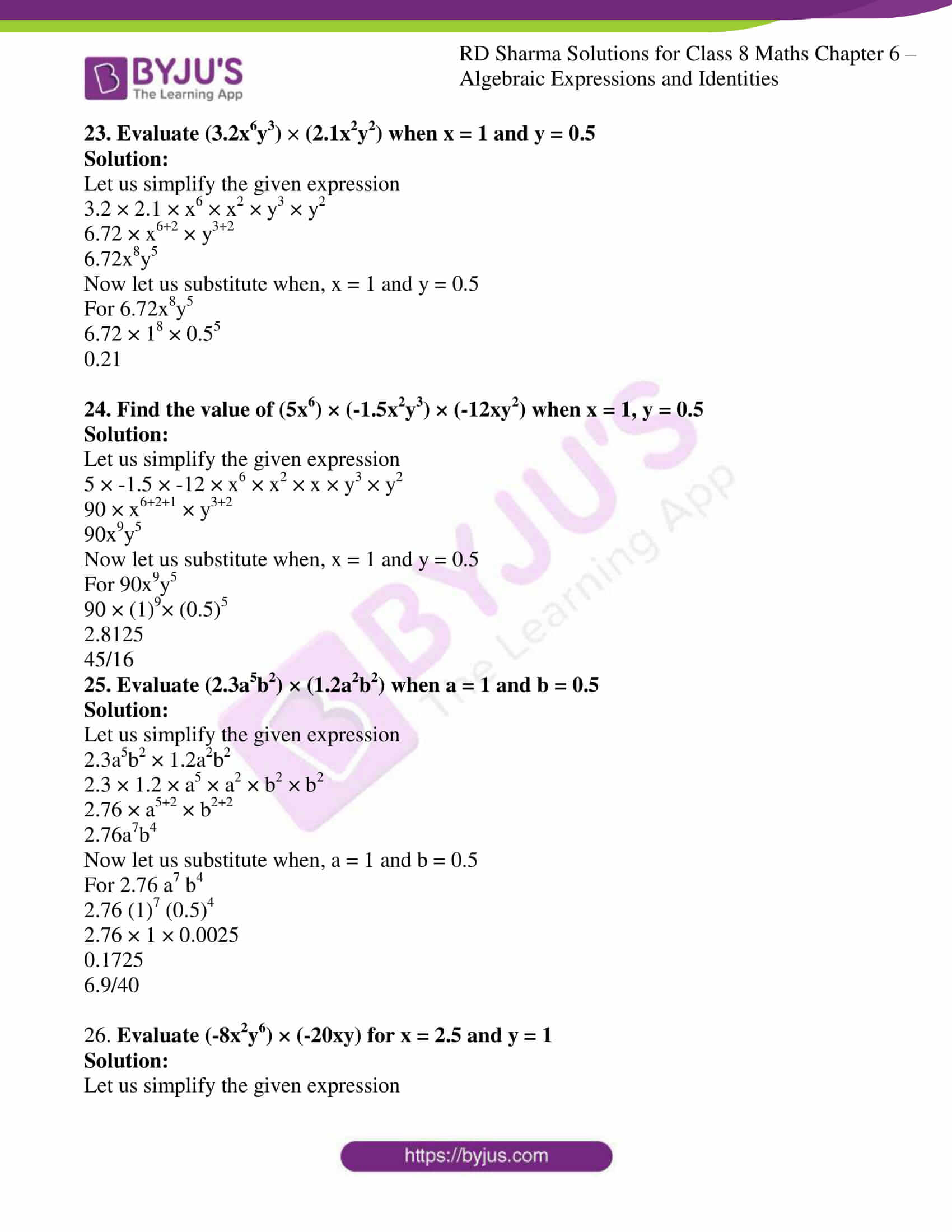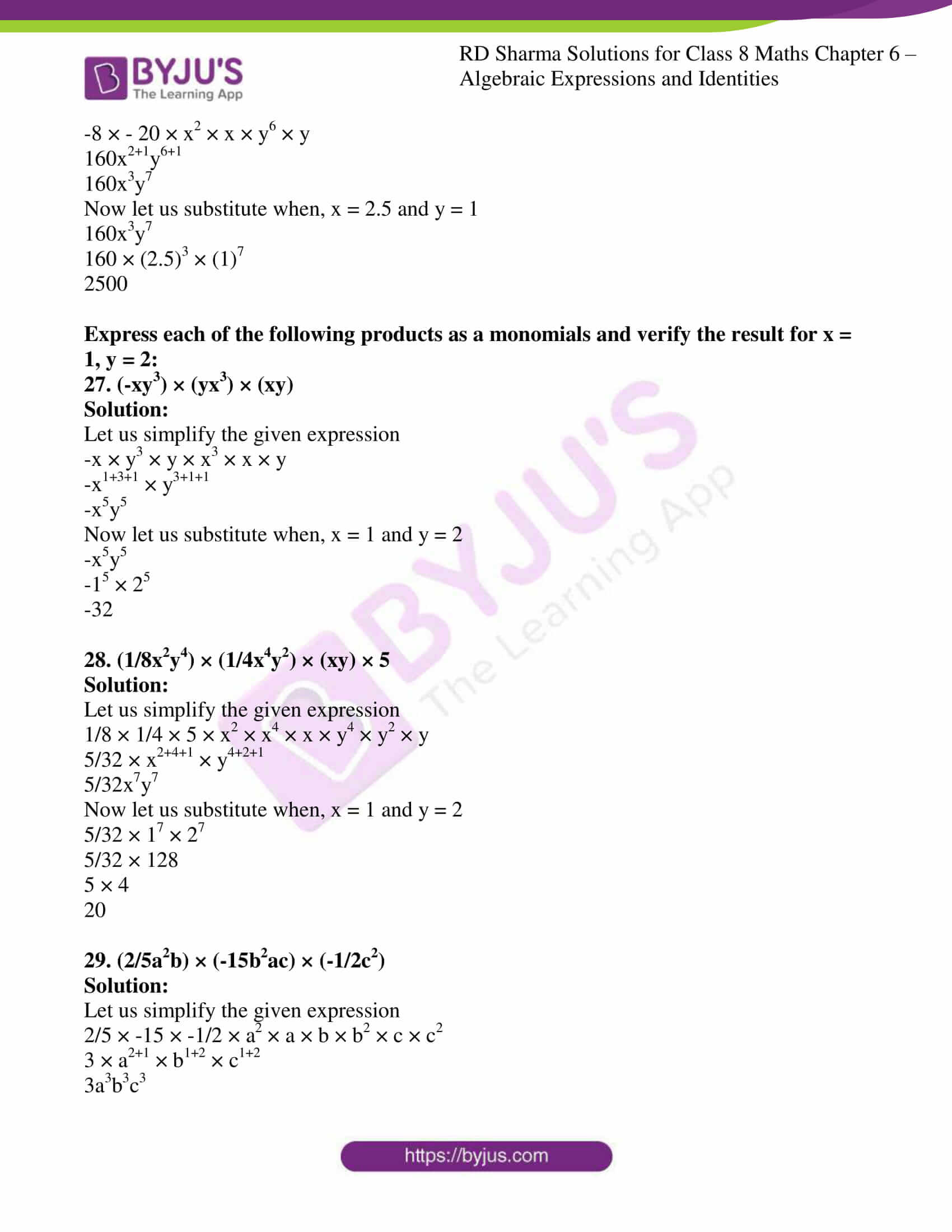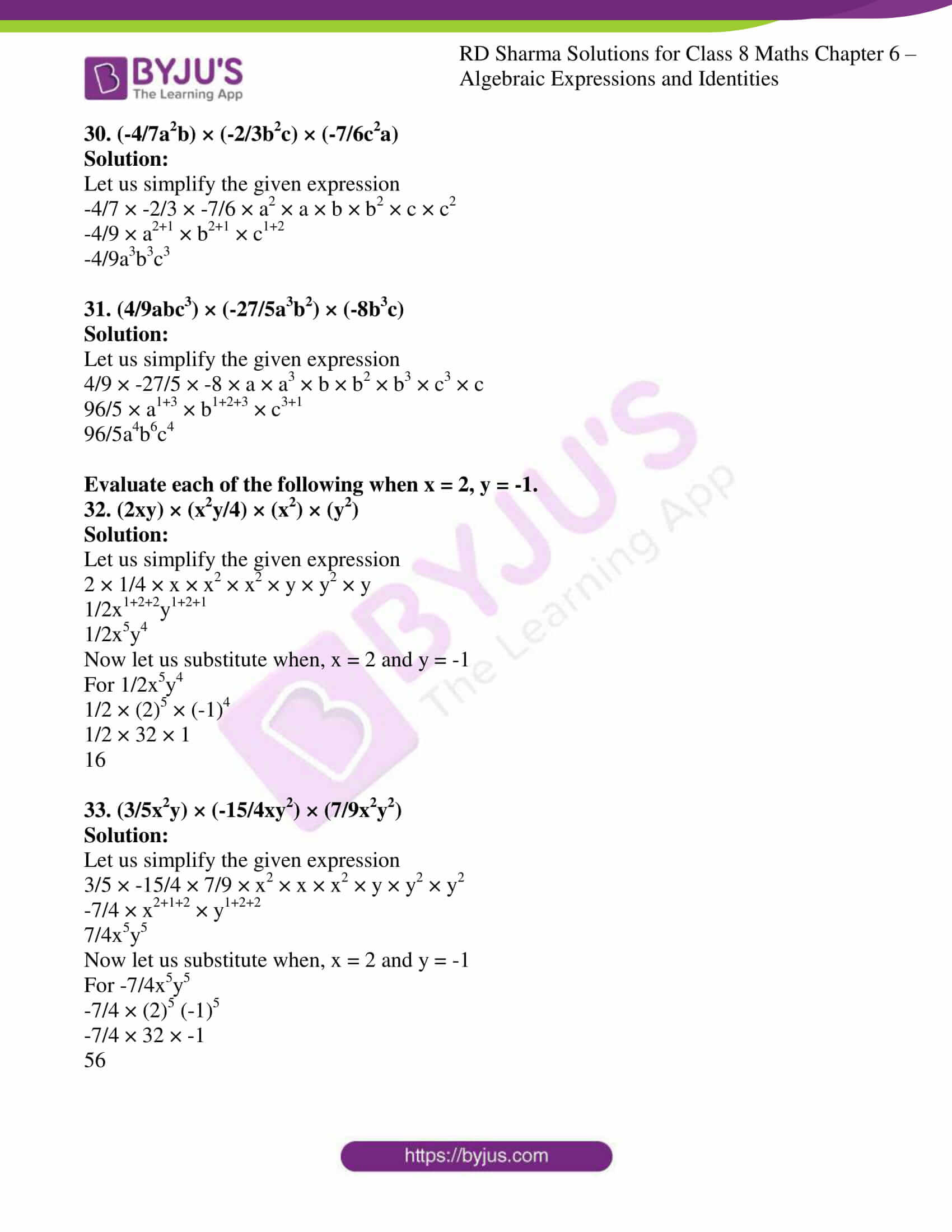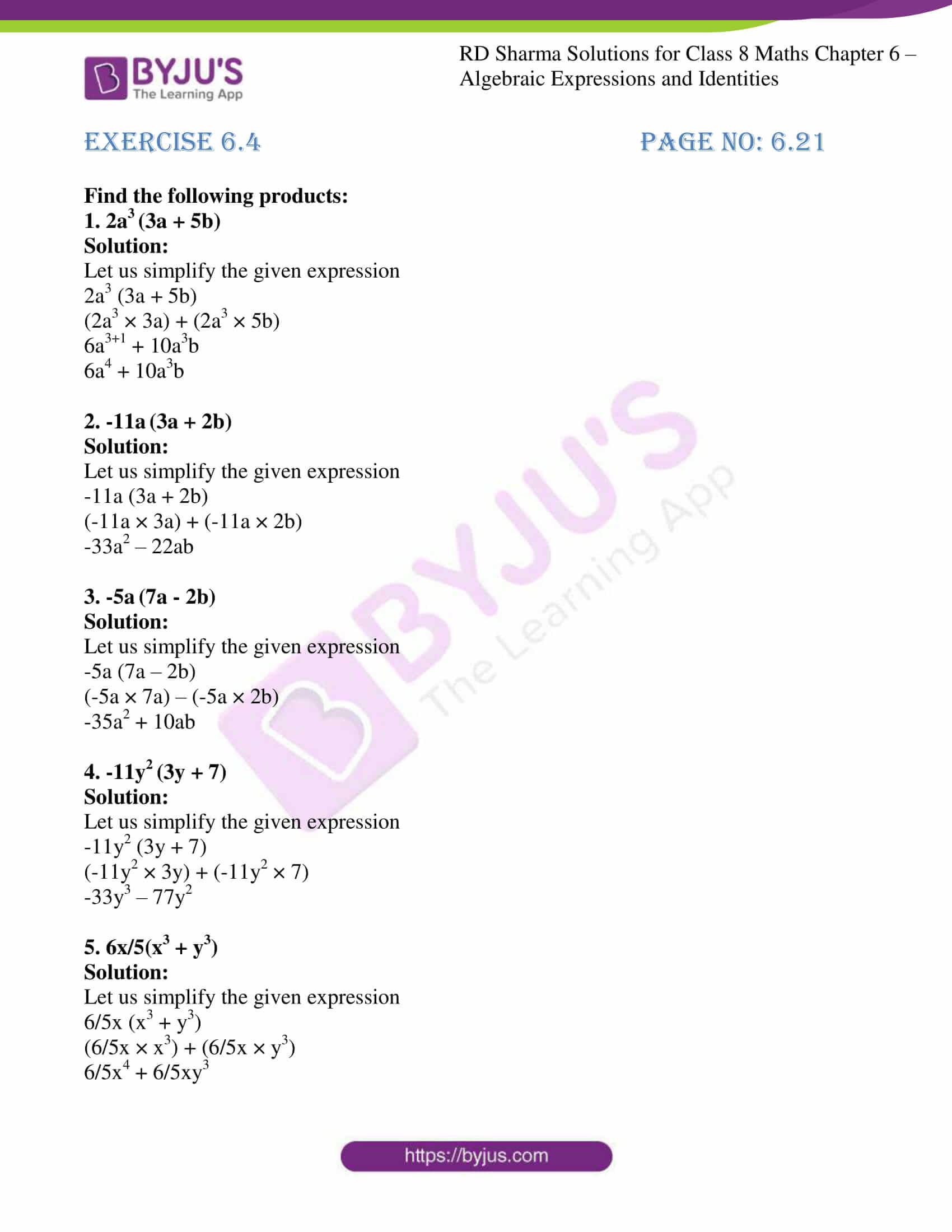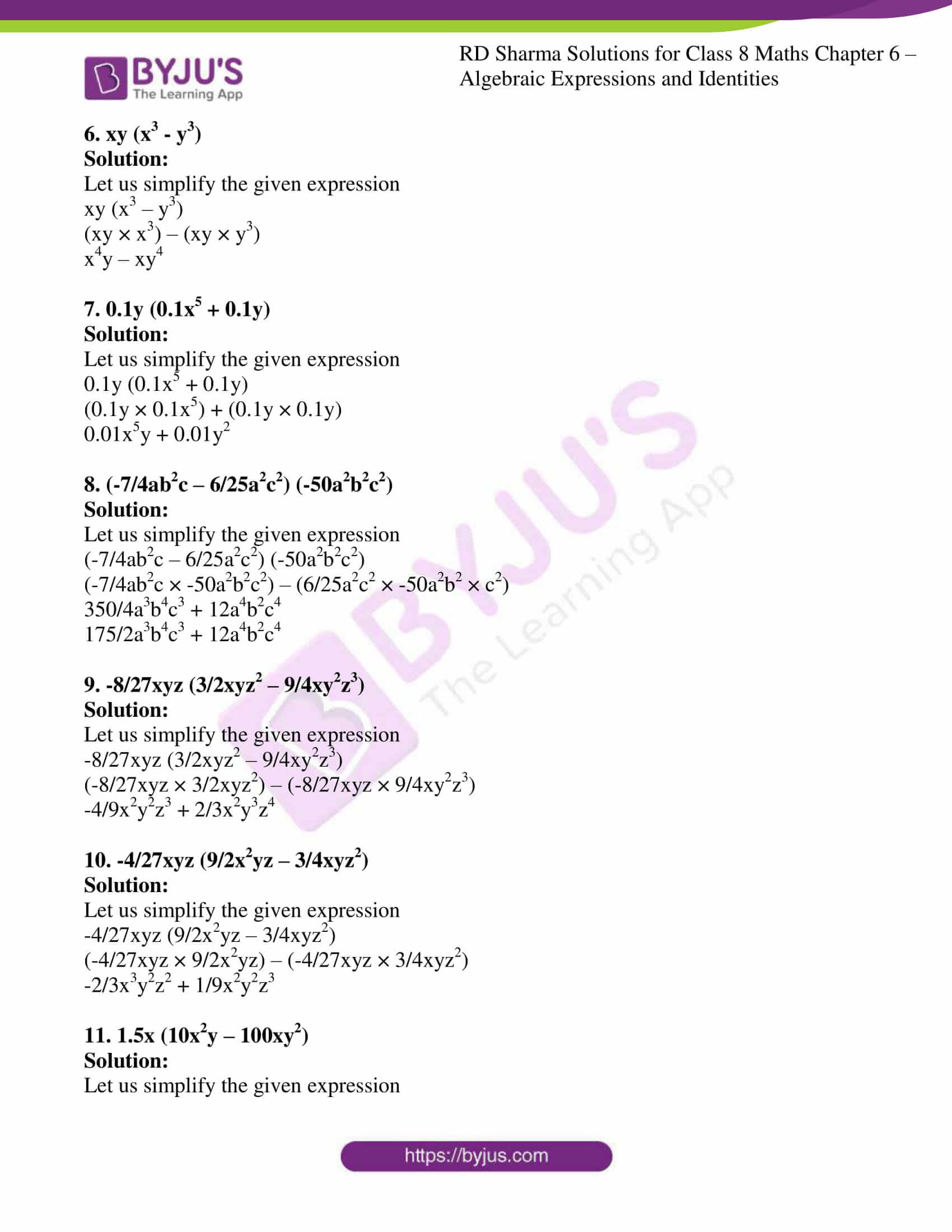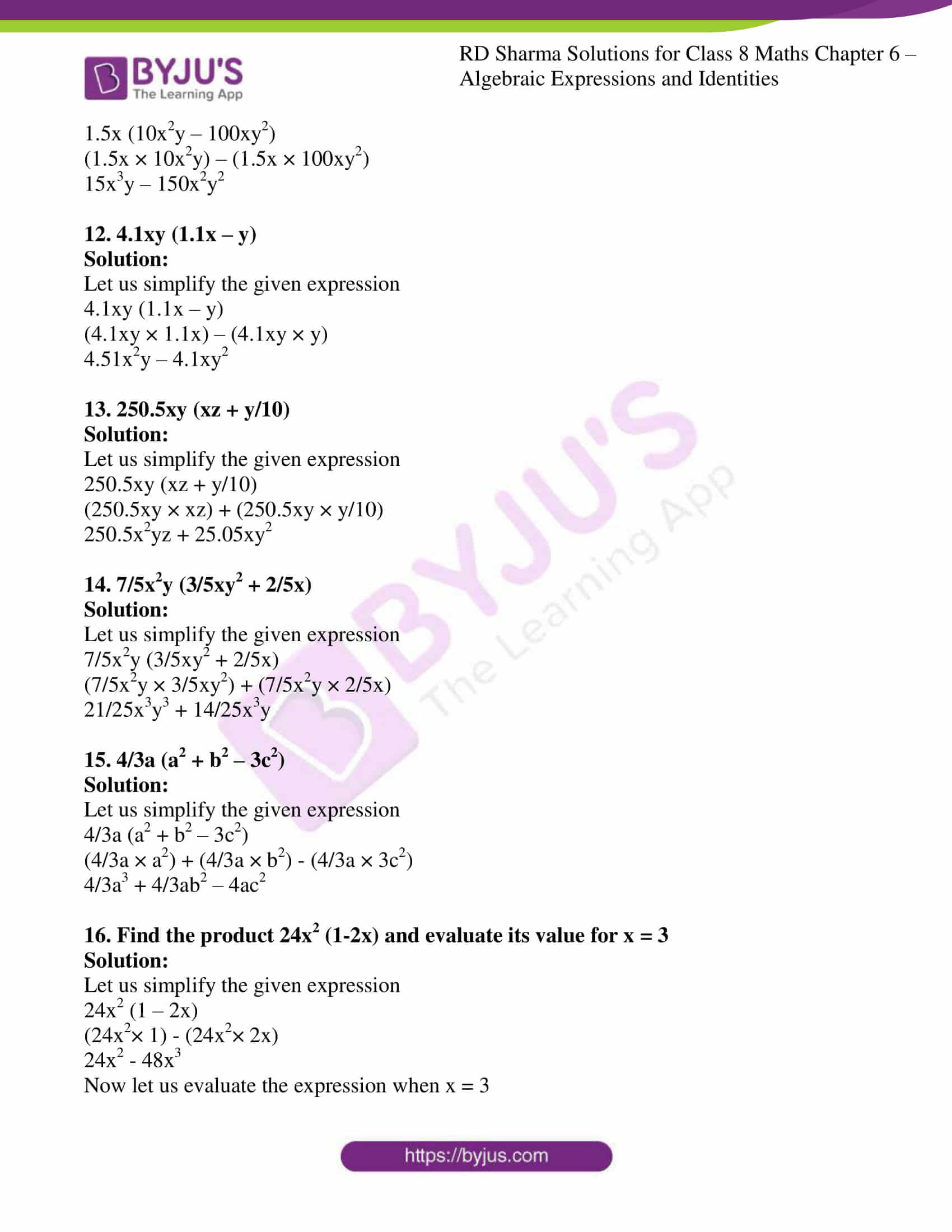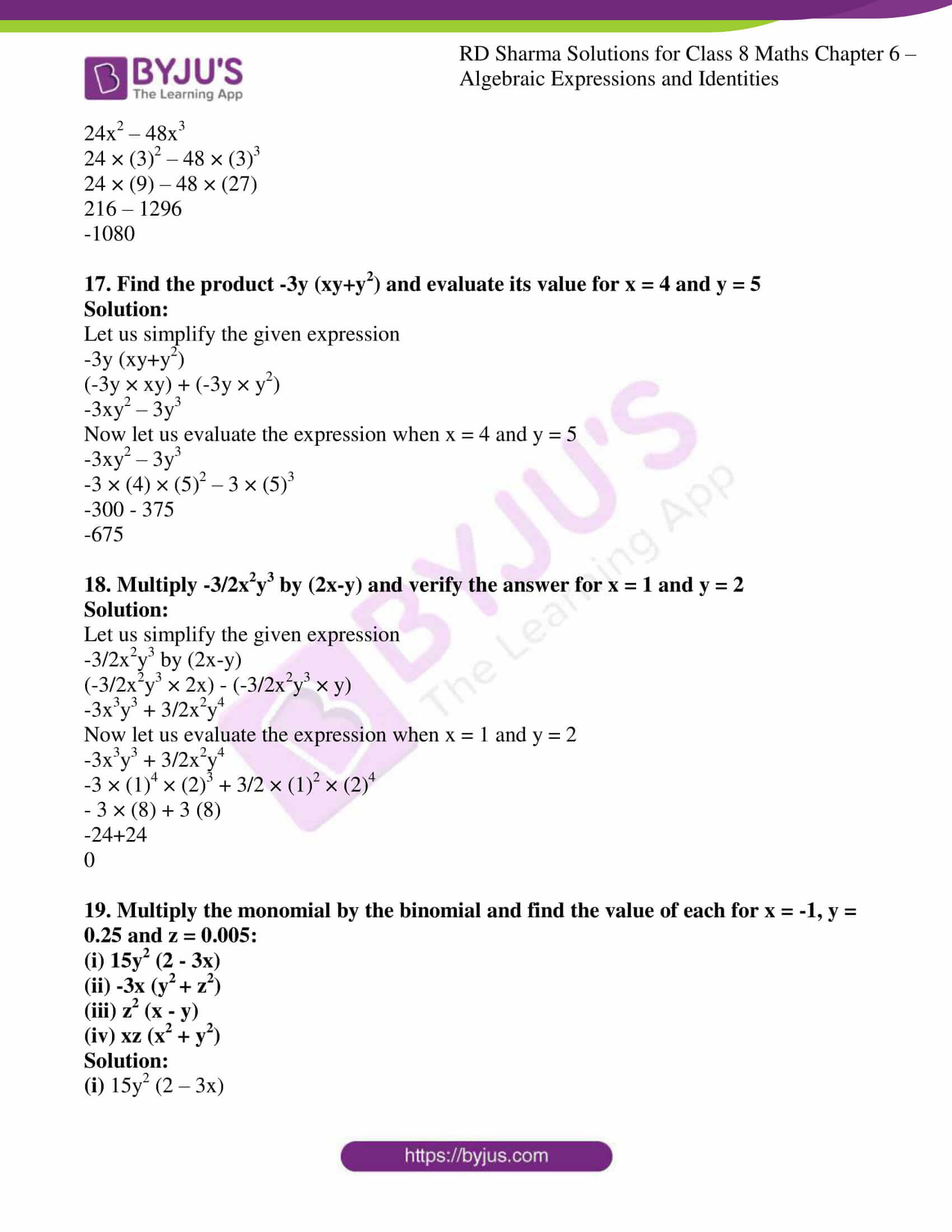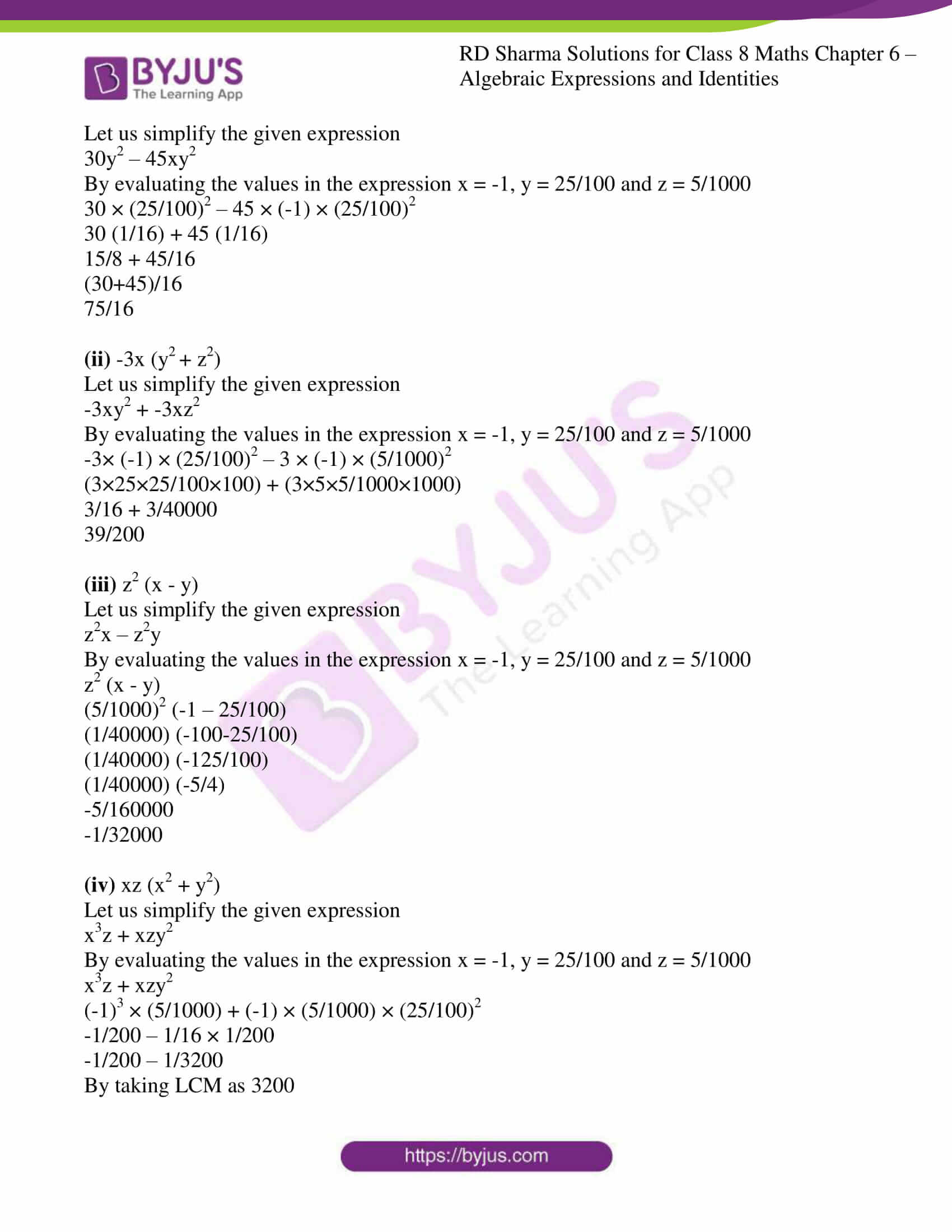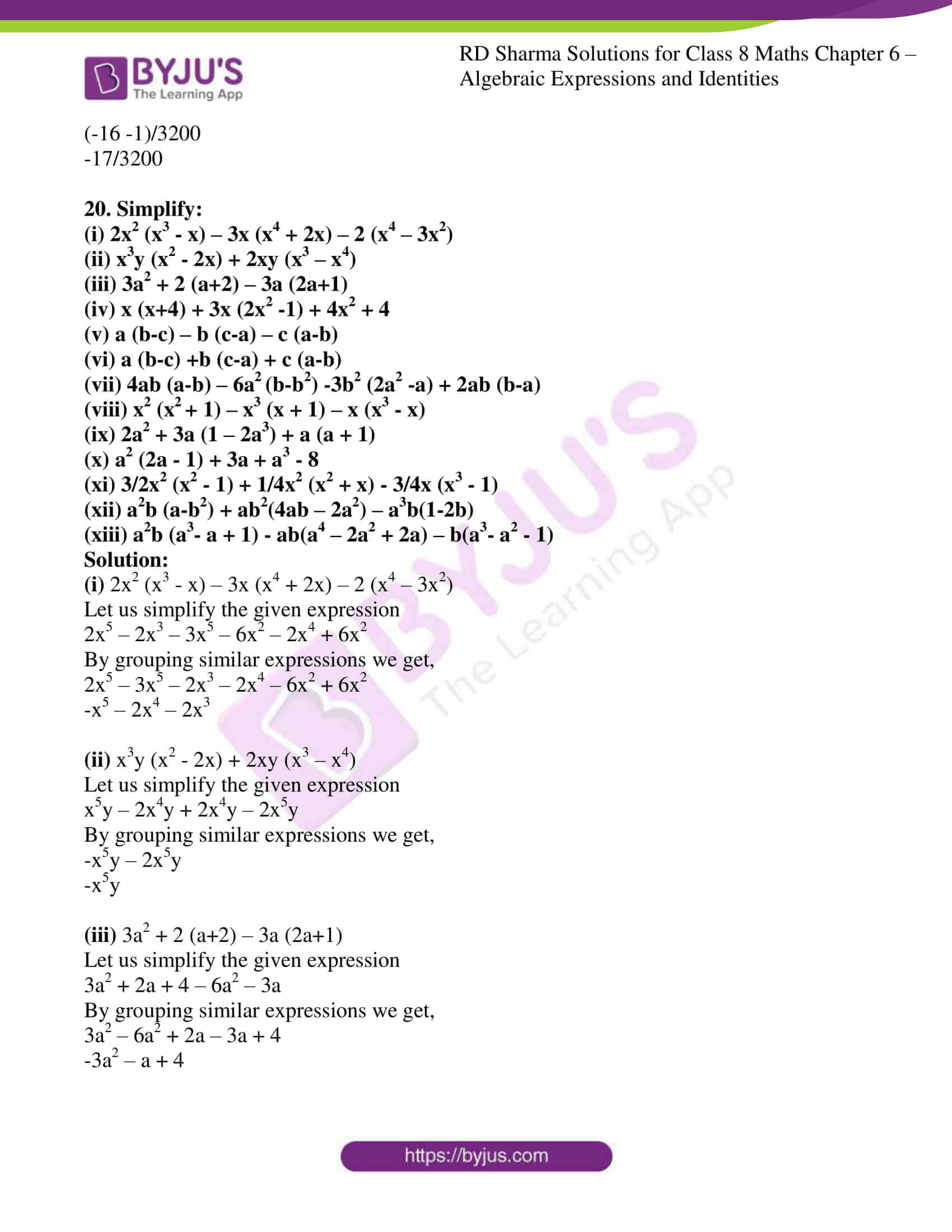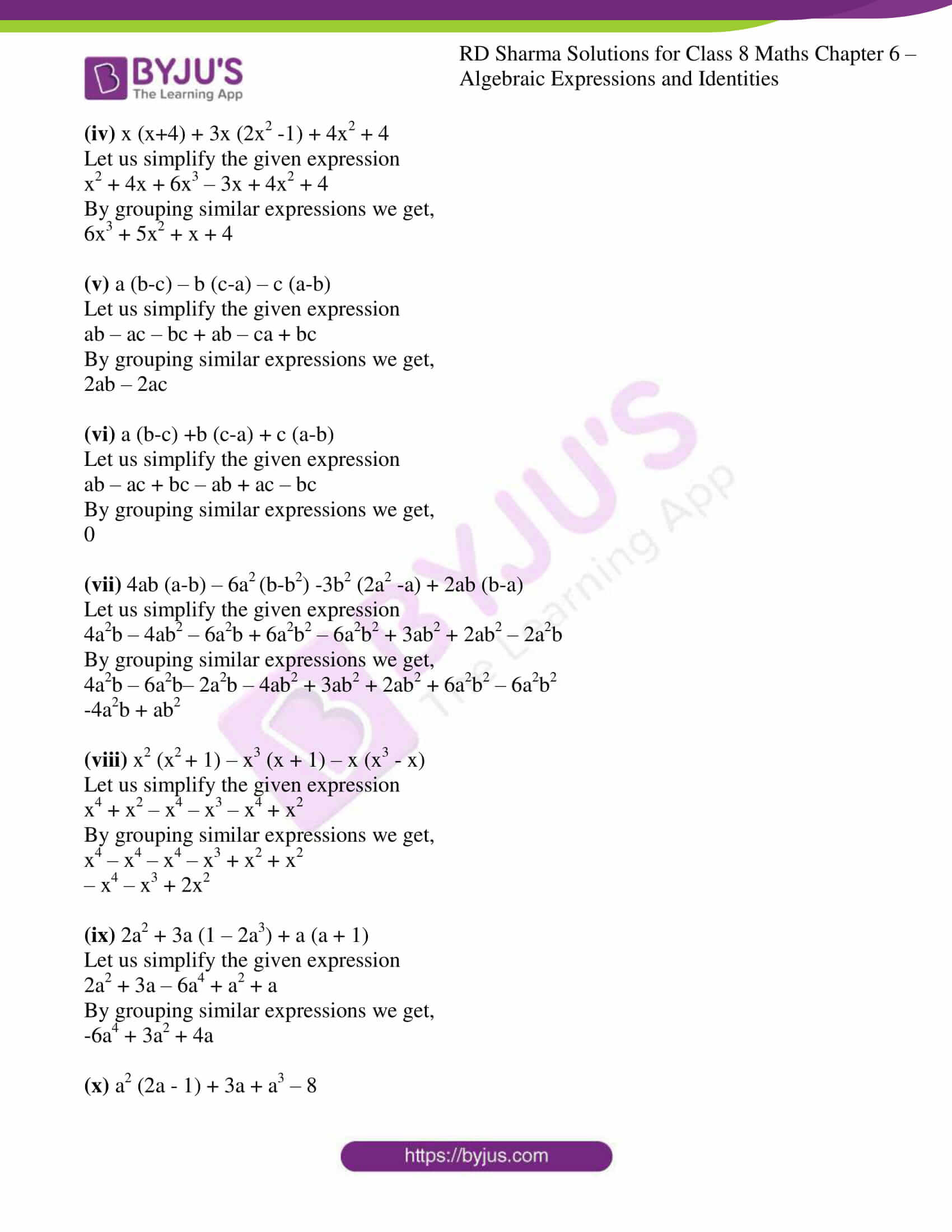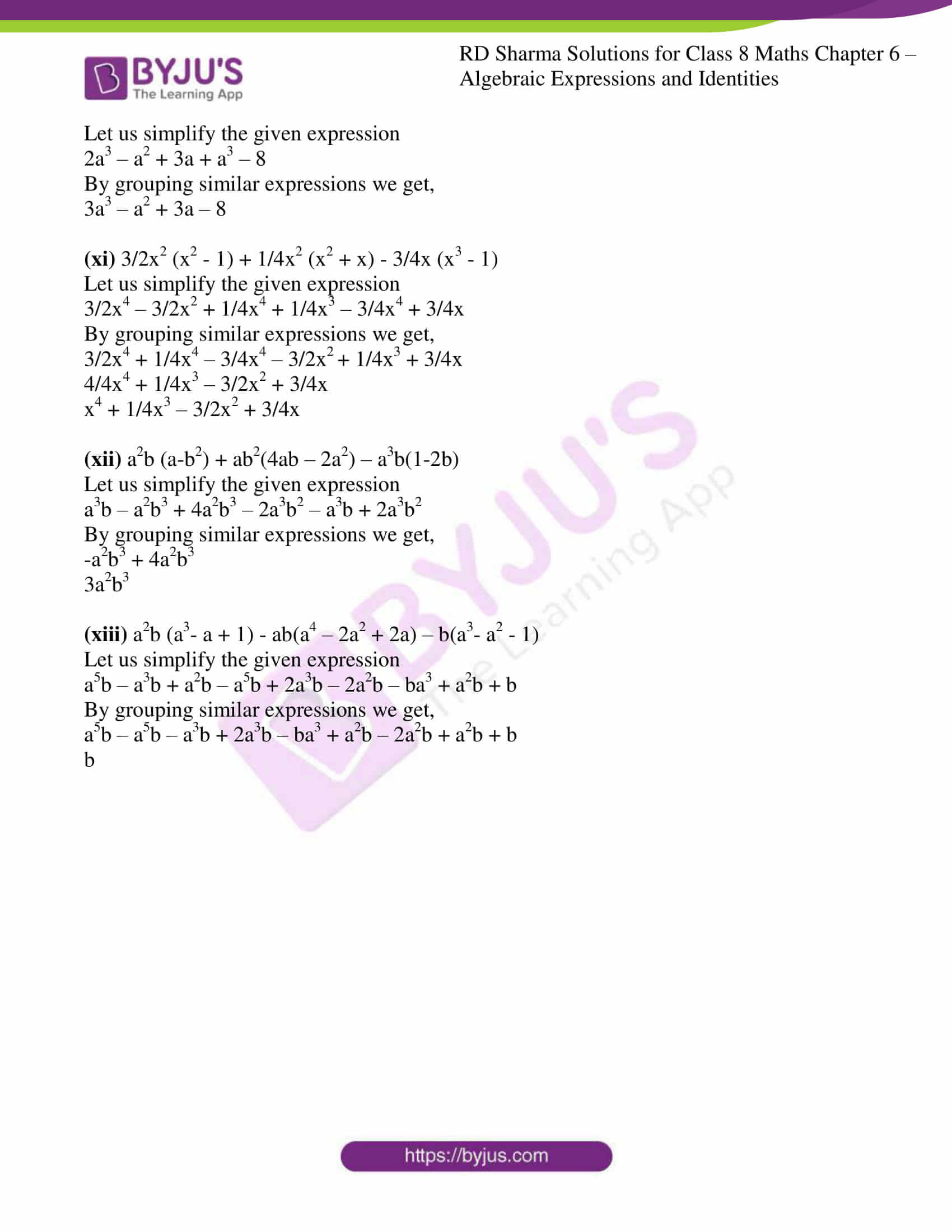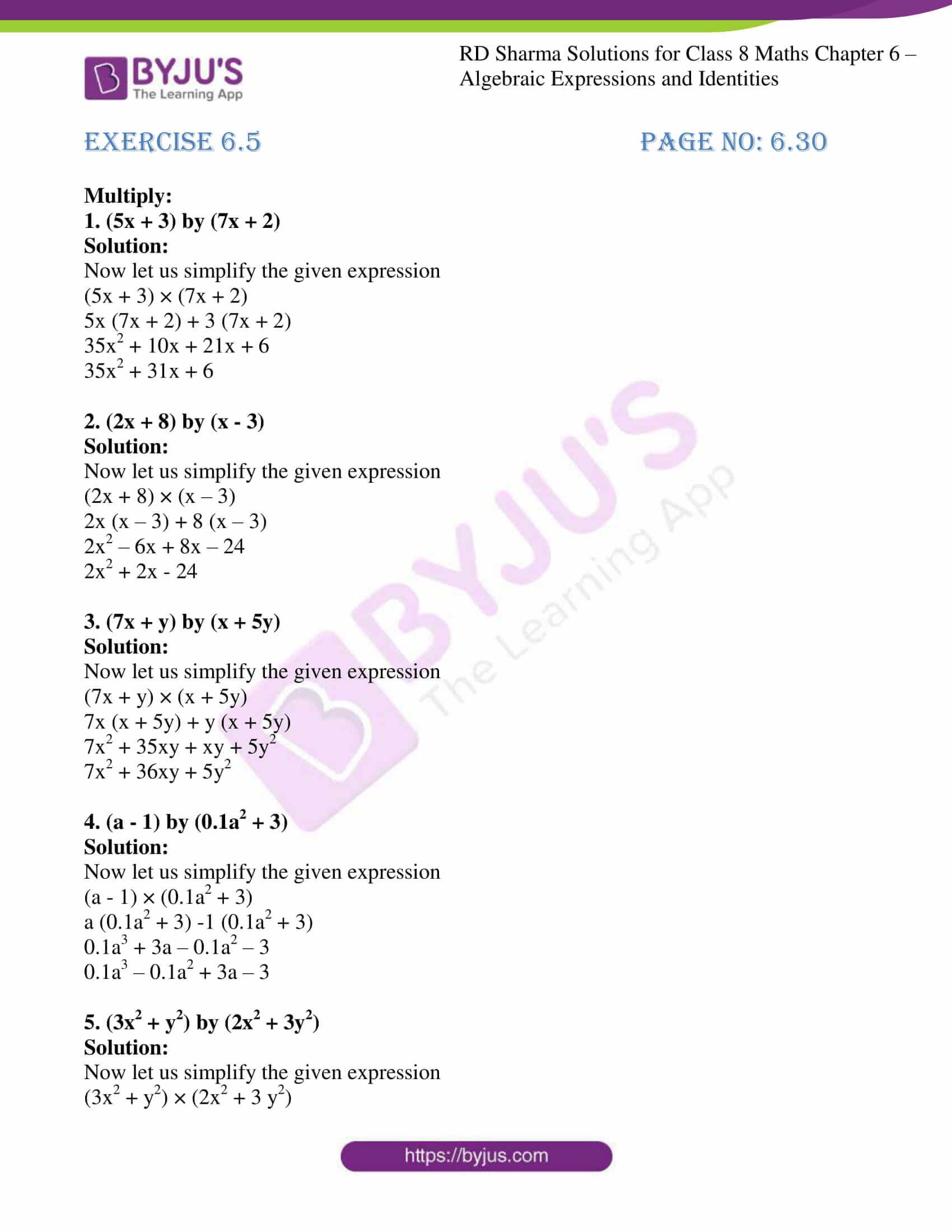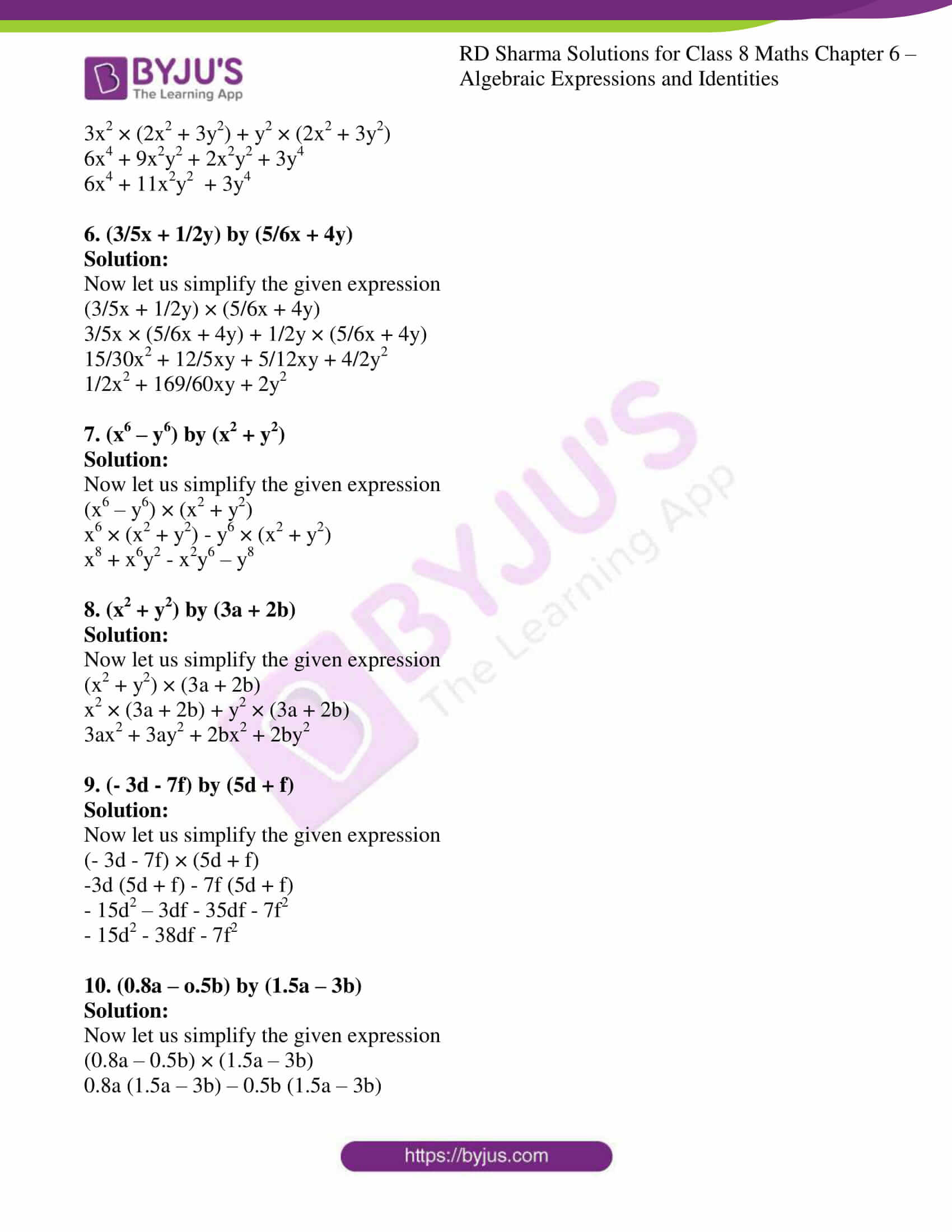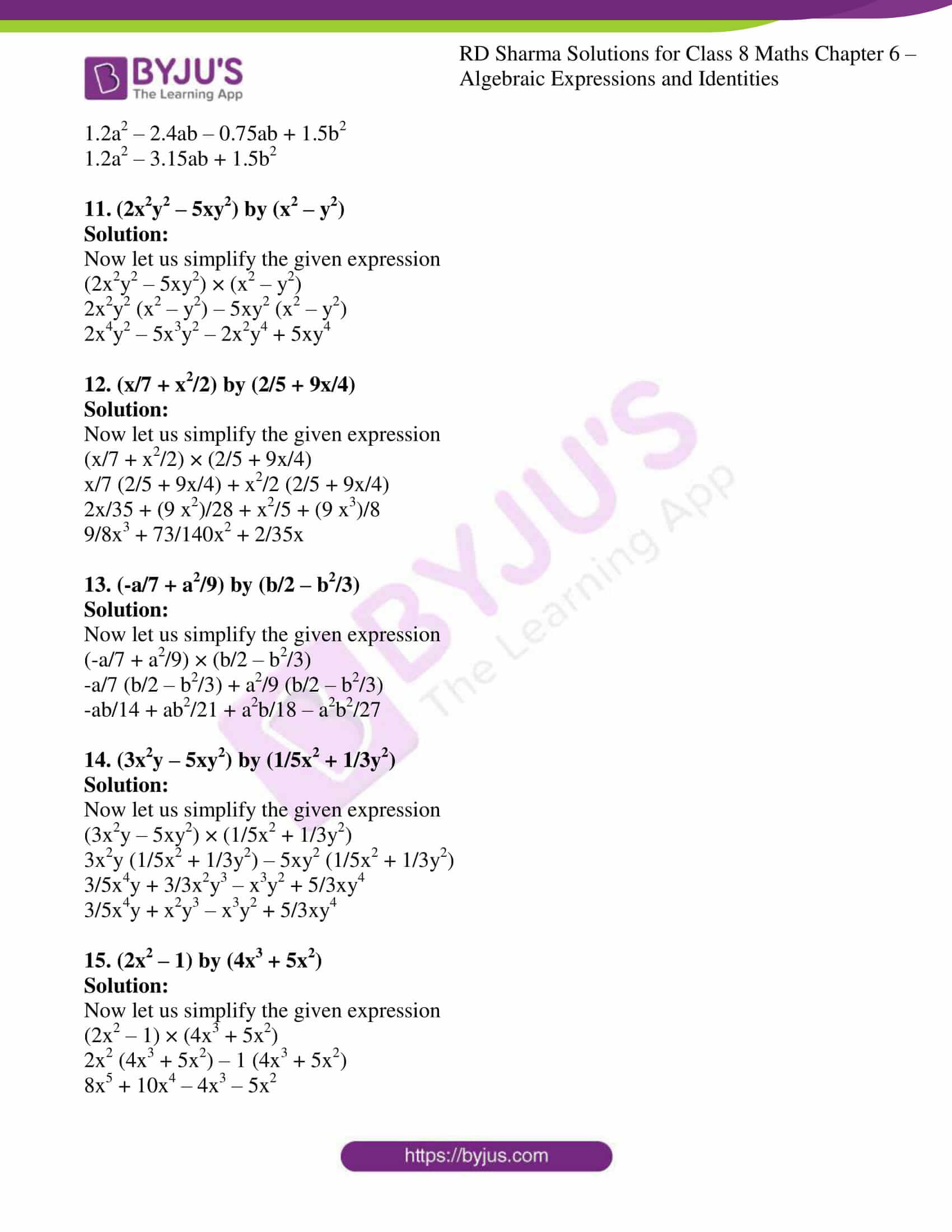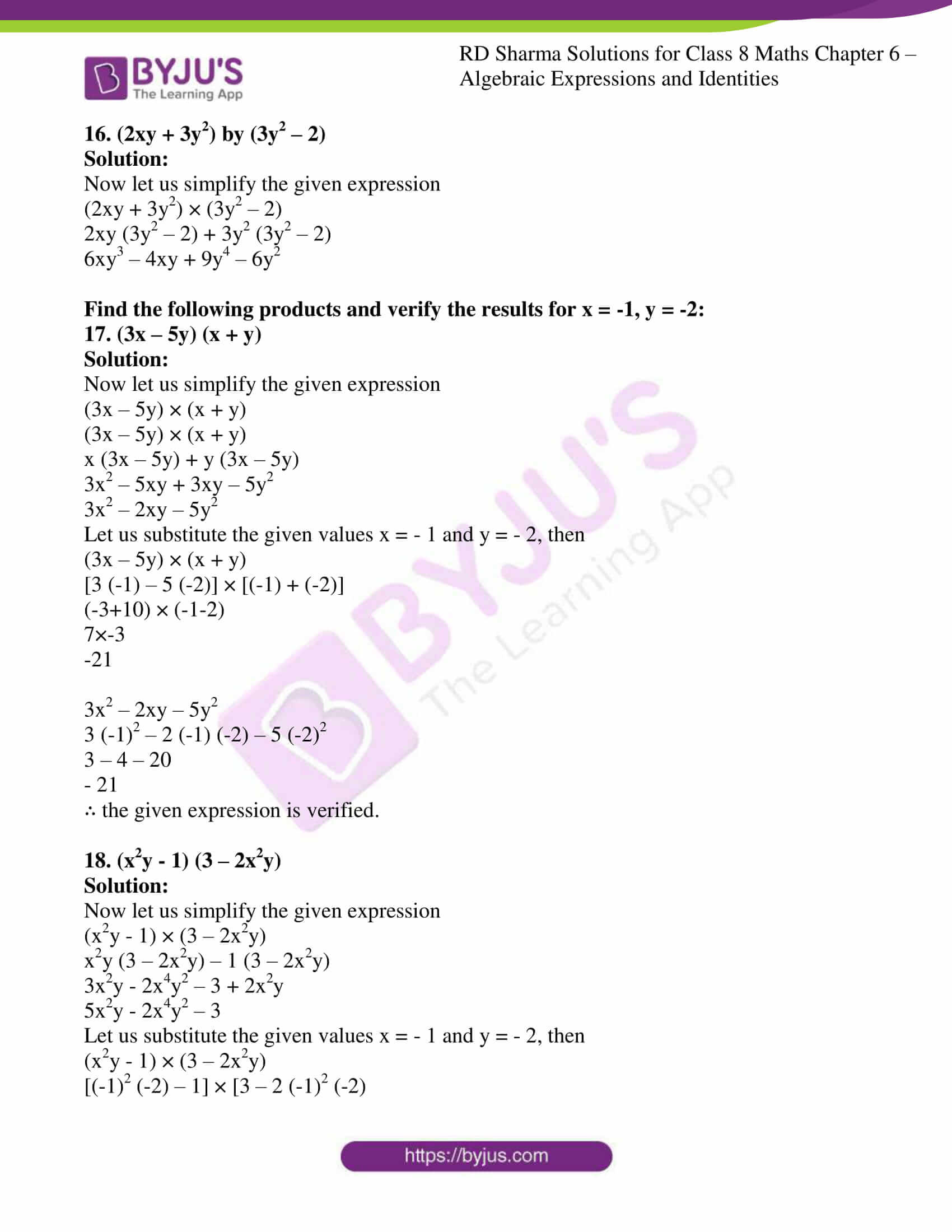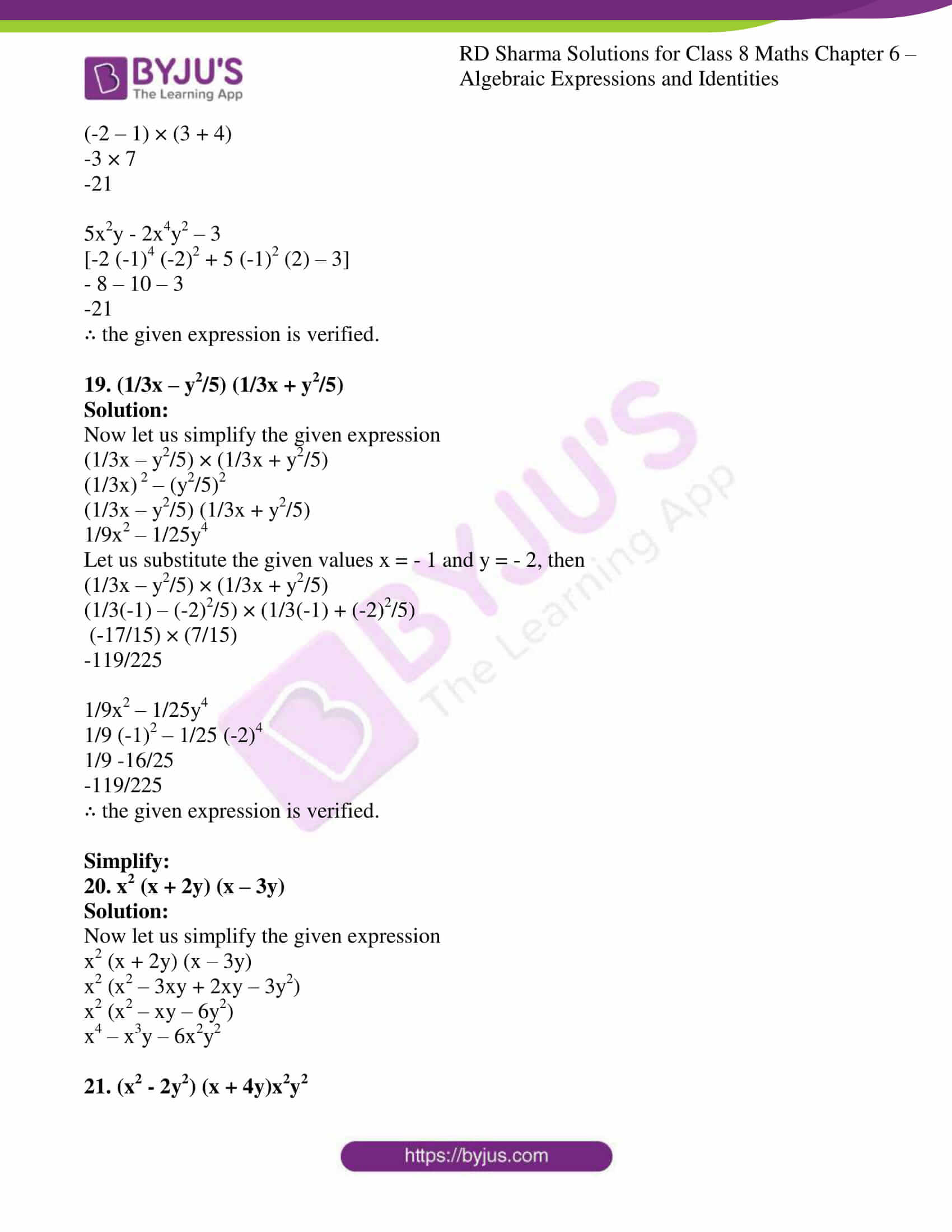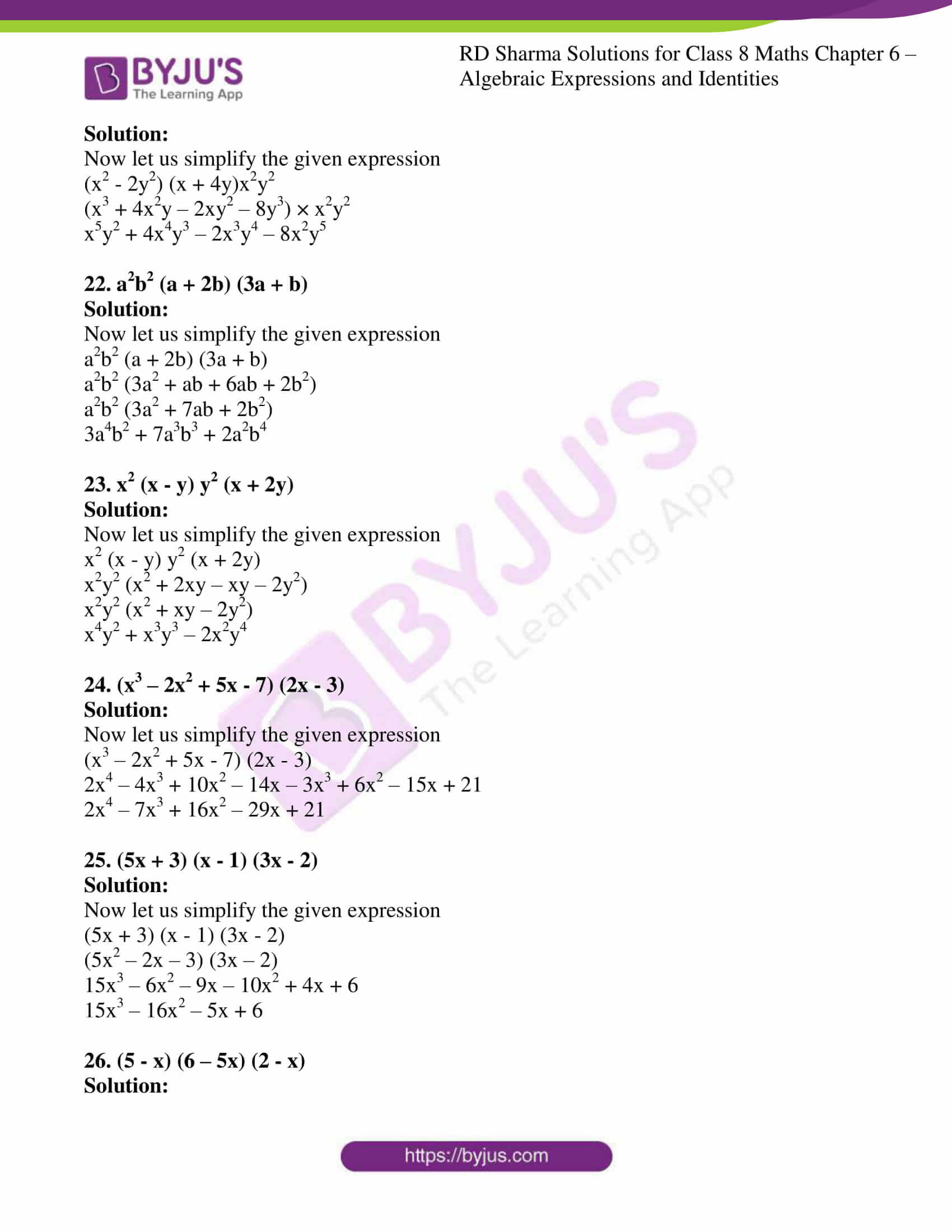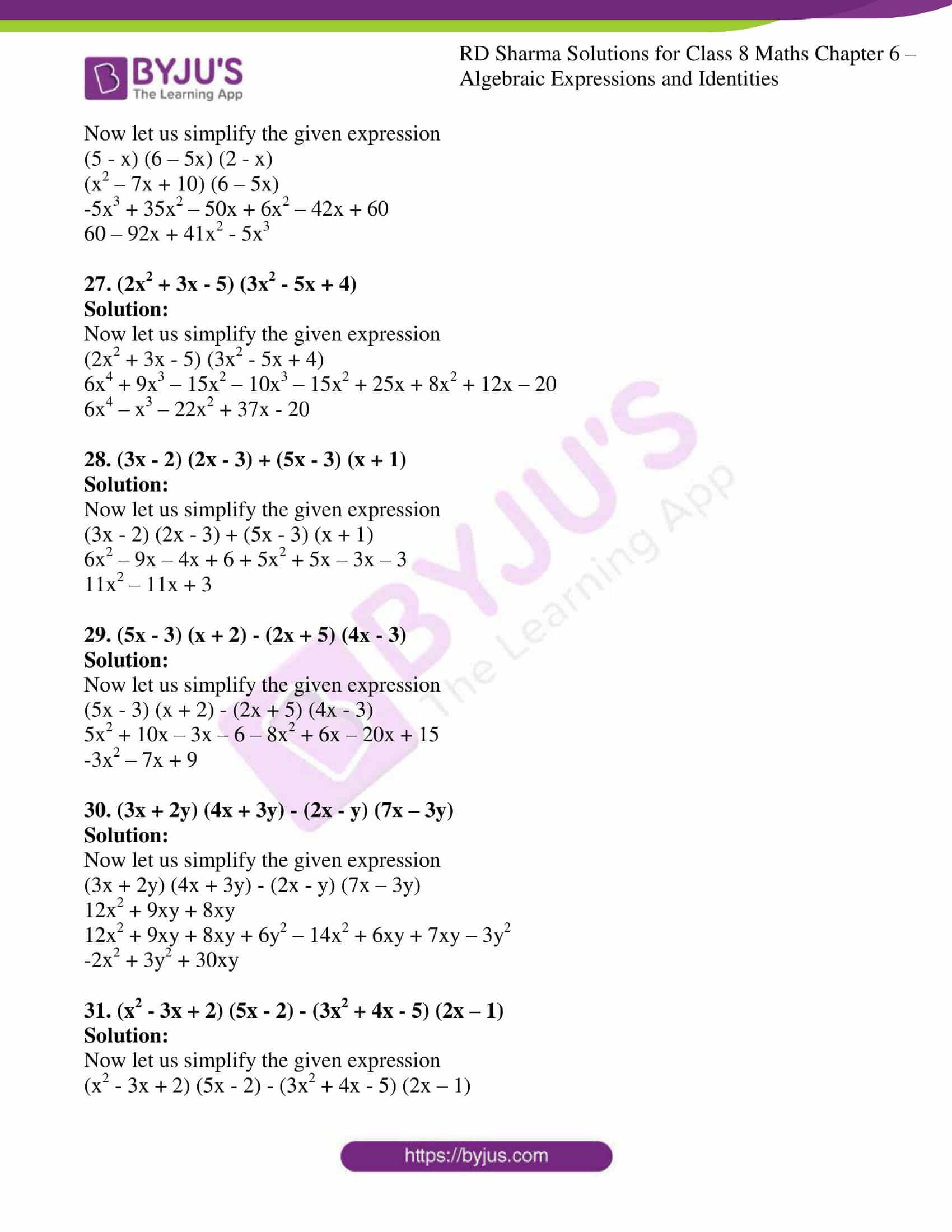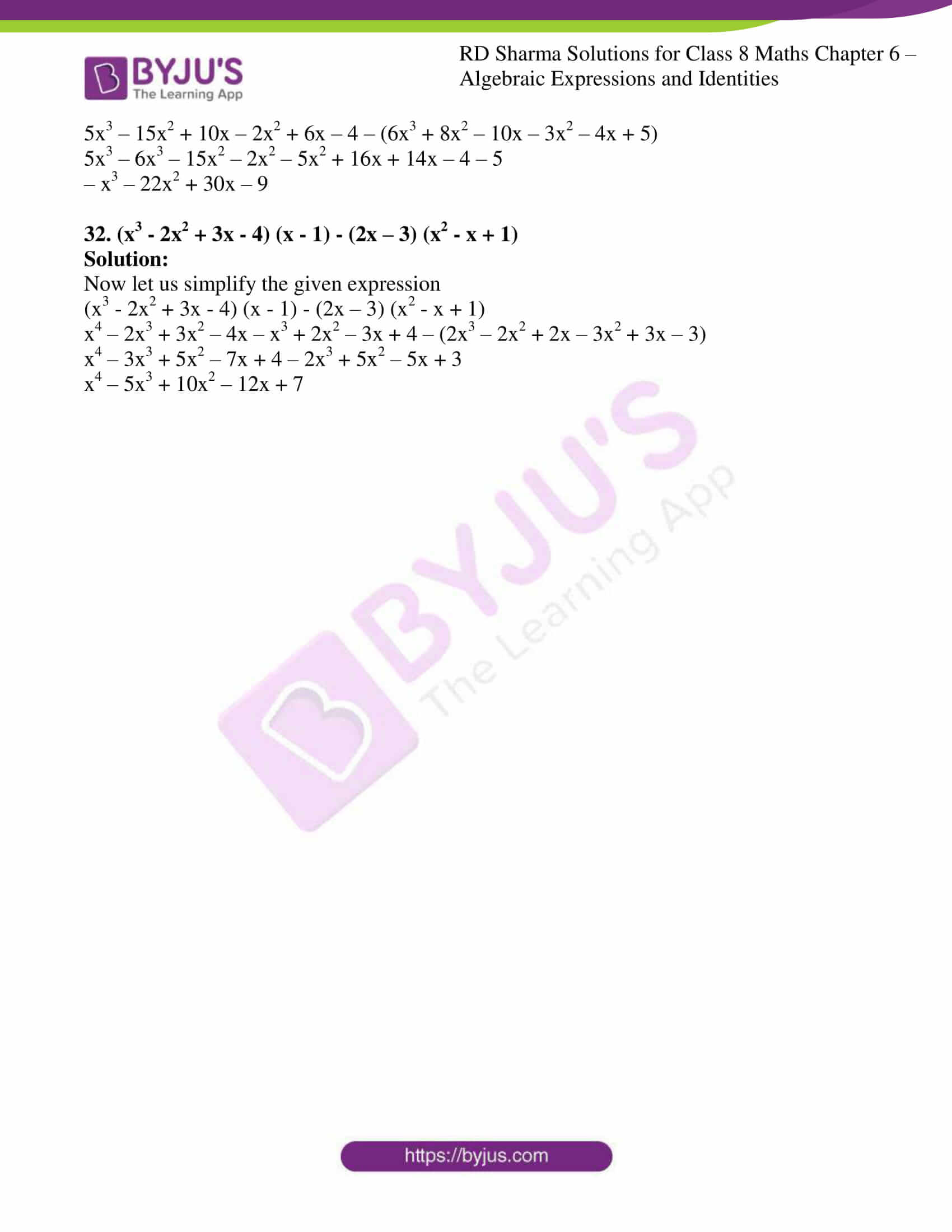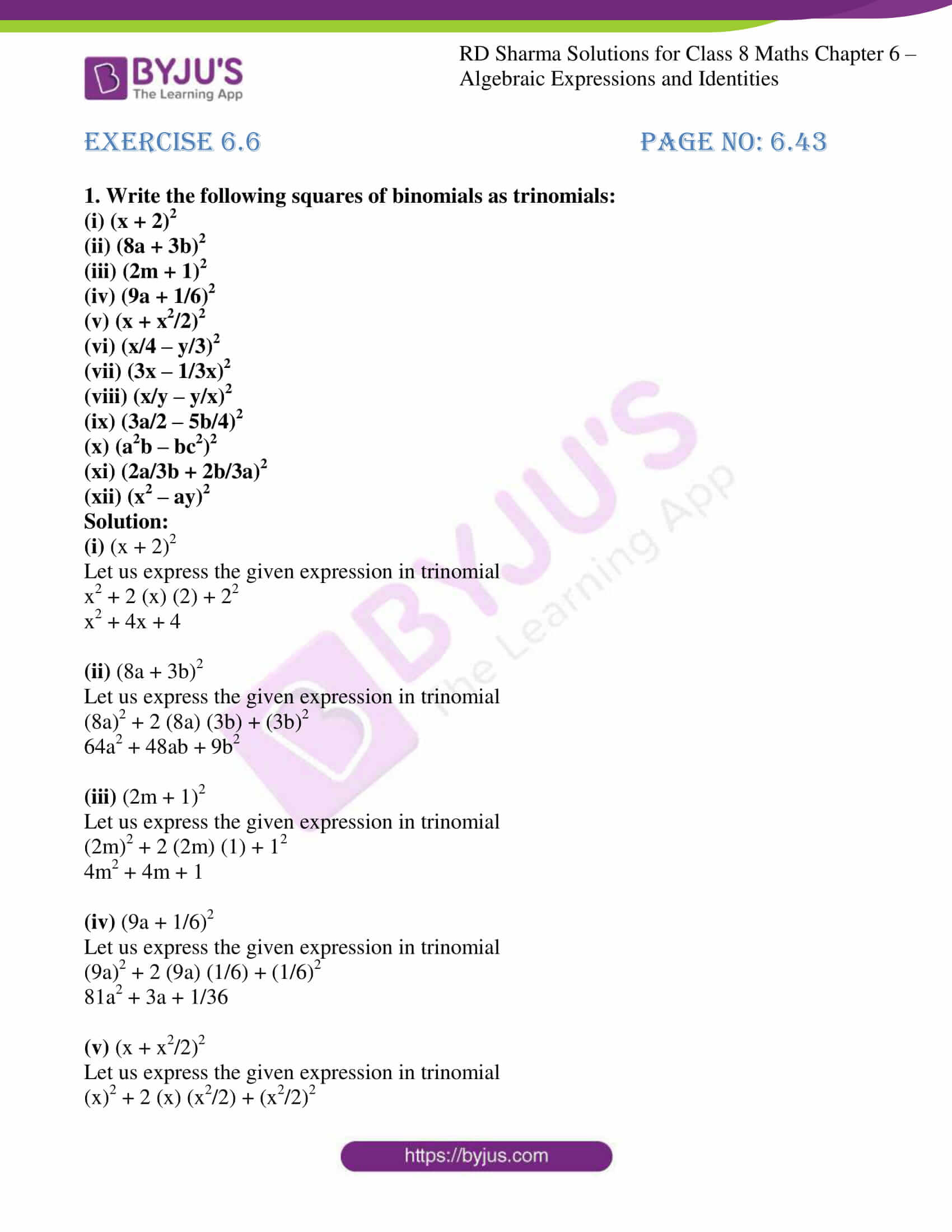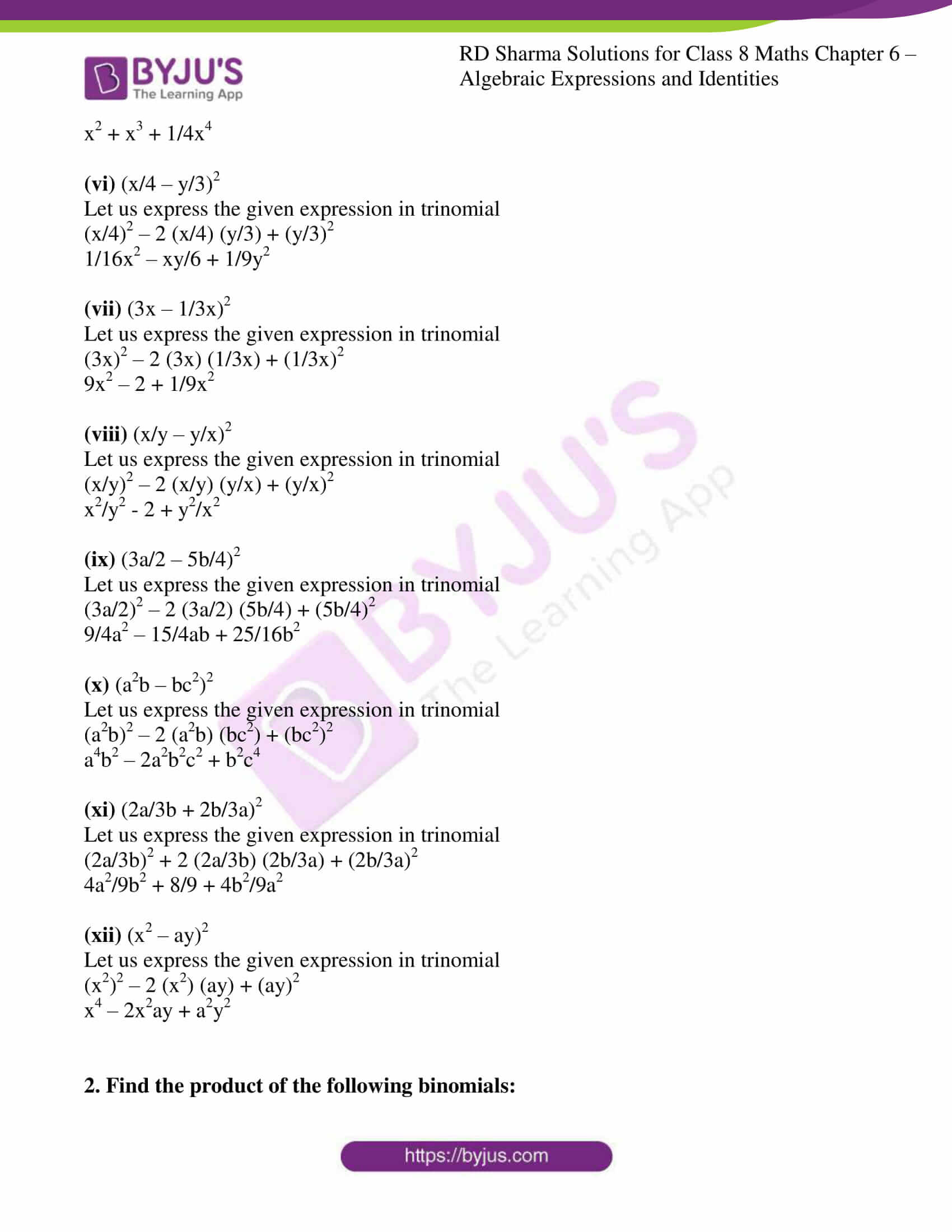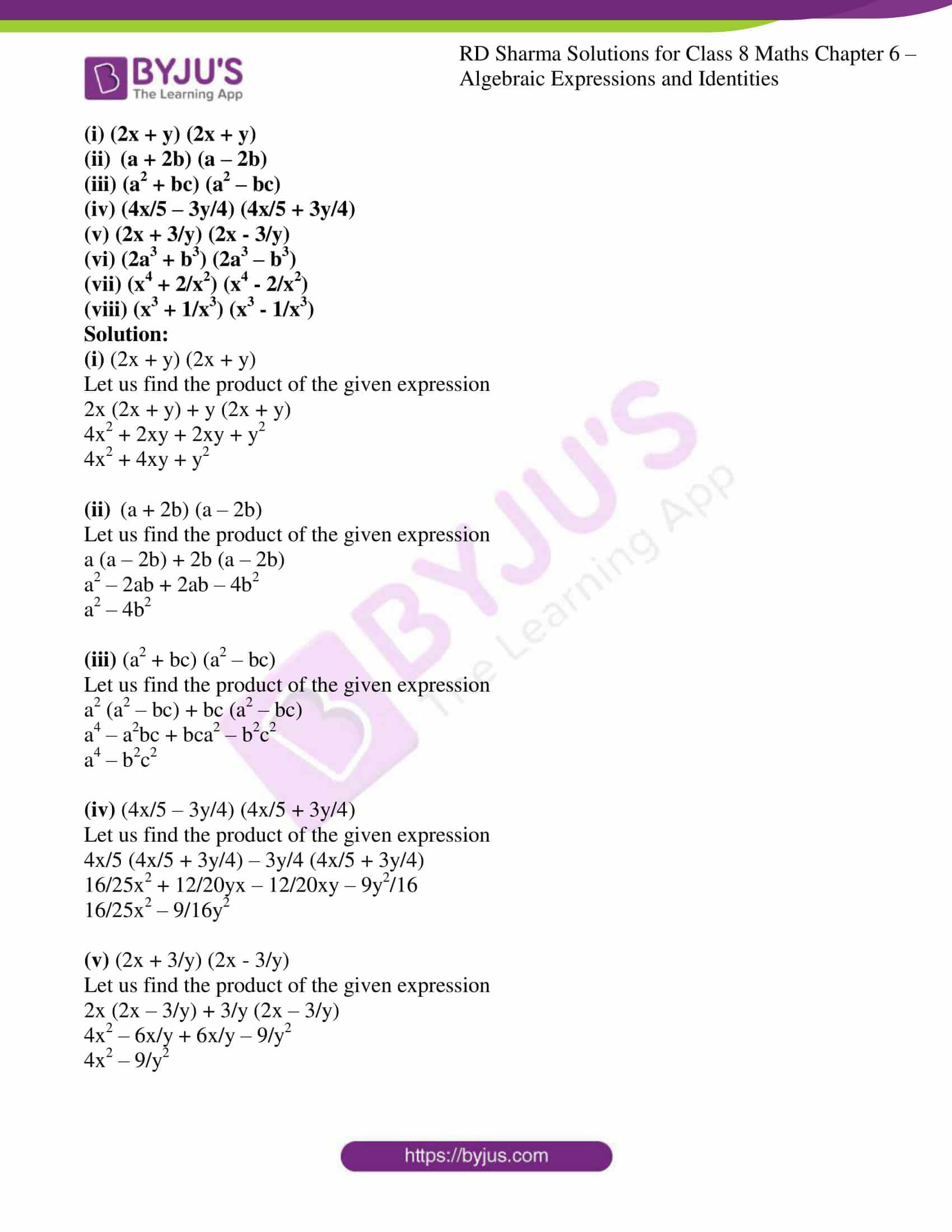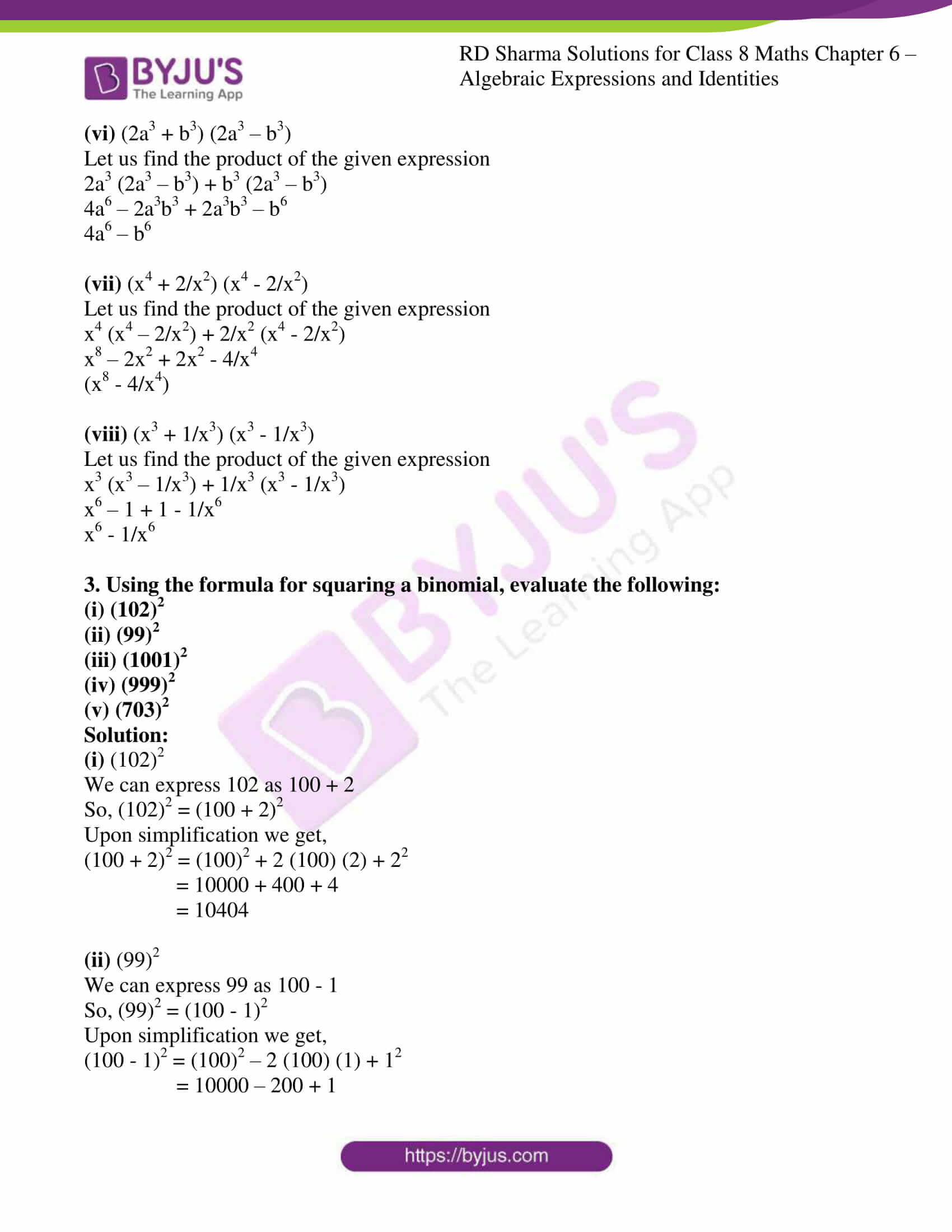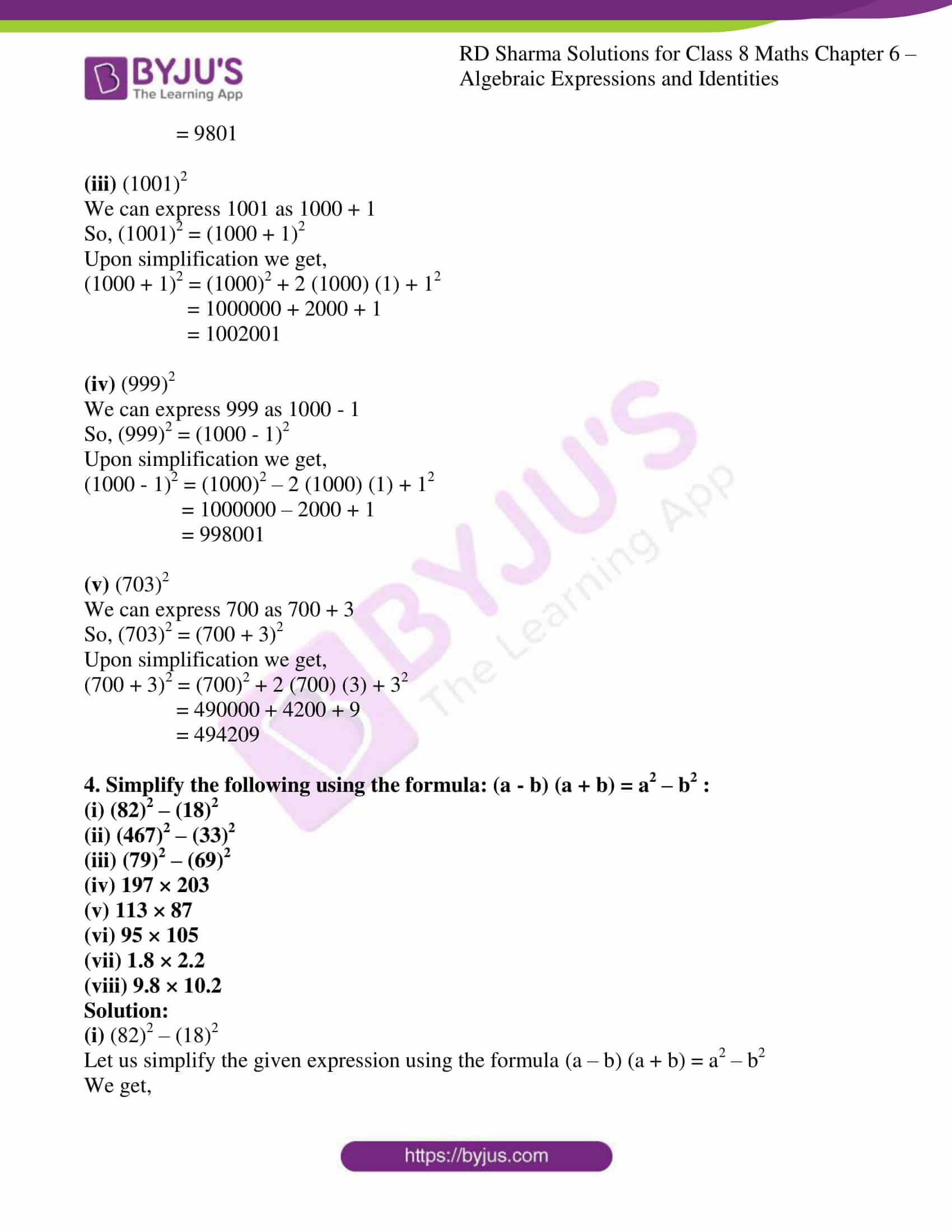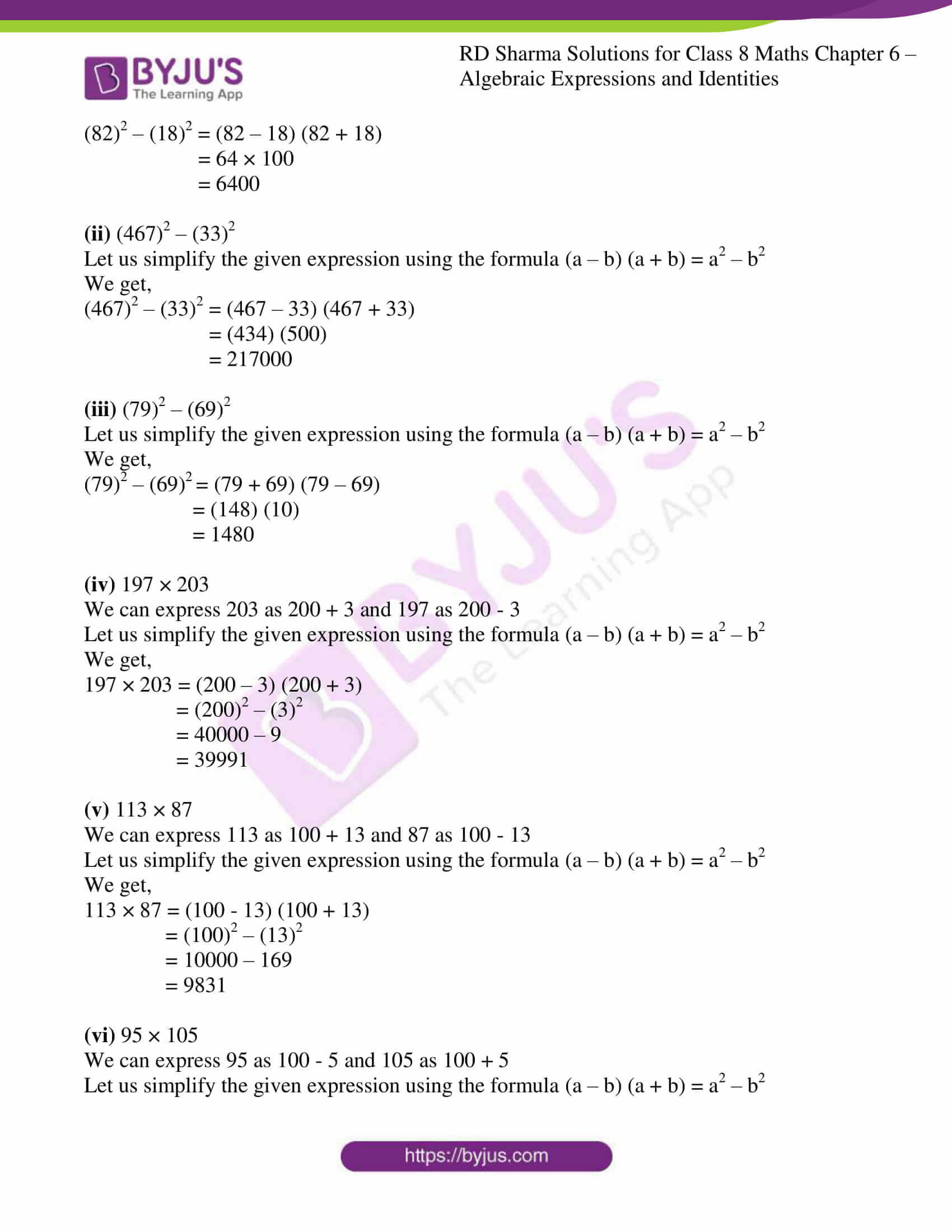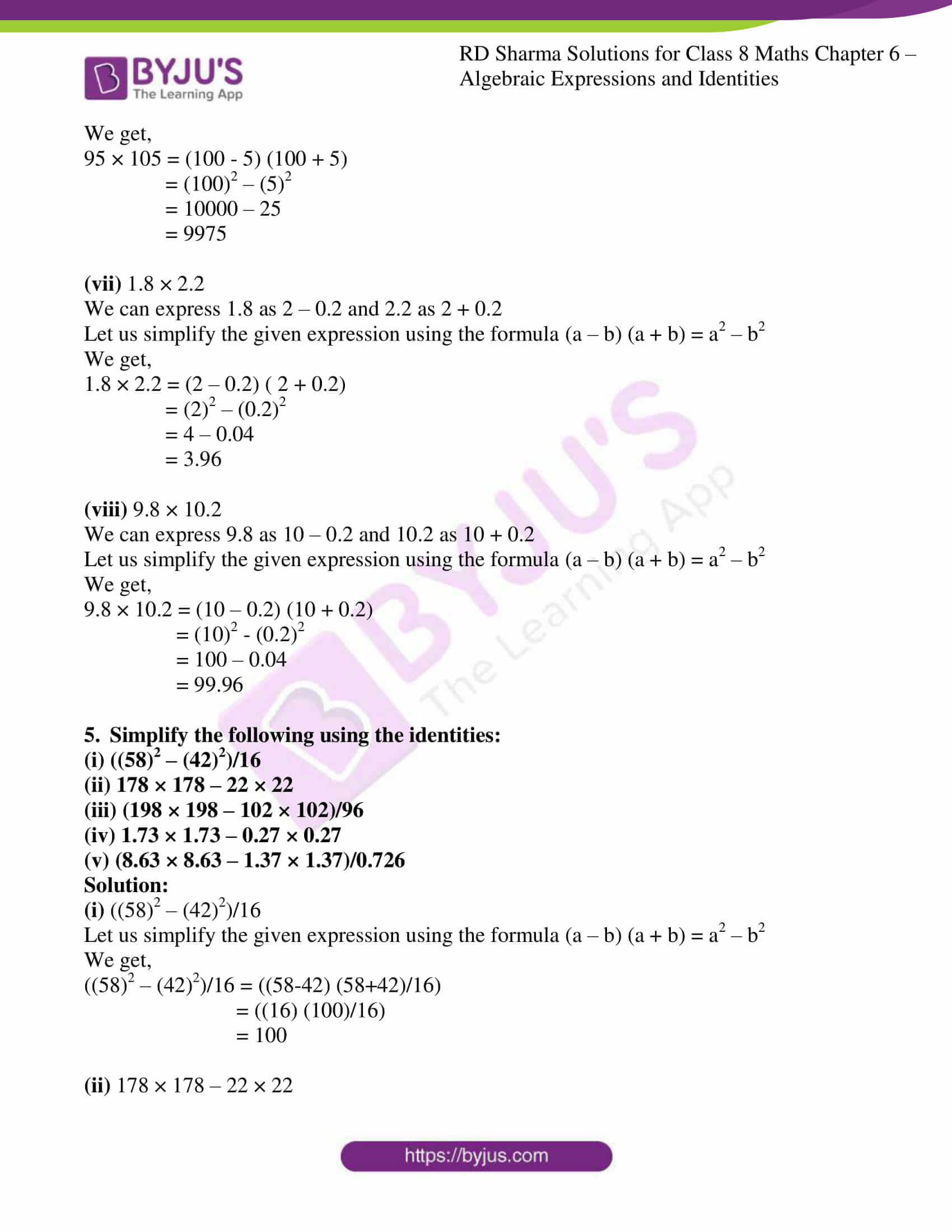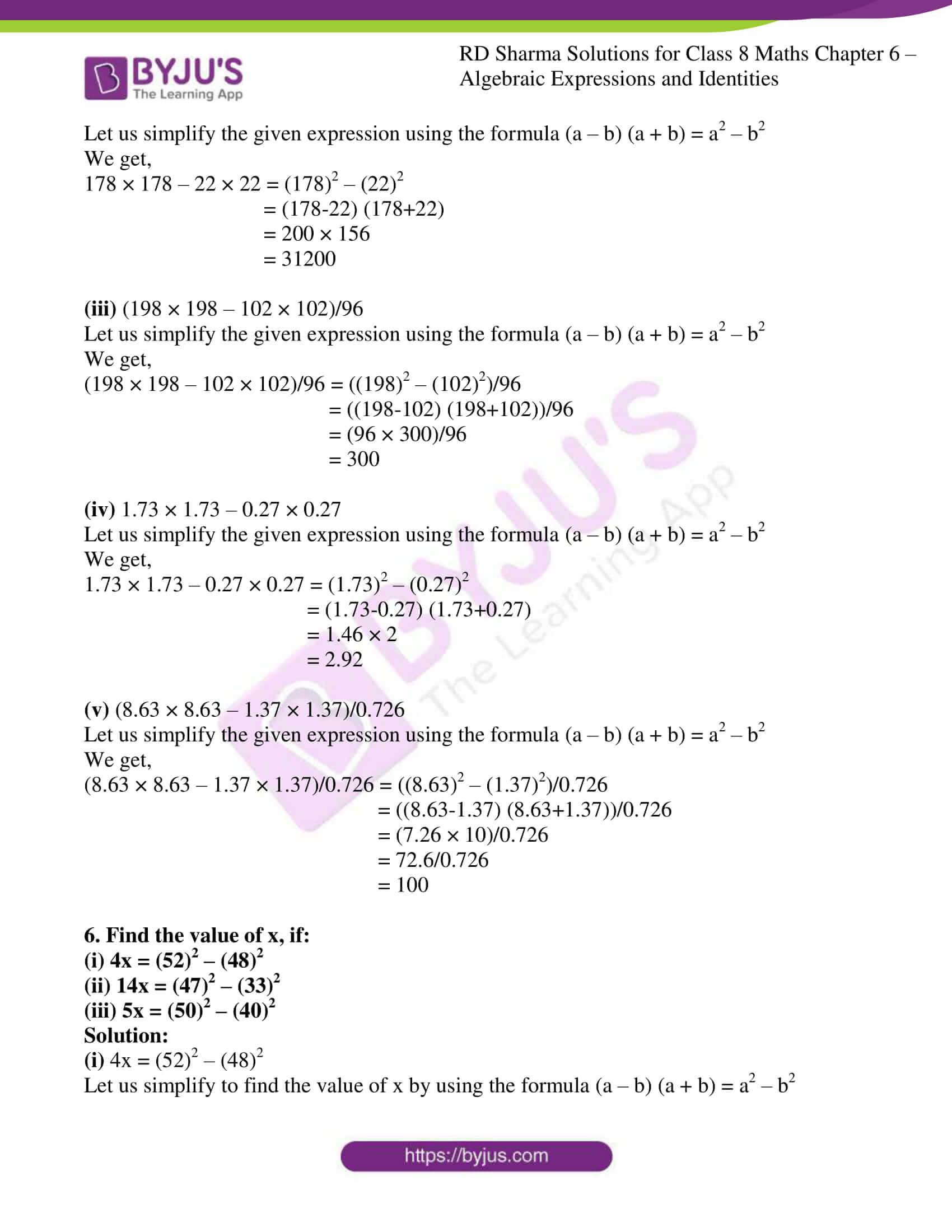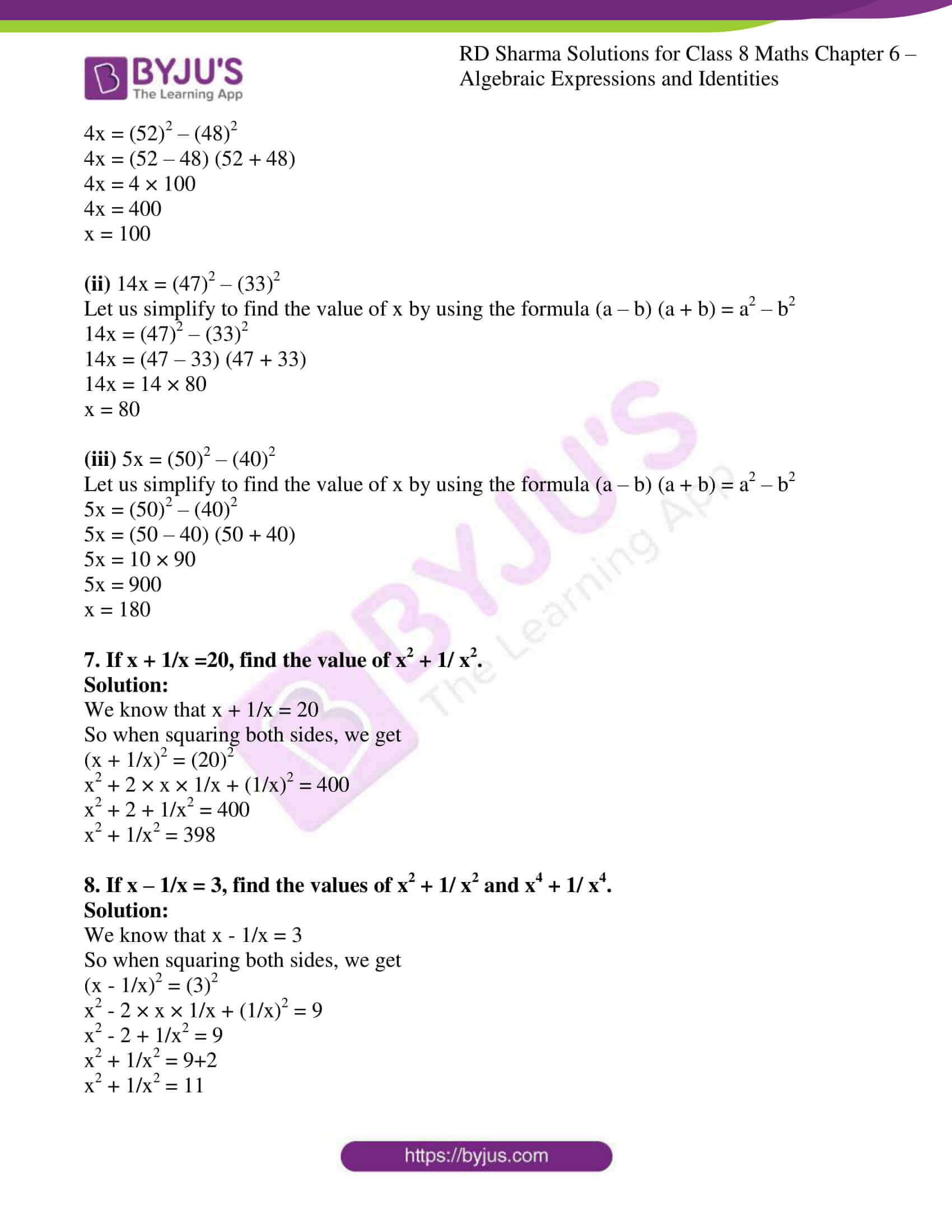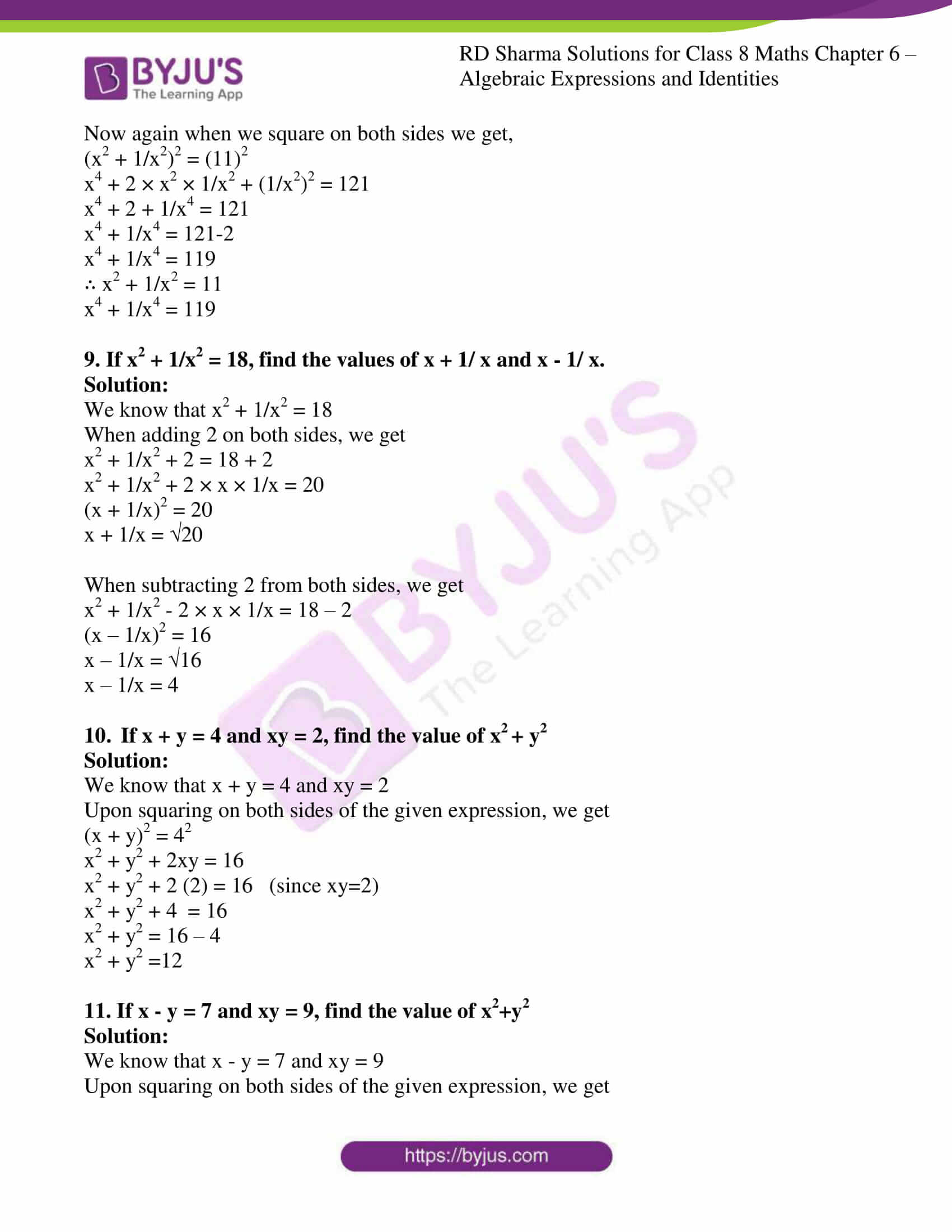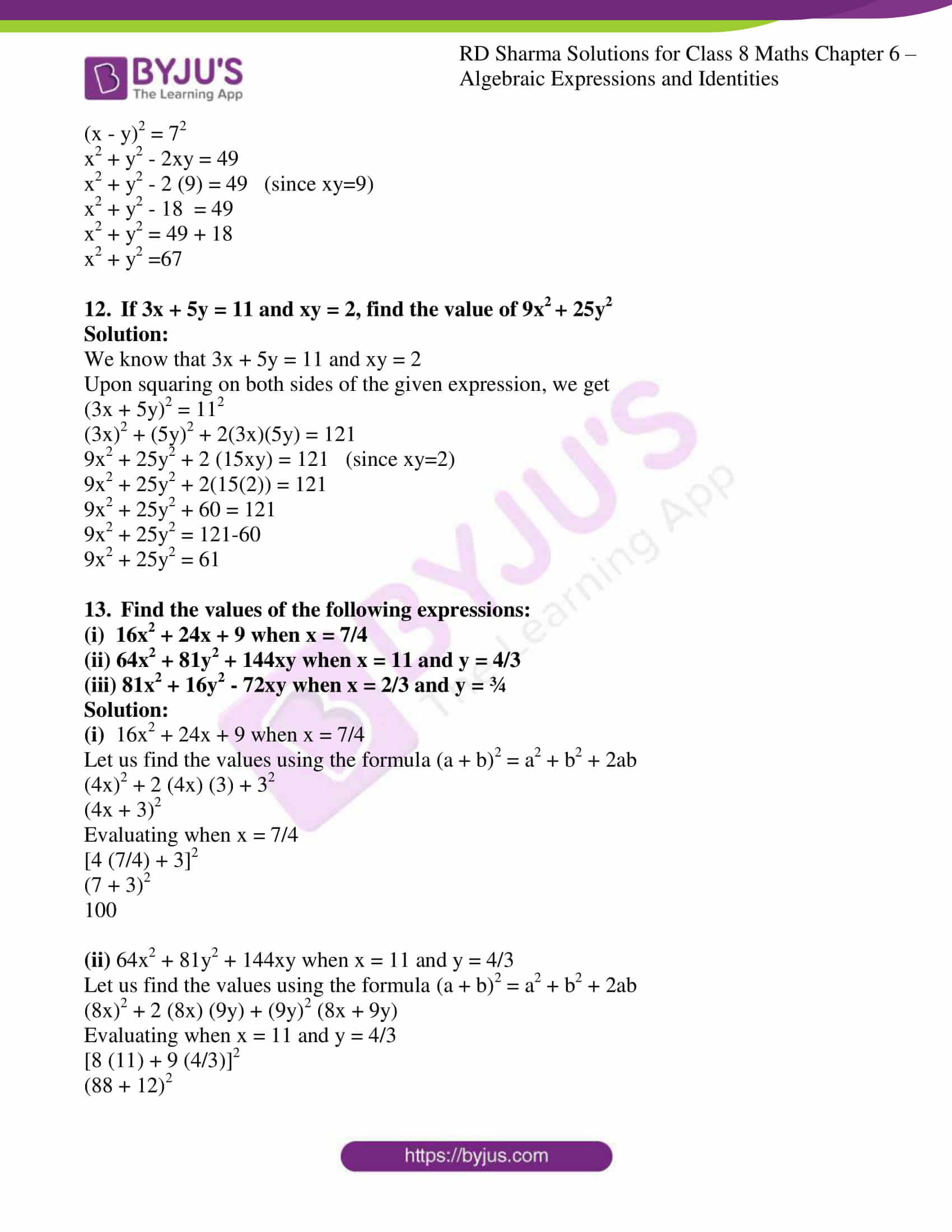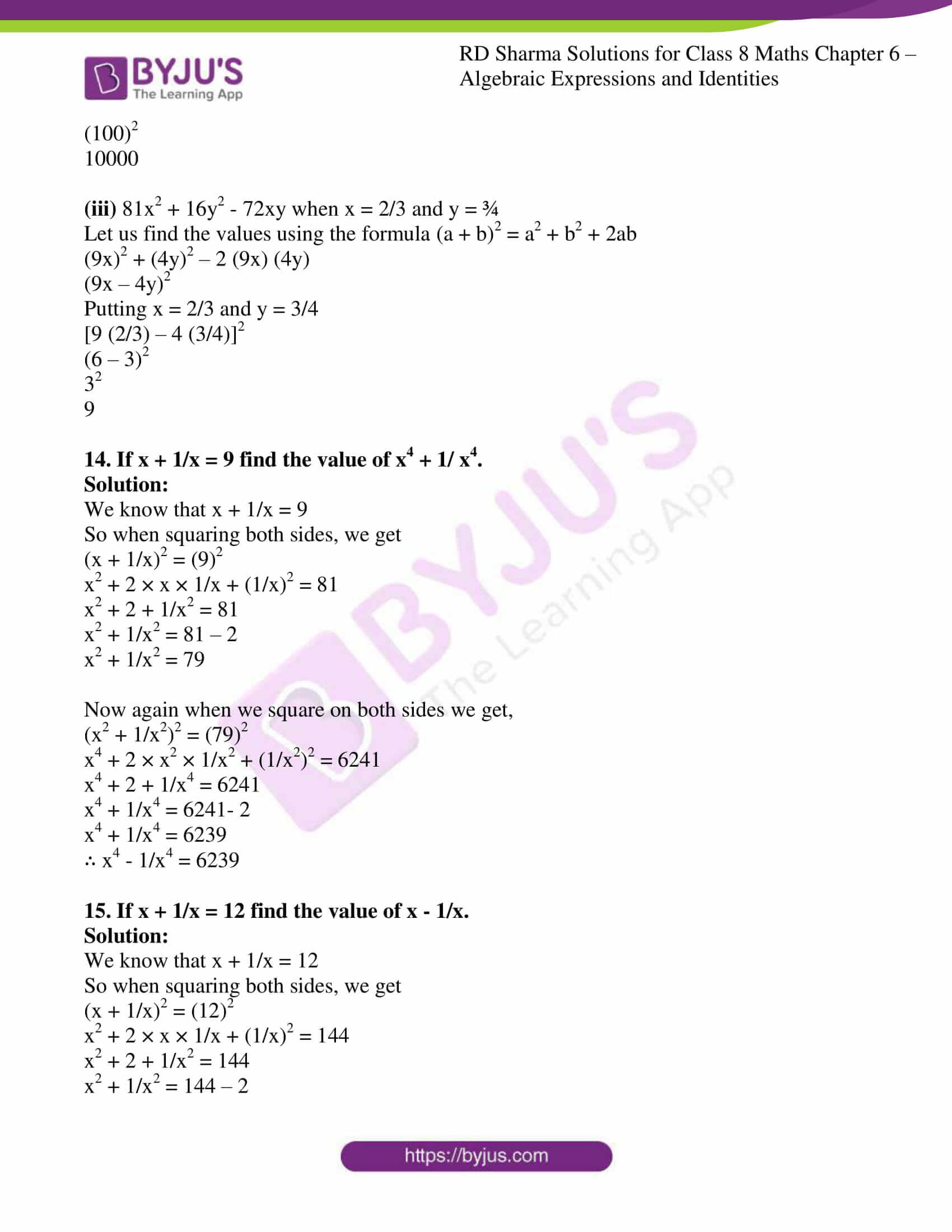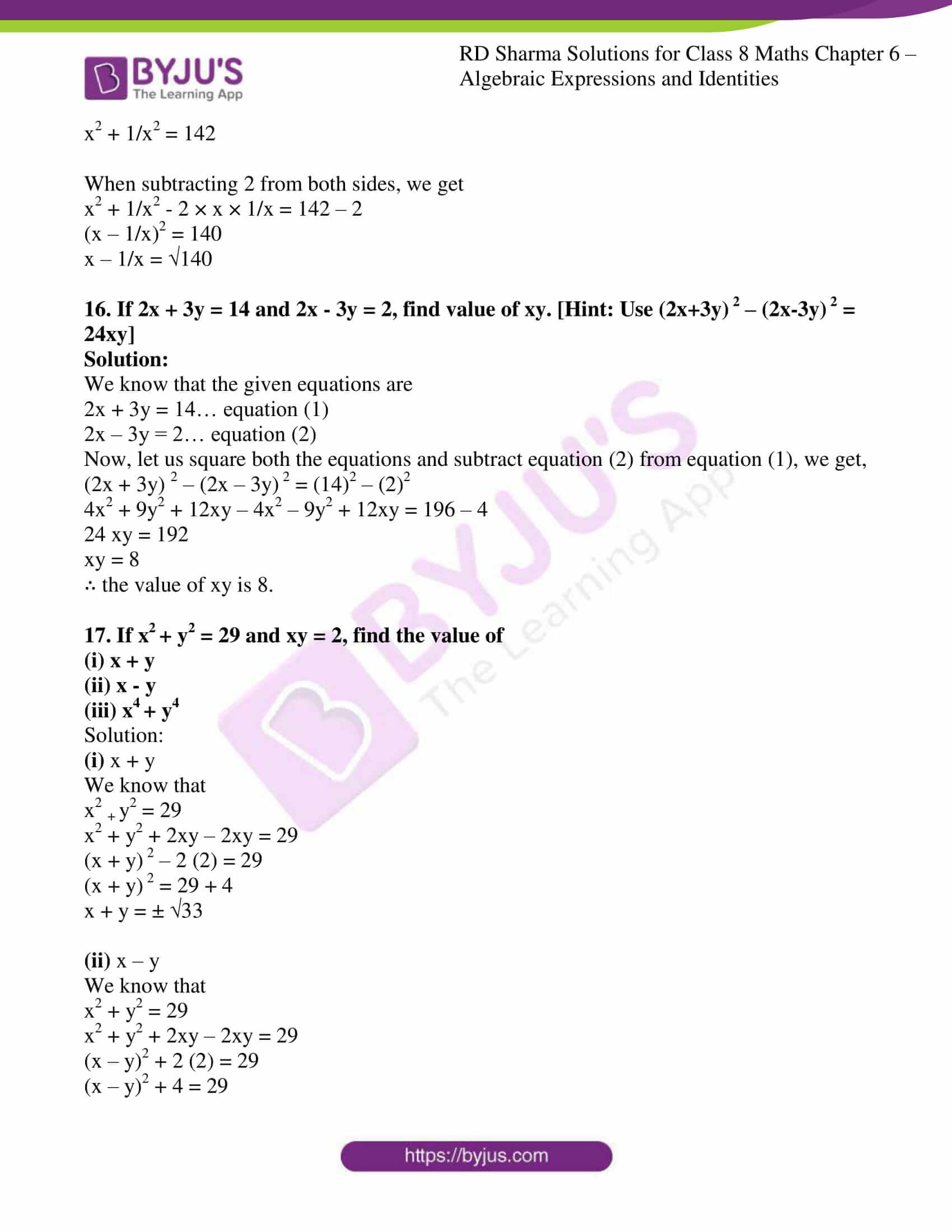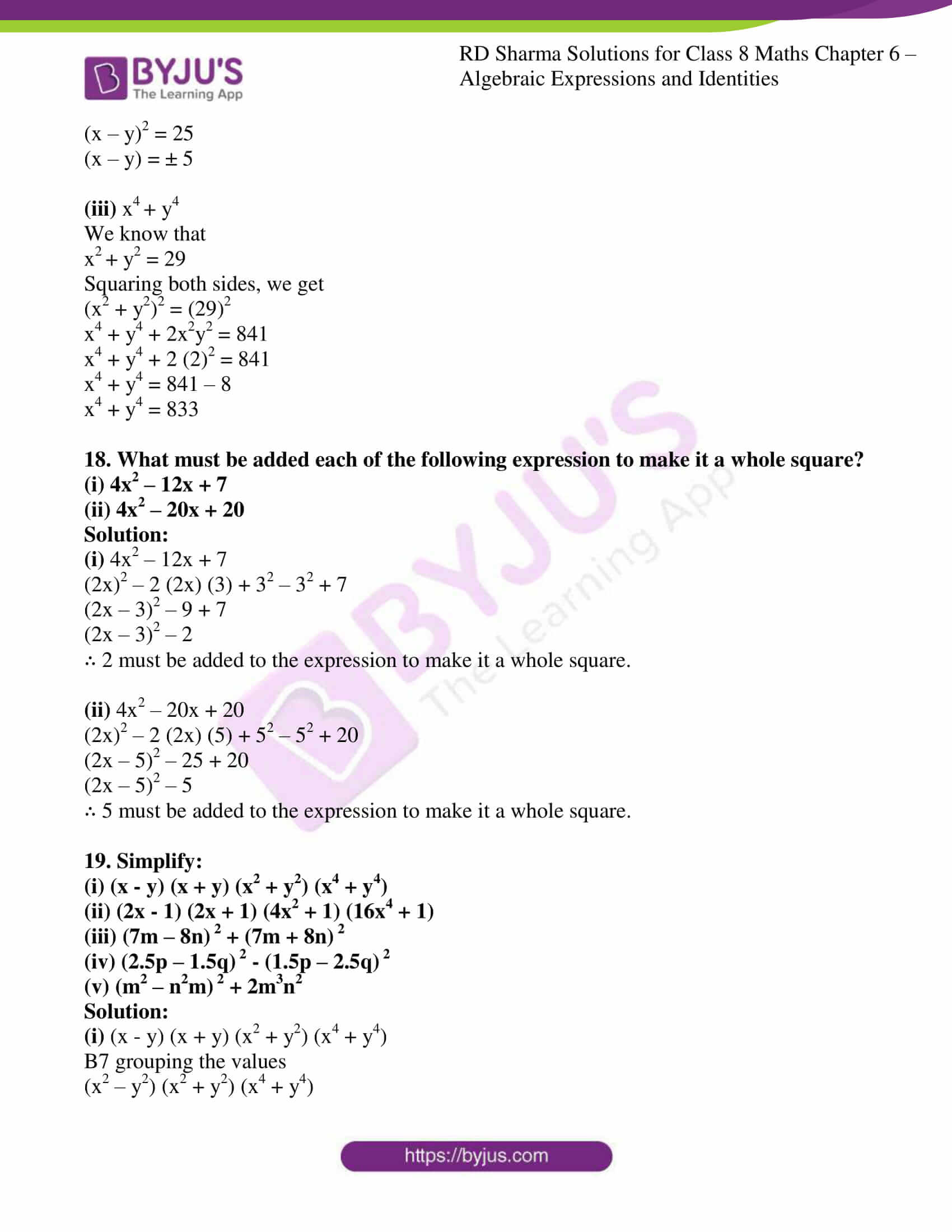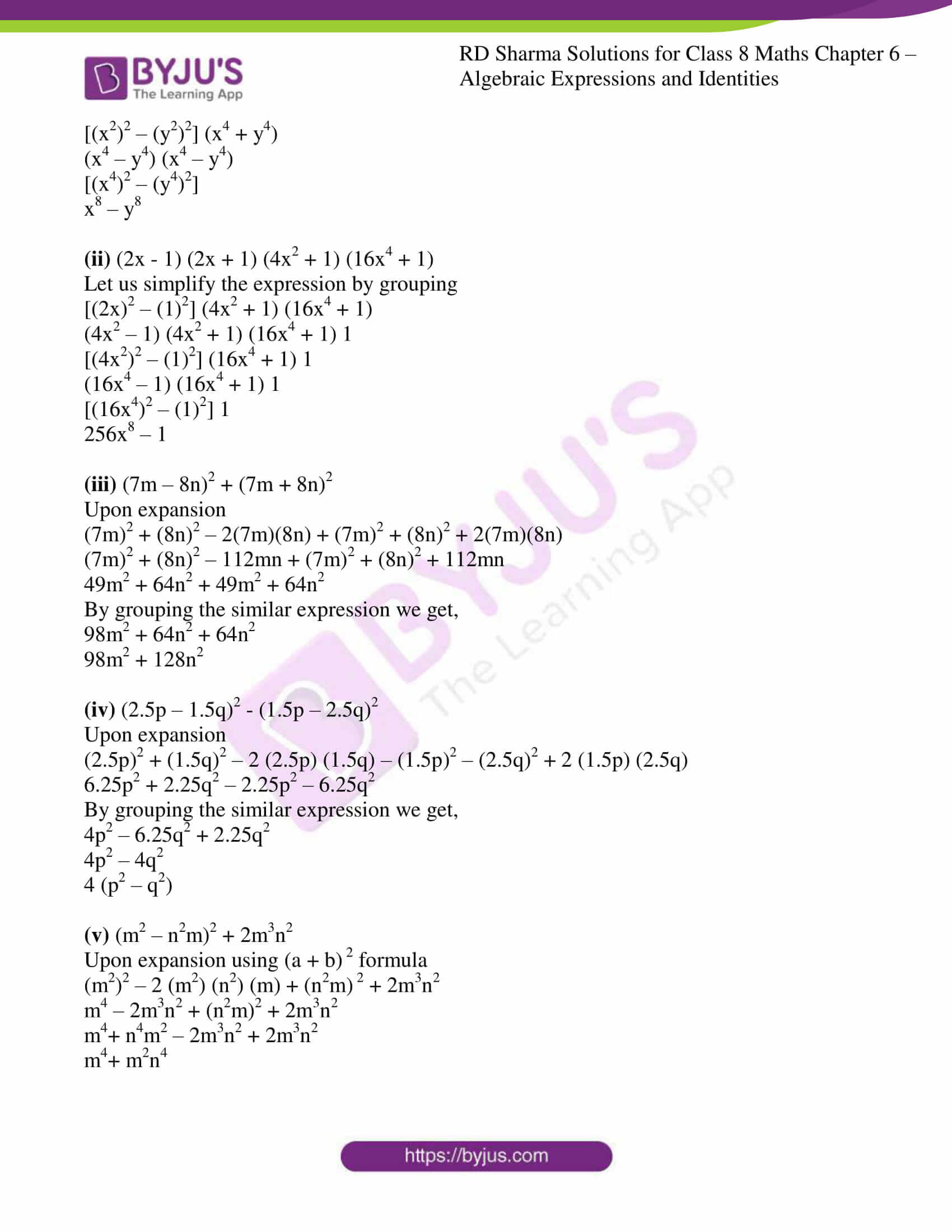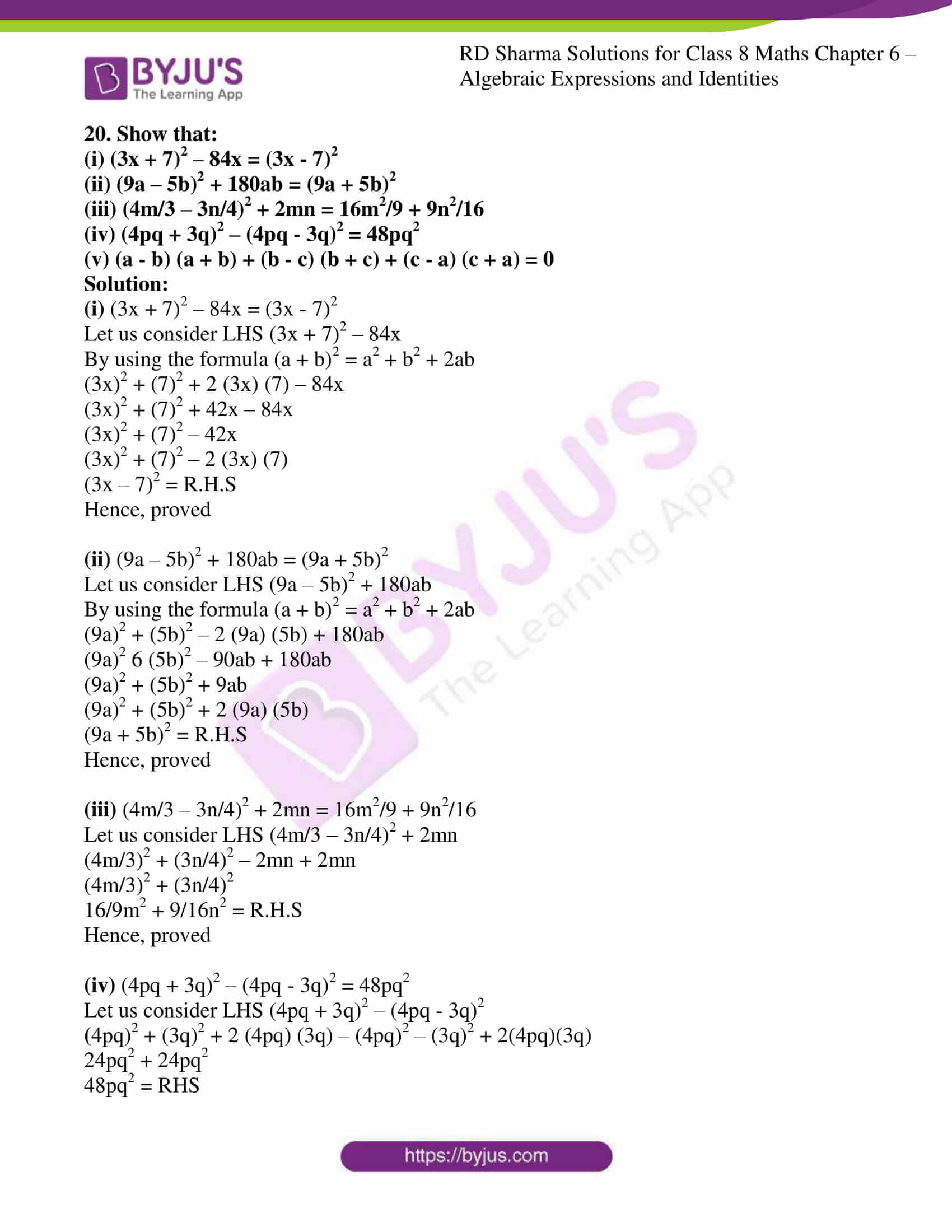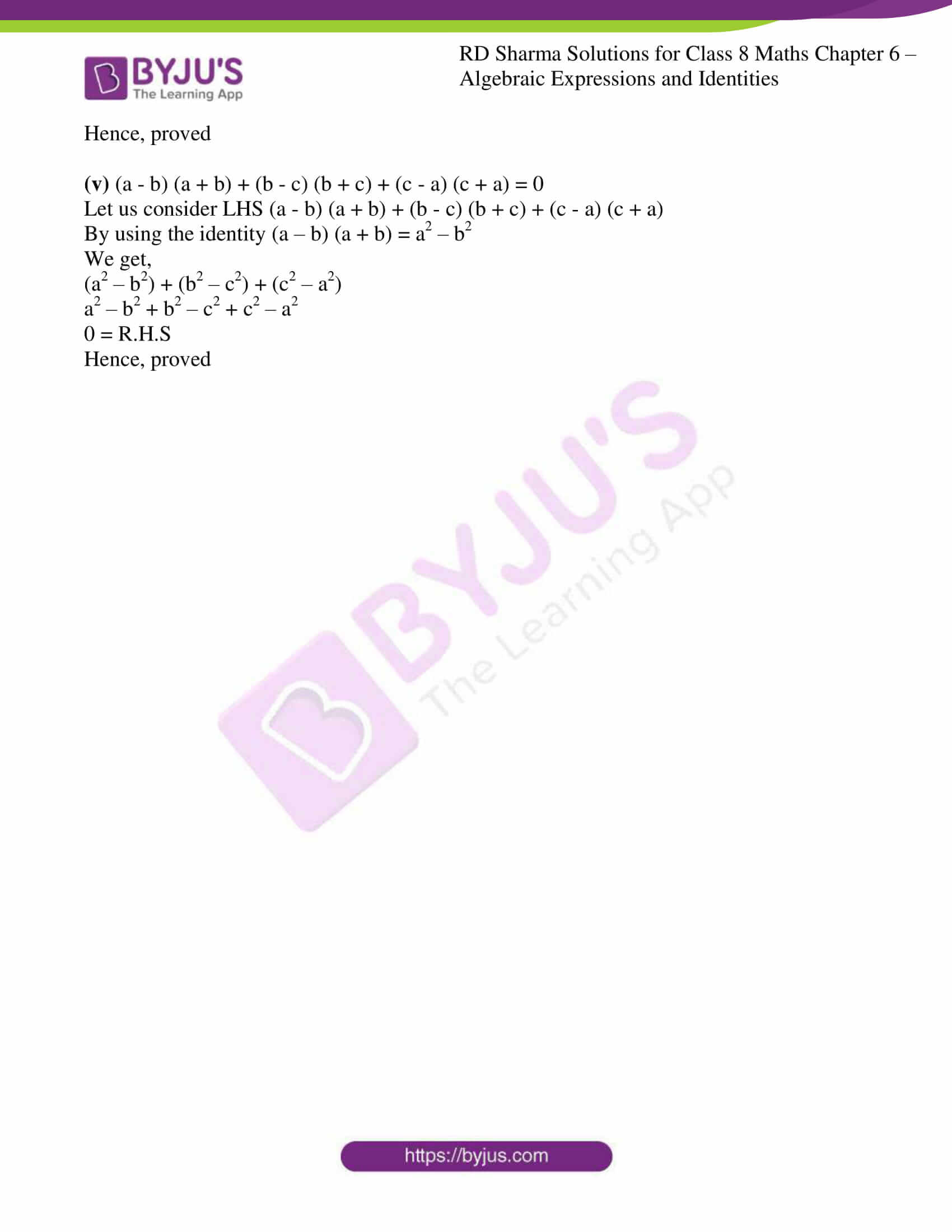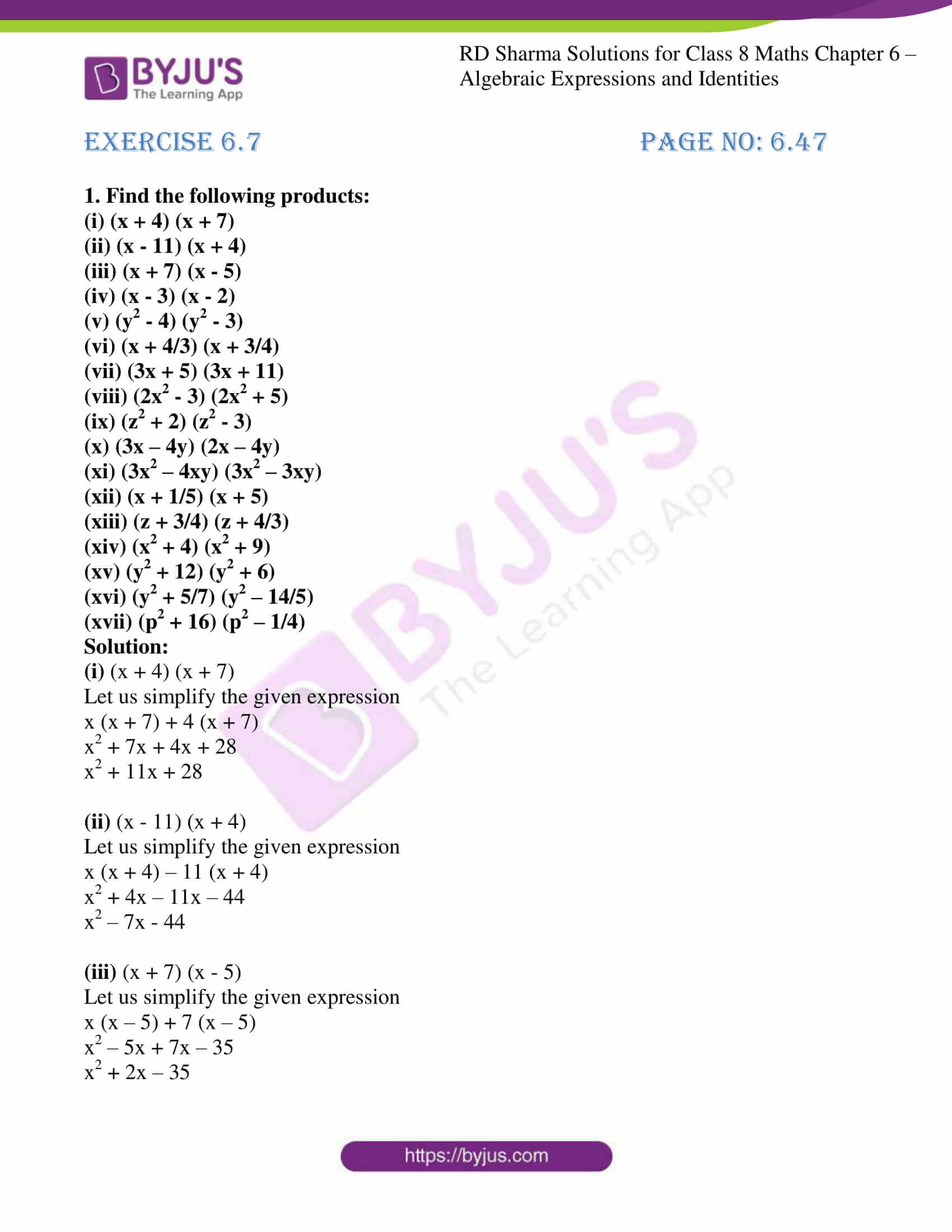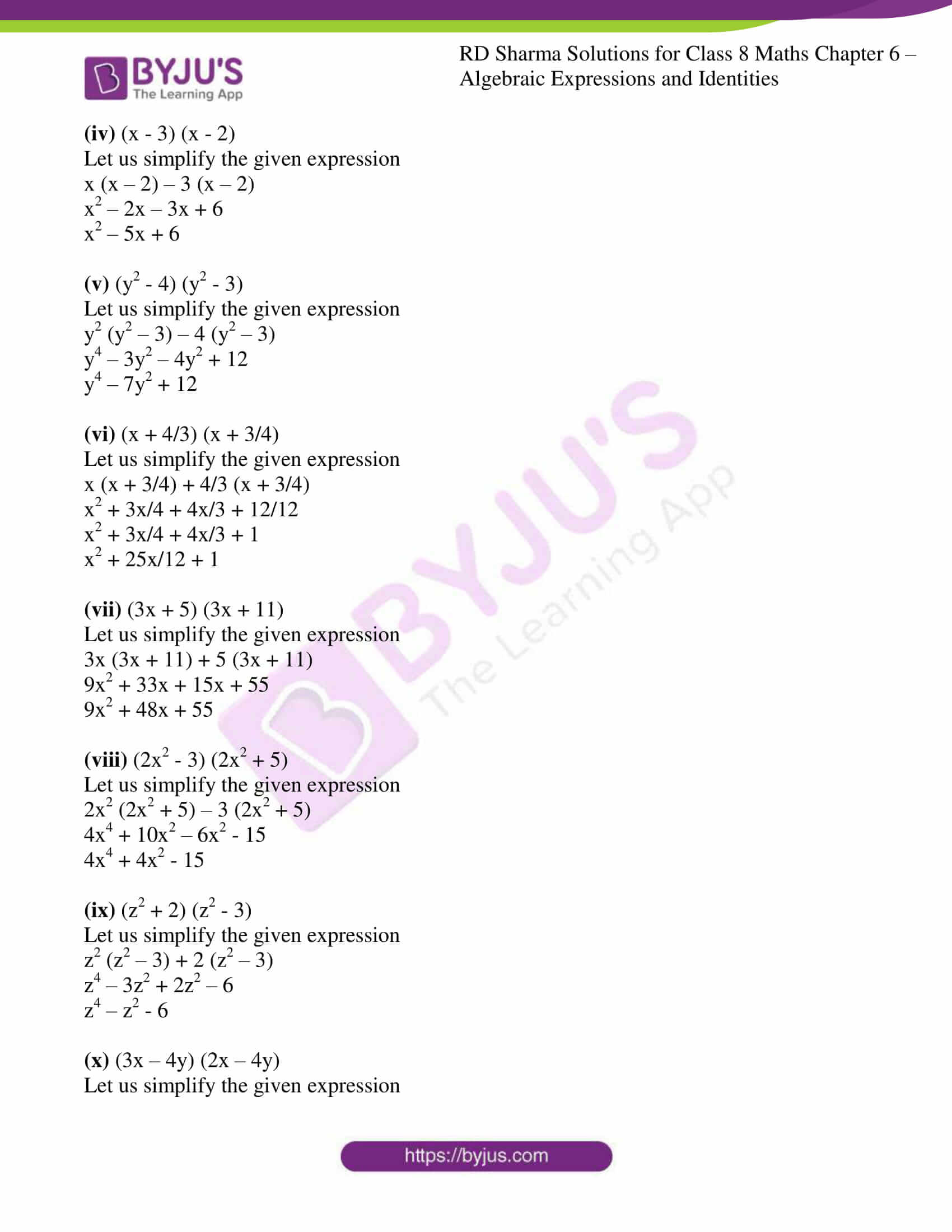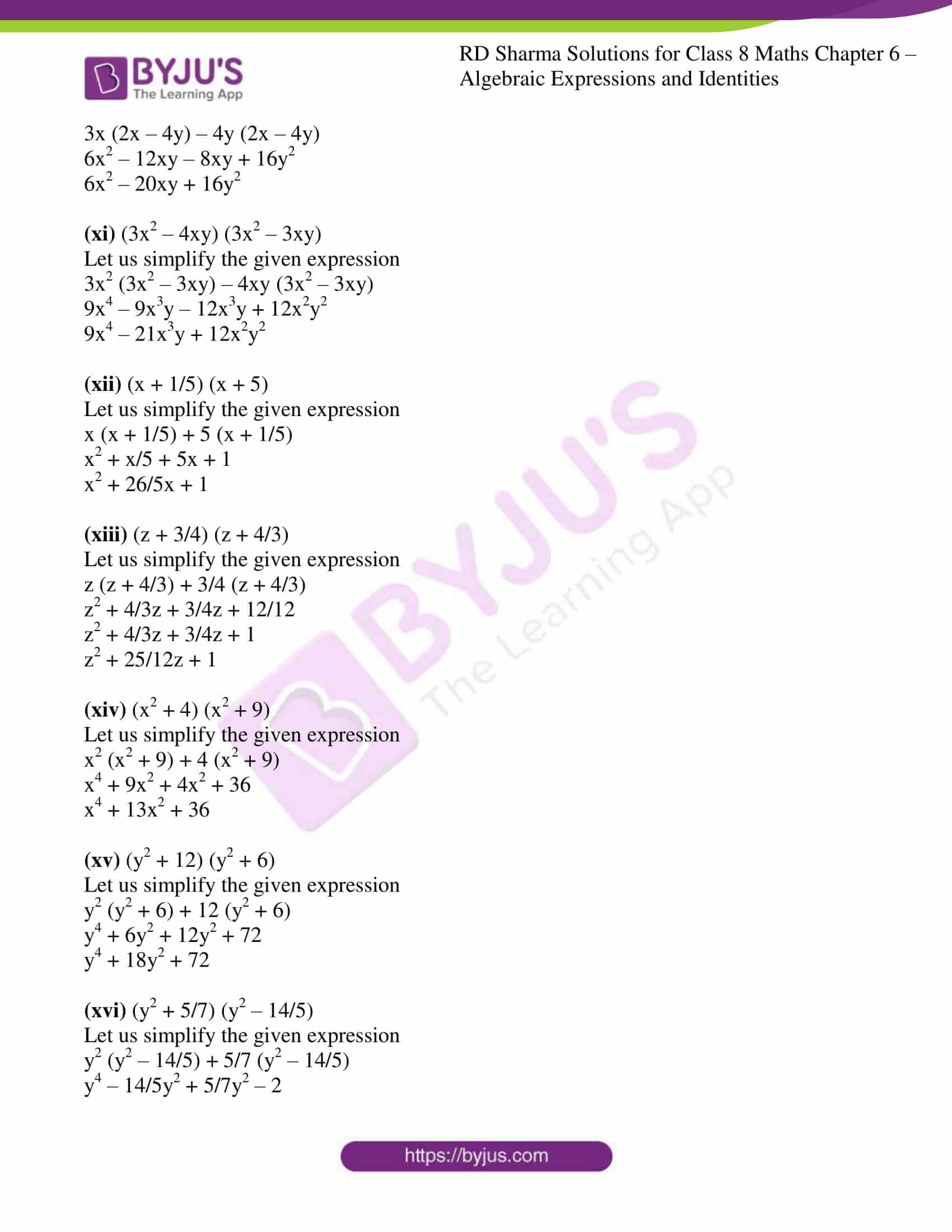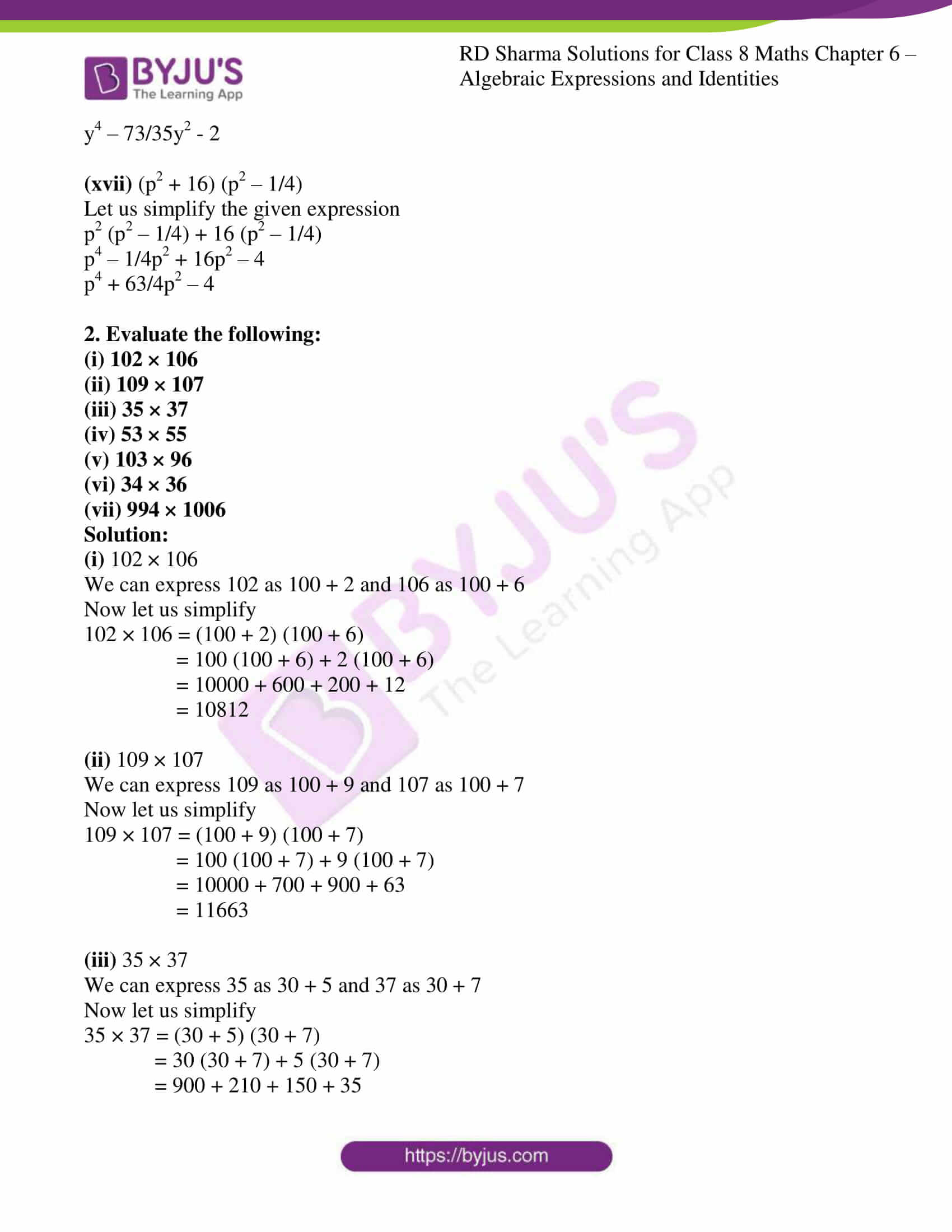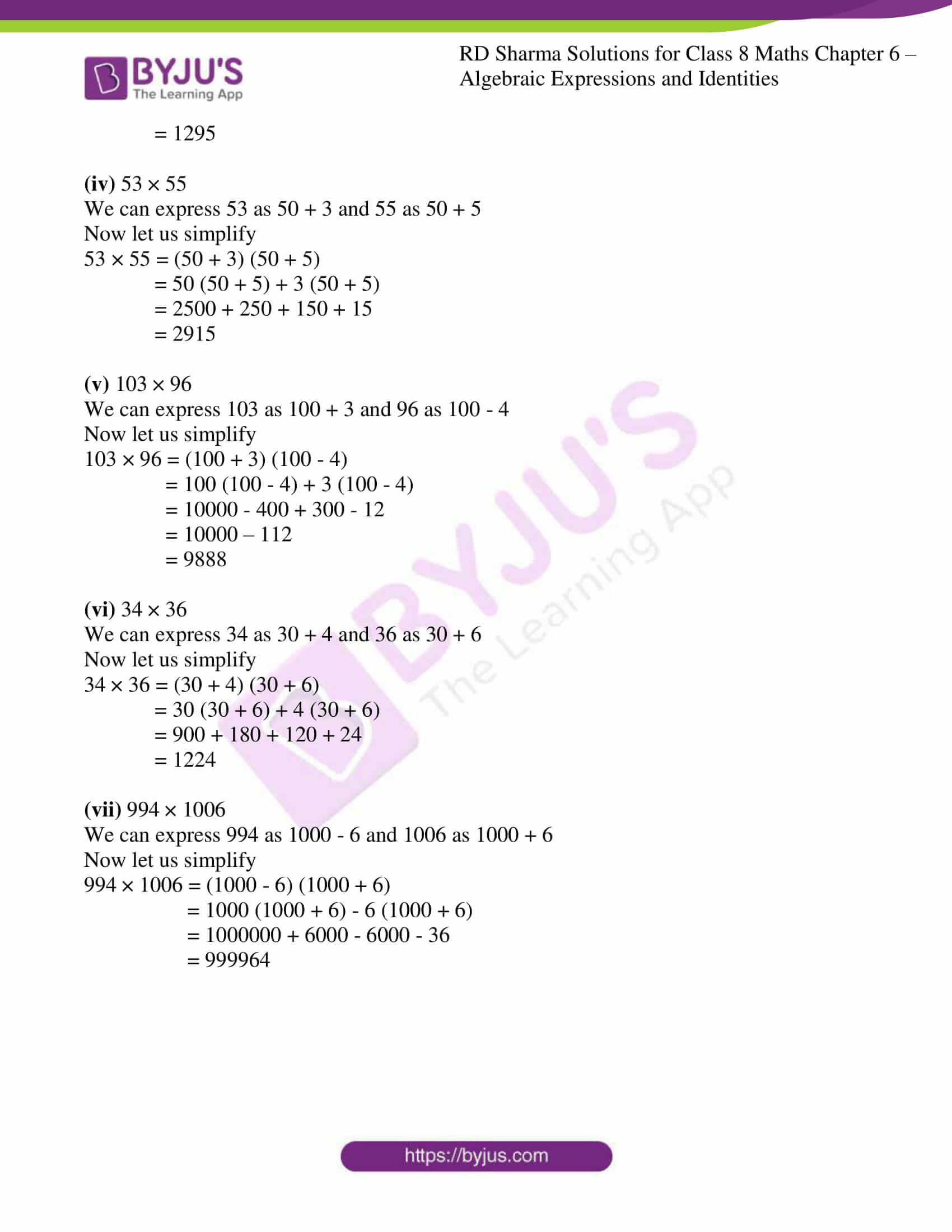### EXERCISE 6.1 PAGE NO: 6.2

1. Identify the terms, their coefficients for each of the following expressions:

(i) 7x2yz – 5xy

(ii) x2 + x + 1

(iii) 3x2y2 – 5x2y2z2 + z2

(iv) 9 – ab + bc – ca

(v) a/2 + b/2 – ab

(vi) 0.2x – 0.3xy + 0.5y

Solution:
(i)
7x2yz – 5xy

The given equation has two terms that are:

7x2yz and – 5xy

The coefficient of 7x2yz is 7

The coefficient of – 5xy is – 5

(ii) x2 + x + 1

The given equation has three terms that are:

x2, x, 1

The coefficient of x2 is 1

The coefficient of x is 1

The coefficient of 1 is 1

(iii) 3x2y2 – 5x2y2z2 + z2

The given equation has three terms that are:

3x2y, -5x2y2z2 and z2

The coefficient of 3x2y is 3

The coefficient of -5x2y2z2 is -5

The coefficient of z2 is 1

(iv) 9 – ab + bc – ca

The given equation has four terms that are:

9, -ab, bc, -ca

The coefficient of 9 is 9

The coefficient of -ab is -1

The coefficient of bc is 1

The coefficient of -ca is -1

(v) a/2 + b/2 – ab

The given equation has three terms that are:

a/2, b/2, -ab

The coefficient of a/2 is 1/2

The coefficient of b/2 is 1/2

The coefficient of -ab is -1

(vi) 0.2x – 0.3xy + 0.5y

The given equation has three terms that are:

0.2x, -0.3xy, 0.5y

The coefficient of 0.2x is 0.2

The coefficient of -0.3xy is -0.3

The coefficient of 0.5y is 0.5

2. Classify the following polynomials as monomials, binomials, trinomials. Which polynomials do not fit in any category?

(i) x+y
(ii) 1000
(iii) x+x2+x3+x4
(iv) 7+a+5b
(v) 2b-3b2
(vi) 2y-3y2+4y3
(vii) 5x-4y+3x
(viii) 4a-15a2
(ix) xy+yz+zt+tx
(x) pqr
(xi) p2q+pq2
(xii) 2p+2q

Solution:

(i) x+y

The given expression contains two terms x and y

∴ It is Binomial

(ii) 1000

The given expression contains one term 1000

∴ It is Monomial

(iii) x+x2+x3+x4

The given expression contains four terms

∴ It belongs to none of the categories

(iv) 7+a+5b

The given expression contains three terms

∴ It is Trinomial

(v) 2b-3b2

The given expression contains two terms

∴ It is Binomial

(vi) 2y-3y2+4y3

The given expression contains three terms

∴ It is Trinomial

(vii) 5x-4y+3x

The given expression contains three terms

∴ It is Trinomial

(viii) 4a-15a2

The given expression contains two terms

∴ It is Binomial

(ix) xy + yz + zt + tx

The given expression contains four terms

∴ It belongs to none of the categories

(x) pqr

The given expression contains one term

∴ It is Monomial

(xi) p2q+pq2

The given expression contains two terms

∴ It is Binomial

(xii) 2p+2q

The given expression contains two terms

∴ It is Binomial

### EXERCISE 6.2 PAGE NO: 6.5

1. Add the following algebraic expressions:

(i) 3a2b, -4a2b, 9a2b

(ii) 2/3a, 3/5a, -6/5a

(iii) 4xy2 – 7x2y, 12x2y -6xy2, -3x2y + 5xy2

(iv) 3/2a – 5/4b + 2/5c, 2/3a – 7/2b + 7/2c, 5/3a + 5/2b – 5/4c

(v) 11/2xy + 12/5y + 13/7x, -11/2y – 12/5x – 137xy

(vi) 7/2x3 – 1/2x2 + 5/3, 3/2x3 + 7/4x2 – x + 1/3, 3/2x2 -5/2x -2

Solution:

(i) 3a2b, -4a2b, 9a2b

Let us add the given expression

3a2b + (-4a2b) + 9a2b

3a2b – 4a2b + 9a2b

8a2b

(ii) 2/3a, 3/5a, -6/5a

Let us add the given expression

2/3a + 3/5a + (-6/5a)

2/3a + 3/5a – 6/5a

Let us take LCM for 3 and 5 which is 15

(2×5)/(3×5)a + (3×3)/(5×3)a – (6×3)/(5×3)a

10/15a + 9/15a – 18/15a

(10a+9a-18a)/15

a/15

(iii) 4xy2 – 7x2y, 12x2y -6xy2, -3x2y + 5xy2

Let us add the given expression

4xy2 – 7x2y + 12x2y – 6xy2 – 3x2y + 5xy2

Upon rearranging

12x2y – 3x2y – 7x2y – 6xy2 + 5xy2 + 4xy2

3xy2 + 2x2y

(iv) 3/2a – 5/4b + 2/5c, 2/3a – 7/2b + 7/2c, 5/3a + 5/2b – 5/4c

Let us add the given expression

3/2a – 5/4b + 2/5c + 2/3a – 7/2b + 7/2c + 5/3a + 5/2b – 5/4c

Upon rearranging

3/2a + 2/3a + 5/3a – 5/4b – 7/2b + 5/2b + 2/5c + 7/2c – 5/4c

By taking LCM for (2 and 3 is 6), (4 and 2 is 4), (5,2 and 4 is 20)

(9a+4a+10a)/6 + (-5b-14b+10b)/4 + (8c+70c-25c)/20

23a/6 – 9b/4 + 53c/20

(v) 11/2xy + 12/5y + 13/7x, -11/2y – 12/5x – 13/7xy

Let us add the given expression

11/2xy + 12/5y + 13/7x + -11/2y – 12/5x – 13/7xy

Upon rearranging

11/2xy – 13/7xy + 13/7x – 12/5x + 12/5y -11/2y

By taking LCM for (2 and 7 is 14), (7 and 5 is 35), (5 and 2 is 10)

(11xy-12xy)/14 + (65x-84x)/35 + (24y-55y)/10

51xy/14 – 19x/35 – 31y/10

(vi) 7/2x3 – 1/2x2 + 5/3, 3/2x3 + 7/4x2 – x + 1/3, 3/2x2 -5/2x – 2

Let us add the given expression

7/2x3 – 1/2x2 + 5/3 + 3/2x3 + 7/4x2 – x + 1/3 + 3/2x2 -5/2x – 2

Upon rearranging

7/2x3 + 3/2x3 – 1/2x2 + 7/4x2 + 3/2x2 – x – 5/2x + 5/3 + 1/3 – 2

10/2x3 + 11/4x2 – 7/2x + 0/6

5x3 + 11/4x2 -7/2x

2. Subtract:
(i) -5xy from 12xy
(ii) 2a2 from -7a2
(iii) 2a-b from 3a-5b
(iv) 2x3 – 4x2 + 3x + 5 from 4x3 + x2 + x + 6
(v) 2/3y3 – 2/7y2 – 5 from 1/3y3 + 5/7y2 + y – 2
(vi) 3/2x – 5/4y – 7/2z from 2/3x + 3/2y – 4/3z
(vii) x2y – 4/5xy2 + 4/3xy from 2/3x2y + 3/2xy2 – 1/3xy
(viii) ab/7 -35/3bc + 6/5ac from 3/5bc – 4/5ac

Solution:

(i) -5xy from 12xy

Let us subtract the given expression

12xy – (- 5xy)

5xy + 12xy

17xy

(ii) 2a2 from -7a2

Let us subtract the given expression

(-7a2) – 2a2

-7a2 – 2a2

-9a2

(iii) 2a-b from 3a-5b

Let us subtract the given expression

(3a – 5b) – (2a – b)

3a – 5b – 2a + b

a – 4b

(iv) 2x3 – 4x2 + 3x + 5 from 4x3 + x2 + x + 6

Let us subtract the given expression

(4x3 + x2 + x + 6) – (2x3 – 4x2 + 3x + 5)

4x3 + x2 + x + 6 – 2x3 + 4x2 – 3x – 5

2x3 + 5x2 – 2x + 1

(v) 2/3y3 – 2/7y2 – 5 from 1/3y3 + 5/7y2 + y – 2

Let us subtract the given expression

1/3y3 + 5/7y2 + y – 2 – 2/3y3 + 2/7y2 + 5

Upon rearranging

1/3y3 – 2/3y3 + 5/7y2 + 2/7y2 + y – 2 + 5

By grouping similar expressions we get,

-1/3y3 + 7/7y2 + y + 3

-1/3y3 + y2 + y + 3

(vi) 3/2x – 5/4y – 7/2z from 2/3x + 3/2y – 4/3z

Let us subtract the given expression

2/3x + 3/2y – 4/3z – (3/2x – 5/4y – 7/2z)

Upon rearranging

2/3x – 3/2x + 3/2y + 5/4y – 4/3z + 7/2z

By grouping similar expressions we get,

LCM for (3 and 2 is 6), (2 and 4 is 4), (3 and 2 is 6)

(4x-9x)/6 + (6y+5y)/4 + (-8z+21z)/6

-5x/6 + 11y/4 + 13z/6

(vii) x2y – 4/5xy2 + 4/3xy from 2/3x2y + 3/2xy2 – 1/3xy

Let us subtract the given expression

2/3x2y + 3/2xy2 – 1/3xy – (x2y – 4/5xy2 + 4/3xy)

Upon rearranging

2/3x2y – x2y + 3/2xy2 + 4/5xy2 – 1/3xy – 4/3xy

By grouping similar expressions we get,

LCM for (3 and 1 is 3), (2 and 5 is 10), (3 and 3 is 3)

-1/3x2y + 23/10xy2 – 5/3xy

(viii) ab/7 -35/3bc + 6/5ac from 3/5bc – 4/5ac

Let us subtract the given expression

3/5bc – 4/5ac – (ab/7 – 35/3bc + 6/5ac)

Upon rearranging

3/5bc + 35/3bc – 4/5ac – 6/5ac – ab/7

By grouping similar expressions we get,

LCM for (5 and 3 is 15), (5 and 5 is 5)

(9bc+175bc)/15 + (-4ac-6ac)/5 – ab/7

184bc/15 + -10ac/5 – ab/7

– ab/7 + 184bc/15 – 2ac

3. Take away:

(i) 6/5x2 – 4/5x3 + 5/6 + 3/2x from x3/3 – 5/2x2 + 3/5x + 1/4

(ii) 5a2/2 + 3a3/2 + a/3 – 6/5 from 1/3a3 – 3/4a2 – 5/2

(iii) 7/4x3 + 3/5x2 + 1/2x + 9/2 from 7/2 – x/3 – x2/5
(iv) y3/3 + 7/3y2 + 1/2y + 1/2 from 1/3 – 5/3y2

(v) 2/3ac – 5/7ab + 2/3bc from 3/2ab -7/4ac – 5/6bc

Solution:

(i) 6/5x2 – 4/5x3 + 5/6 + 3/2x from x3/3 – 5/2x2 + 3/5x + 1/4

Let us subtract the given expression

1/3x3 – 5/2x2 + 3/5x + 1/4 – (6/5x2 – 4/5x3 + 5/6 + 3/2x)

Upon rearranging

1/3x3 + 4/5x3 – 5/2x2 – 6/5x2 + 3/5x – 3/2x + 1/4 – 5/6

By grouping similar expressions we get,

LCM for (3 and 5 is 15), (2 and 5 is 10), (5 and 2 is 10), (4 and 6 is 24)

17/15x3 – 37/10x2 – 9/10x – 14/24

17/15x3 – 37/10x2 – 9/10x – 7/12

(ii) 5a2/2 + 3a3/2 + a/3 – 6/5 from 1/3a3 – 3/4a2 – 5/2

Let us subtract the given expression

1/3a3 – 3/4a2 – 5/2 – (5/2a2 + 3/2a3 + a/3 – 6/5)

Upon rearranging

1/3a5 – 3/2a3 – 3/4a2 – 5/2a2 – a/3 – 5/2 + 6/5

By grouping similar expressions we get,

LCM for (3 and 2 is 6), (4 and 2 is 4), (2 and 5 is 10)

(2a3 – 9a3)/6 – (3a2 + 10a2)/4 – a/3 + (-25+12)/10

-7/6a3 – 13/4a2 – a/3 – 13/10

(iii) 7/4x3 + 3/5x2 + 1/2x + 9/2 from 7/2 – x/3 – x2/5

Let us subtract the given expression

7/2 – x/3 – 1/5x2 – (7/4x3 + 3/5x2 + 1/2x + 9/2)

Upon rearranging

-7/4x3 – 1/5x2 – 3/5x2 – x/3 – x/2 + 7/2 – 9/2

By grouping similar expressions we get,

LCM for (3 and 2 is 6)

-7/4x3 – 4/5x2– (2x-3x)/6 + (7-9)/2

-7/4x3 – 4/5x2 – 5/6x – 1

(iv) y3/3 + 7/3y2 + 1/2y + 1/2 from 1/3 – 5/3y2

Let us subtract the given expression

1/3 – 5/3y2 – (1/3y3 + 7/3y2 + 1/2y + 1/2)

Upon rearranging

-1/3y3 – 5/3y2 – 7/3y2 – 1/2y + 1/3 – 1/2

By grouping similar expressions we get,

LCM for (3 and 3 is 3), (3 and 2 is 6)

-1/3y3 + (-5y2 – 7y2)/3 – 1/2y + (2-3)/6

-1/3y3 – 12/3y2 – 1/2y – 1/6

(v) 2/3ac – 5/7ab + 2/3bc from 3/2ab -7/4ac – 5/6bc

Let us subtract the given expression

3/2ab – 7/4ac – 5/6bc – (2/3ac – 5/7ab + 2/3bc)

Upon rearranging

3/2ab + 5/7ab – 7/4ac – 2/3ac – 5/6bc – 2/3bc

By grouping similar expressions we get,

LCM for (2 and 7 is 14), (4 and 3 is 12), (6 and 3 is 6)

(21ab+10ab)/14 – (21ac-8ac)/12 – (5bc-4bc)/6

31/14ab – 29/12ac – 3/2bc

4. Subtract 3x – 4y – 7z from the sum of x – 3y + 2z and -4x + 9y – 11z.

Solution:

The sum of x – 3y + 2z and -4x + 9y – 11z is

(x – 3y + 2z) + (-4x + 9y – 11z)

Upon rearranging

x – 4x – 3y + 9y + 2z – 11z

-3x + 6y – 9z

Now, Let us subtract the given expression from -3x + 6y – 9z

(-3x + 6y – 9z) – (3x – 4y – 7z)

Upon rearranging

-3x – 3x + 6y + 4y – 9z + 7z

-6x + 10y – 2z

5. Subtract the sum of 3l – 4m – 7n2 and 2l + 3m – 4n2 from the sum of 9l + 2m – 3n2 and -3l + m + 4n2….

Solution:

Sum of 3l – 4m – 7n2 and 2l + 5m – 4n2

3l – 4m – 7n2 + 2l + 3m – 4n2

Upon rearranging

3l + 2l – 4m + 3m – 7n2 – 4n2

5l – m – 11n2 ……………………..equation (1)

Sum of 9l + 2m – 3n2 and -3l + m + 4n2

9l + 2m – 3n2 + (-3l + m + 4n2)

Upon rearranging

9l – 3l + 2m + m – 3n2 + 4n2

6l + 3m + n2 ……………………….equation (2)

Let us subtract equation (i) from (ii), we get

6l + 3m + n2 – (5l – m – 11n2)

Upon rearranging

6l – 5l + 3m + m + n2 + 11n2

l + 4m + 12n2

6. Subtract the sum of 2x – x2 + 5 and -4x – 3 + 7x2 from 5.

Solution:

Sum of 2x – x2 + 5 and -4x – 3 + 7x2 is

2x – x2 + 5 + (-4x – 3 + 7x2)

2x – x2 + 5 – 4x – 3 + 7x2

Upon rearranging

– x2 + 7x2 + 2x – 4x + 5 – 3

6x2 -2x + 2 ………….equation (i)

Let us subtract equation (i) from 5 we get,

5 – (6x2 -2x + 2)

5 – 6x2 + 2x – 2

3 + 2x – 6x2

7. Simplify each of the following:

(i) x2 – 3x + 5 – 1/2(3x2 – 5x + 7)

(ii) [5 – 3x + 2y – (2x – y)] – (3x – 7y + 9)

(iii) 11/2x2y – 9/4xy2 + 1/4xy – 1/14y2x + 1/15yx2 + 1/2xy

(iv) (1/3y2 – 4/7y + 11) – (1/7y – 3 + 2y2) – (2/7y – 2/3y2 + 2)

(v) -1/2a2b2c + 1/3ab2c – 1/4abc2 – 1/5cb2a2 + 1/6cb2a – 1/7c2ab + 1/8ca2b

Solution:

(i) x2 – 3x + 5 – 1/2(3x2 – 5x + 7)

Upon rearranging

x2 – 3/2x2 – 3x + 5/2x + 5 – 7/2

By grouping similar expressions we get,

LCM for (1 and 2 is 2)

(2x2 – 3x2)/2 – (6x + 5x)/2 + (10-7)/2

-1/2x2 – 1/2x + 3/2

(ii) [5 – 3x + 2y – (2x – y)] – (3x – 7y + 9)

5 – 3x + 2y – 2x + y – 3x + 7y – 9

Upon rearranging

– 3x – 2x – 3x + 2y + y + 7y + 5 – 9

By grouping similar expressions we get,

-8x + 10y – 4

(iii) 11/2x2y – 9/4xy2 + 1/4xy – 1/14y2x + 1/15yx2 + 1/2xy

Upon rearranging

11/2x2y + 1/15x2y – 9/4xy2 – 1/14xy2 + 1/4xy + 1/2xy

By grouping similar expressions we get,

LCM for (2 and 15 is 30), (4 and 14 is 56), (4 and 2 is 4)

(165x2y + 2x2y)/30 + (-126xy2 – 4xy2)/56 + (xy + 2xy)/4

167/30x2y – 130/56xy2 + 3/4xy

167/30x2y – 65/28xy2 + 3/4xy

(iv) (1/3y2 – 4/7y + 11) – (1/7y – 3 + 2y2) – (2/7y – 2/3y2 + 2)

Upon rearranging

1/3y2 – 2y2 – 2/3y2 – 4/7y – 1/7y – 2/7y + 11 + 3 – 2

By grouping similar expressions we get,

LCM for (3, 1 and 3 is 3), (7, 7 and 7 is 7)

(y2 – 6y2 + 2y2)/3 – (4y – y – 2y)/7 + 12

-3/3y2 – 7/7y + 12

-y2 – y + 12

(v) -1/2a2b2c + 1/3ab2c – 1/4abc2 – 1/5cb2a2 + 1/6cb2a – 1/7c2ab + 1/8ca2b

Upon rearranging

-1/2a2b2c – 1/5a2b2c + 1/3ab2c + 1/6ab2c – 1/4abc2 – 1/7abc2 + 1/8a2bc

By grouping similar expressions we get,

LCM for (2 and 5 is 10), (3 and 6 is 6), (4 and 7 is 28)

-7/10a2b2c + 1/2ab2c – 11/28abc2 + 1/8a2bc

### EXERCISE 6.3 PAGE NO: 6.13

Find each of the following products:

1. 5x2 × 4x3

Solution:

Let us simplify the given expression

5 × x × x × 4 × x × x × x

5 × 4 × x1+1+1+1+1

20 × x5

20x5

2. -3a2 × 4b4

Solution:

Let us simplify the given expression

– 3 × a2 × 4 × b4

-12 × a2 × b4

-12a2b4

3. (-5xy) × (-3x2yz)

Solution:

Let us simplify the given expression

(-5) × (-3) × x × x2 × y × y × z

15 × x1+2 × y1+1 × z

15x3y2z

4. 1/2xy × 2/3x2yz2

Solution:

Let us simplify the given expression

1/2 × 2/3 × x × x2 × y × y × z2

1/3 × x1+2 × y1+1 × z2

1/3x3y2z2

5. (-7/5xy2z) × (13/3x2yz2)

Solution:

Let us simplify the given expression

-7/5 × 13/3 × x × x2 × y2 × y × z × z2

-91/15 × x1+2 × y2+1 × z1+2

-91/15x3y3z3

6. (-24/25x3z) × (-15/16xz2y)

Solution:

Let us simplify the given expression

-24/25 × -15/16 × x3 × x × z × z2 × y

18/20 × x3+1 × z1+2 × y

9/10x4z3y

7. (-1/27a2b2) × (9/2a3b2c2)

Solution:

Let us simplify the given expression

-1/27 × 9/2 × a2 × a3 × b2 × b2 × c2

-1/6 x a2+3 × b2+2 × c2

-1/6a5b4c2

8. (-7xy) × (1/4x2yz)

Solution:

Let us simplify the given expression

-7 × 1/4 × x × y × x2 × y × z

-7/4 × x1+2 × y1+1 × z

-7/4x3y2z

9. (7ab) × (-5ab2c) × (6abc2)

Solution:

Let us simplify the given expression

7 × -5 × 6 × a × a × a × b × b2 × b × c × c2

210 × a1+1+1 × b1+2+1 × c1+2

210a3b4c3

10. (-5a) × (-10a2) × (-2a3)

Solution:

Let us simplify the given expression

(-5) × (-10) × (-2) × a × a2 × a3

-100 × a1+2+3

-100a6

11. (-4x2) × (-6xy2) × (-3yz2)

Solution:

Let us simplify the given expression

(-4) × (-6) – (-3) × x2 × x × y2 × y × z2

– 72 × x2+1 × y2+1 × z2

-72x3y3z2

12. (-2/7a4) × (-3/4a2b) × (-14/5b2)

Solution:

Let us simplify the given expression

-2/7 × -3/4 × -14/5 × a4 × a2 × b × b2

-6/10 × a4+2 × b1+2

-3/5a6b3

13. (7/9ab2) × (15/7ac2b) × (-3/5a2c)

Solution:

Let us simplify the given expression

7/9 × 15/7 × -3/5 × a × a × a2 × b2 × b × c2 × c

– a1+1+2 × b2+1 × c2+1

-a4b3c3

14. (4/3u2vw) × (-5uvw2) × (1/3v2wu)

Solution:

Let us simplify the given expression

4/3 × -5 × 1/3 × u2 × u × u × v × v × v2 × w × w2 × w

-20/9 × u2+1+1 × v1+1+2 × w1+2+1

-20/9u4v4w4

15. (0.5x) × (1/3xy2z4) × (24x2yz)

Solution:

Let us simplify the given expression

0.5 × 1/3 × 24 × x × x × y2 × y × x2 × z4 × z

12/3 × x1+1+2 × y2+1 × z4+1

4x4 × y3 × z5

4x4y3z5

16. (4/3pq2) × (-1/4p2r) × (16p2q2r2)

Solution:

Let us simplify the given expression

4/3 × 1/4 × 16 × p × p2 × p2 × q2 × q2 × r × r2

-16/3 × p1+2+2 × q2+2 × r1+2

-16/3p5q4r3

17. (2.3xy) × (0.1x) × (0.16)

Solution:

Let us simplify the given expression

2.3 × 0.1 × 0.16 × x × x × y

0.0368 × x1+1 × y

0.0368x2y

Express each of the following products as a monomials and verify the result in each case for x=1:

18. (3x) × (4x) × (-5x)

Solution:

Let us simplify the given expression

3 × 4 × -5 × x × x × x

-60 × x1+1+1

-60x3

Verification

LHS = (3 × 1) × (4 × 1) × (-5 × 1)

= 3 × 4 × – 5

= – 60

RHS = -60 (1)3 = – 60

Therefore, LHS = RHS.

19. (4x2) × (-3x) × (4/5x3)

Solution:

Let us simplify the given expression

4 × -3 × 4/5 × x2 × x × x3

-48/5 × x2+1+3

-48/5x6

Verification

LHS = 4 × 12 × – 3 × 1 × 4/5 × 13

= – 48/5

RHS = – 48/5 × 16 = – 48/5

Therefore, LHS = RHS.

20. (5x4) × (x2)3 × (2x) 2

Solution:

Let us simplify the given expression

5 × x4 × x6 × 4 × x2

5 × 4 × x4 × x6 × x2

20 × x4+6+2

20x12

Verification

LHS = (5 × 14) × (12)3 × (2 × 1)2

= 5 × 4

= 20

RHS = 20 × 112 = 20

Therefore, LHS = RHS.

21. (x2)3 × (2x) × (-4x) × (5)

Solution:

Let us simplify the given expression

x6 × 2 × x × -4 × x × 5

2 × -4 × 5 × x6 × x × x

-40 × x6+1+1

-40x8

Verification

LHS = (12)3 × (2 × 1) × (-4 × 1) × 5

= – 40

RHS = – 40 × 18 = – 40

Therefore, LHS = RHS.

22. Write down the product of -8x2y6 and -20xy verify the product for x = 2.5, y = 1

Solution:

Let us simplify the given expression

-8 × -20 × x2 × x × y6 × y

160 × x2+1 × y6+1

160x3y7

Now let us verify when, x = 2.5 and y = 1

For 160x3y7

160 (2.5)3 × (1)7

160 × 15.625

2500

For -8x2y6 and -20xy

-8 × 2.52 × 16 × -20 × 1 × 2.5

2500

Hence, the given expression is verified.

23. Evaluate (3.2x6y3× (2.1x2y2) when x = 1 and y = 0.5

Solution:

Let us simplify the given expression

3.2 × 2.1 × x6 × x2 × y3 × y2

6.72 × x6+2 × y3+2

6.72x8y5

Now let us substitute when, x = 1 and y = 0.5

For 6.72x8y5

6.72 × 18 × 0.55

0.21

24. Find the value of (5x6) × (-1.5x2y3) × (-12xy2) when x = 1, y = 0.5

Solution:

Let us simplify the given expression

5 × -1.5 × -12 × x6 × x2 × x × y3 × y2

90 × x6+2+1 × y3+2

90x9y5

Now let us substitute when, x = 1 and y = 0.5

For 90x9y5

90 × (1)9× (0.5)5

2.8125

45/16

25. Evaluate (2.3a5b2) × (1.2a2b2) when a = 1 and b = 0.5

Solution:

Let us simplify the given expression

2.3a5b2 × 1.2a2b2

2.3 × 1.2 × a5 × a2 × b2 × b2

2.76 × a5+2 × b2+2

2.76a7b4

Now let us substitute when, a = 1 and b = 0.5

For 2.76 a7 b4

2.76 (1)7 (0.5)4

2.76 × 1 × 0.0025

0.1725

6.9/40

26. Evaluate (-8x2y6) × (-20xy) for x = 2.5 and y = 1

Solution:

Let us simplify the given expression

-8 × – 20 × x2 × x × y6 × y

160x2+1y6+1

160x3y7

Now let us substitute when, x = 2.5 and y = 1

160x3y7

160 × (2.5)3 × (1)7

2500

Express each of the following products as a monomials and verify the result for x = 1, y = 2:

27. (-xy3) × (yx3) × (xy)

Solution:

Let us simplify the given expression

-x × y3 × y × x3 × x × y

-x1+3+1 × y3+1+1

-x5y5

Now let us substitute when, x = 1 and y = 2

-x5y5

-15 × 25

-32

28. (1/8x2y4) × (1/4x4y2) × (xy) × 5

Solution:

Let us simplify the given expression

1/8 × 1/4 × 5 × x2 × x4 × x × y4 × y2 × y

5/32 × x2+4+1 × y4+2+1

5/32x7y7

Now let us substitute when, x = 1 and y = 2

5/32 × 17 × 27

5/32 × 128

5 × 4

20

29. (2/5a2b) × (-15b2ac) × (-1/2c2)

Solution:

Let us simplify the given expression

2/5 × -15 × -1/2 × a2 × a × b × b2 × c × c2

3 × a2+1 × b1+2 × c1+2

3a3b3c3

30. (-4/7a2b) × (-2/3b2c) × (-7/6c2a)

Solution:

Let us simplify the given expression

-4/7 × -2/3 × -7/6 × a2 × a × b × b2 × c × c2

-4/9 × a2+1 × b2+1 × c1+2

-4/9a3b3c3

31. (4/9abc3) × (-27/5a3b2) × (-8b3c)

Solution:

Let us simplify the given expression

4/9 × -27/5 × -8 × a × a3 × b × b2 × b3 × c3 × c

96/5 × a1+3 × b1+2+3 × c3+1

96/5a4b6c4

Evaluate each of the following when x = 2, y = -1.

32. (2xy) × (x2y/4) × (x2) × (y2)

Solution:

Let us simplify the given expression

2 × 1/4 × x × x2 × x2 × y × y2 × y

1/2x1+2+2y1+2+1

1/2x5y4

Now let us substitute when, x = 2 and y = -1

For 1/2x5y4

1/2 × (2)5 × (-1)4

1/2 × 32 × 1

16

33. (3/5x2y) × (-15/4xy2) × (7/9x2y2)

Solution:

Let us simplify the given expression

3/5 × -15/4 × 7/9 × x2 × x × x2 × y × y2 × y2

-7/4 × x2+1+2 × y1+2+2

7/4x5y5

Now let us substitute when, x = 2 and y = -1

For -7/4x5y5

-7/4 × (2)5 (-1)5

-7/4 × 32 × -1

56

### EXERCISE 6.4 PAGE NO: 6.21

Find the following products:

1. 2a3 (3a + 5b)

Solution:

Let us simplify the given expression

2a3 (3a + 5b)

(2a3 × 3a) + (2a3 × 5b)

6a3+1 + 10a3b

6a4 + 10a3b

2. -11a (3a + 2b)

Solution:

Let us simplify the given expression

-11a (3a + 2b)

(-11a × 3a) + (-11a × 2b)

-33a2 – 22ab

3. -5a (7a – 2b)

Solution:

Let us simplify the given expression

-5a (7a – 2b)

(-5a × 7a) – (-5a × 2b)

-35a2 + 10ab

4. -11y2 (3y + 7)

Solution:

Let us simplify the given expression

-11y2 (3y + 7)

(-11y2 × 3y) + (-11y2 × 7)

-33y3 – 77y2

5. 6x/5(x3 + y3)

Solution:

Let us simplify the given expression

6/5x (x3 + y3)

(6/5x × x3) + (6/5x × y3)

6/5x4 + 6/5xy3

6. xy (x3 – y3)

Solution:

Let us simplify the given expression

xy (x3 – y3)

(xy × x3) – (xy × y3)

x4y – xy4

7. 0.1y (0.1x5 + 0.1y)

Solution:

Let us simplify the given expression

0.1y (0.1x5 + 0.1y)

(0.1y × 0.1x5) + (0.1y × 0.1y)

0.01x5y + 0.01y2

8. (-7/4ab2c – 6/25a2c2) (-50a2b2c2)

Solution:

Let us simplify the given expression

(-7/4ab2c – 6/25a2c2) (-50a2b2c2)

(-7/4ab2c × -50a2b2c2) – (6/25a2c2 × -50a2b2 × c2)

350/4a3b4c3 + 12a4b2c4

175/2a3b4c3 + 12a4b2c4

9. -8/27xyz (3/2xyz2 – 9/4xy2z3)

Solution:

Let us simplify the given expression

-8/27xyz (3/2xyz2 – 9/4xy2z3)

(-8/27xyz × 3/2xyz2) – (-8/27xyz × 9/4xy2z3)

-4/9x2y2z3 + 2/3x2y3z4

10. -4/27xyz (9/2x2yz – 3/4xyz2)

Solution:

Let us simplify the given expression

-4/27xyz (9/2x2yz – 3/4xyz2)

(-4/27xyz × 9/2x2yz) – (-4/27xyz × 3/4xyz2)

-2/3x3y2z2 + 1/9x2y2z3

11. 1.5x (10x2y – 100xy2)

Solution:

Let us simplify the given expression

1.5x (10x2y – 100xy2)

(1.5x × 10x2y) – (1.5x × 100xy2)

15x3y – 150x2y2

12. 4.1xy (1.1x – y)

Solution:

Let us simplify the given expression

4.1xy (1.1x – y)

(4.1xy × 1.1x) – (4.1xy × y)

4.51x2y – 4.1xy2

13. 250.5xy (xz + y/10)

Solution:

Let us simplify the given expression

250.5xy (xz + y/10)

(250.5xy × xz) + (250.5xy × y/10)

250.5x2yz + 25.05xy2

14. 7/5x2y (3/5xy2 + 2/5x)

Solution:

Let us simplify the given expression

7/5x2y (3/5xy2 + 2/5x)

(7/5x2y × 3/5xy2) + (7/5x2y × 2/5x)

21/25x3y3 + 14/25x3y

15. 4/3a (a2 + b2 – 3c2)

Solution:

Let us simplify the given expression

4/3a (a2 + b2 – 3c2)

(4/3a × a2) + (4/3a × b2) – (4/3a × 3c2)

4/3a3 + 4/3ab2 – 4ac2

16. Find the product 24x2 (1-2x) and evaluate its value for x = 3

Solution:

Let us simplify the given expression

24x2 (1 – 2x)

(24x2× 1) – (24x2× 2x)

24x2 – 48x3

Now let us evaluate the expression when x = 3

24x2 – 48x3

24 × (3)2 – 48 × (3)3

24 × (9) – 48 × (27)

216 – 1296

-1080

17. Find the product -3y (xy+y2) and evaluate its value for x = 4 and y = 5

Solution:

Let us simplify the given expression

-3y (xy+y2)

(-3y × xy) + (-3y × y2)

-3xy2 – 3y3

Now let us evaluate the expression when x = 4 and y = 5

-3xy2 – 3y3

-3 × (4) × (5)2 – 3 × (5)3

-300 – 375

-675

18. Multiply -3/2x2y3 by (2x-y) and verify the answer for x = 1 and y = 2

Solution:

Let us simplify the given expression

-3/2x2y3 by (2x-y)

(-3/2x2y3 × 2x) – (-3/2x2y3 × y)

-3x3y3 + 3/2x2y4

Now let us evaluate the expression when x = 1 and y = 2

-3x3y3 + 3/2x2y4

-3 × (1)4 × (2)3 + 3/2 × (1)2 × (2)4

– 3 × (8) + 3 (8)

-24+24

0

19. Multiply the monomial by the binomial and find the value of each for x = -1, y = 0.25 and z = 0.005:
(i) 15y2 (2 – 3x)
(ii) -3x (y2 + z2)
(iii) z2 (x – y)
(iv) xz (x2 + y2)

Solution:

(i) 15y2 (2 – 3x)

Let us simplify the given expression

30y2 – 45xy2

By evaluating the values in the expression x = -1, y = 25/100 and z = 5/1000

30 × (25/100)2 – 45 × (-1) × (25/100)2

30 (1/16) + 45 (1/16)

15/8 + 45/16

(30+45)/16

75/16

(ii) -3x (y2 + z2)

Let us simplify the given expression

-3xy2 + -3xz2

By evaluating the values in the expression x = -1, y = 25/100 and z = 5/1000

-3× (-1) × (25/100)2 – 3 × (-1) × (5/1000)2

(3×25×25/100×100) + (3×5×5/1000×1000)

3/16 + 3/40000

39/200

(iii) z2 (x – y)

Let us simplify the given expression

z2x – z2y

By evaluating the values in the expression x = -1, y = 25/100 and z = 5/1000

z2 (x – y)

(5/1000)2 (-1 – 25/100)

(1/40000) (-100-25/100)

(1/40000) (-125/100)

(1/40000) (-5/4)

-5/160000

-1/32000

(iv) xz (x2 + y2)

Let us simplify the given expression

x3z + xzy2

By evaluating the values in the expression x = -1, y = 25/100 and z = 5/1000

x3z + xzy2

(-1)3 × (5/1000) + (-1) × (5/1000) × (25/100)2

-1/200 – 1/16 × 1/200

-1/200 – 1/3200

By taking LCM as 3200

(-16 -1)/3200

-17/3200

20. Simplify:

(i) 2x2 (x3 – x) – 3x (x4 + 2x) – 2 (x4 – 3x2)

(ii) x3y (x2 – 2x) + 2xy (x3 – x4)

(iii) 3a2 + 2 (a+2) – 3a (2a+1)

(iv) x (x+4) + 3x (2x2 -1) + 4x2 + 4

(v) a (b-c) – b (c-a) – c (a-b)

(vi) a (b-c) +b (c-a) + c (a-b)

(vii) 4ab (a-b) – 6a2 (b-b2) -3b2 (2a2 -a) + 2ab (b-a)

(viii) x2 (x2 + 1) – x3 (x + 1) – x (x3 – x)

(ix) 2a2 + 3a (1 – 2a3) + a (a + 1)

(x) a2 (2a – 1) + 3a + a3 – 8

(xi) 3/2x2 (x2 – 1) + 1/4x2 (x2 + x) – 3/4x (x3 – 1)

(xii) a2b (a-b2) + ab2(4ab – 2a2) – a3b(1-2b)

(xiii) a2b (a3– a + 1) – ab(a4 – 2a2 + 2a) – b(a3– a2 – 1)

Solution:

(i) 2x2 (x3 – x) – 3x (x4 + 2x) – 2 (x4 – 3x2)

Let us simplify the given expression

2x5 – 2x3 – 3x5 – 6x2 – 2x4 + 6x2

By grouping similar expressions we get,

2x5 – 3x5 – 2x3 – 2x4 – 6x2 + 6x2

-x5 – 2x4 – 2x3

(ii) x3y (x2 – 2x) + 2xy (x3 – x4)

Let us simplify the given expression

x5y – 2x4y + 2x4y – 2x5y

By grouping similar expressions we get,

-x5y – 2x5y

-x5y

(iii) 3a2 + 2 (a+2) – 3a (2a+1)

Let us simplify the given expression

3a2 + 2a + 4 – 6a2 – 3a

By grouping similar expressions we get,

3a2 – 6a2 + 2a – 3a + 4

-3a2 – a + 4

(iv) x (x+4) + 3x (2x2 -1) + 4x2 + 4

Let us simplify the given expression

x2 + 4x + 6x3 – 3x + 4x2 + 4

By grouping similar expressions we get,

6x3 + 5x2 + x + 4

(v) a (b-c) – b (c-a) – c (a-b)

Let us simplify the given expression

ab – ac – bc + ab – ca + bc

By grouping similar expressions we get,

2ab – 2ac

(vi) a (b-c) +b (c-a) + c (a-b)

Let us simplify the given expression

ab – ac + bc – ab + ac – bc

By grouping similar expressions we get,

0

(vii) 4ab (a-b) – 6a2 (b-b2) -3b2 (2a2 -a) + 2ab (b-a)

Let us simplify the given expression

4a2b – 4ab2 – 6a2b + 6a2b2 – 6a2b2 + 3ab2 + 2ab2 – 2a2b

By grouping similar expressions we get,

4a2b – 6a2b– 2a2b – 4ab2 + 3ab2 + 2ab2 + 6a2b2 – 6a2b2

-4a2b + ab2

(viii) x2 (x2 + 1) – x3 (x + 1) – x (x3 – x)

Let us simplify the given expression

x4 + x2 – x4 – x3 – x4 + x2

By grouping similar expressions we get,

x4 – x4 – x4 – x3 + x2 + x2

– x4 – x3 + 2x2

(ix) 2a2 + 3a (1 – 2a3) + a (a + 1)

Let us simplify the given expression

2a2 + 3a – 6a4 + a2 + a

By grouping similar expressions we get,

-6a4 + 3a2 + 4a

(x) a2 (2a – 1) + 3a + a3 – 8

Let us simplify the given expression

2a3 – a2 + 3a + a3 – 8

By grouping similar expressions we get,

3a3 – a2 + 3a – 8

(xi) 3/2x2 (x2 – 1) + 1/4x2 (x2 + x) – 3/4x (x3 – 1)

Let us simplify the given expression

3/2x4 – 3/2x2 + 1/4x4 + 1/4x3 – 3/4x4 + 3/4x

By grouping similar expressions we get,

3/2x4 + 1/4x4 – 3/4x4 – 3/2x2 + 1/4x3 + 3/4x

4/4x4 + 1/4x3 – 3/2x2 + 3/4x

x4 + 1/4x3 – 3/2x2 + 3/4x

(xii) a2b (a-b2) + ab2(4ab – 2a2) – a3b(1-2b)

Let us simplify the given expression

a3b – a2b3 + 4a2b3 – 2a3b2 – a3b + 2a3b2

By grouping similar expressions we get,

-a2b3 + 4a2b3

3a2b3

(xiii) a2b (a3– a + 1) – ab(a4 – 2a2 + 2a) – b(a3– a2 – 1)

Let us simplify the given expression

a5b – a3b + a2b – a5b + 2a3b – 2a2b – ba3 + a2b + b

By grouping similar expressions we get,

a5b – a5b – a3b + 2a3b – ba3 + a2b – 2a2b + a2b + b

b

### EXERCISE 6.5 PAGE NO: 6.30

Multiply:

1. (5x + 3) by (7x + 2)

Solution:

Now let us simplify the given expression

(5x + 3) × (7x + 2)

5x (7x + 2) + 3 (7x + 2)

35x2 + 10x + 21x + 6

35x2 + 31x + 6

2. (2x + 8) by (x – 3)

Solution:

Now let us simplify the given expression

(2x + 8) × (x – 3)

2x (x – 3) + 8 (x – 3)

2x2 – 6x + 8x – 24

2x2 + 2x – 24

3. (7x + y) by (x + 5y)

Solution:

Now let us simplify the given expression

(7x + y) × (x + 5y)

7x (x + 5y) + y (x + 5y)

7x2 + 35xy + xy + 5y2

7x2 + 36xy + 5y2

4. (a – 1) by (0.1a2 + 3)

Solution:

Now let us simplify the given expression

(a – 1) × (0.1a2 + 3)

a (0.1a2 + 3) -1 (0.1a2 + 3)

0.1a3 + 3a – 0.1a2 – 3

0.1a3 – 0.1a2 + 3a – 3

5. (3x2 + y2) by (2x2 + 3y2)

Solution:

Now let us simplify the given expression

(3x2 + y2) × (2x2 + 3 y2)

3x2 × (2x2 + 3y2) + y2 × (2x2 + 3y2)

6x4 + 9x2y2 + 2x2y2 + 3y4

6x4 + 11x2y2 + 3y4

6. (3/5x + 1/2y) by (5/6x + 4y)

Solution:

Now let us simplify the given expression

(3/5x + 1/2y) × (5/6x + 4y)

3/5x × (5/6x + 4y) + 1/2y × (5/6x + 4y)

15/30x2 + 12/5xy + 5/12xy + 4/2y2

1/2x2 + 169/60xy + 2y2

7. (x6 – y6) by (x2 + y2)

Solution:

Now let us simplify the given expression

(x6 – y6) × (x2 + y2)

x6 × (x2 + y2) – y6 × (x2 + y2)

x8 + x6y2 – x2y6 – y8

8. (x2 + y2) by (3a + 2b)

Solution:

Now let us simplify the given expression

(x2 + y2) × (3a + 2b)

x2 × (3a + 2b) + y2 × (3a + 2b)

3ax2 + 3ay2 + 2bx2 + 2by2

9. (- 3d – 7f) by (5d + f)

Solution:

Now let us simplify the given expression

(- 3d – 7f) × (5d + f)

-3d (5d + f) – 7f (5d + f)

– 15d2 – 3df – 35df – 7f2

– 15d2 – 38df – 7f2

10. (0.8a – o.5b) by (1.5a – 3b)

Solution:

Now let us simplify the given expression

(0.8a – 0.5b) × (1.5a – 3b)

0.8a (1.5a – 3b) – 0.5b (1.5a – 3b)

1.2a2 – 2.4ab – 0.75ab + 1.5b2

1.2a2 – 3.15ab + 1.5b2

11. (2x2y2 – 5xy2) by (x2 – y2)

Solution:

Now let us simplify the given expression

(2x2y2 – 5xy2) × (x2 – y2)

2x2y2 (x2 – y2) – 5xy2 (x2 – y2)

2x4y2 – 5x3y2 – 2x2y4 + 5xy4

12. (x/7 + x2/2) by (2/5 + 9x/4)

Solution:

Now let us simplify the given expression

(x/7 + x2/2) × (2/5 + 9x/4)

x/7 (2/5 + 9x/4) + x2/2 (2/5 + 9x/4)

2x/35 + (9 x2)/28 + x2/5 + (9 x3)/8

9/8x3 + 73/140x2 + 2/35x

13. (-a/7 + a2/9) by (b/2 – b2/3)

Solution:

Now let us simplify the given expression

(-a/7 + a2/9) × (b/2 – b2/3)

-a/7 (b/2 – b2/3) + a2/9 (b/2 – b2/3)

-ab/14 + ab2/21 + a2b/18 – a2b2/27

14. (3x2y – 5xy2) by (1/5x2 + 1/3y2)

Solution:

Now let us simplify the given expression

(3x2y – 5xy2) × (1/5x2 + 1/3y2)

3x2y (1/5x2 + 1/3y2) – 5xy2 (1/5x2 + 1/3y2)

3/5x4y + 3/3x2y3 – x3y2 + 5/3xy4

3/5x4y + x2y3 – x3y2 + 5/3xy4

15. (2x2 – 1) by (4x3 + 5x2)

Solution:

Now let us simplify the given expression

(2x2 – 1) × (4x3 + 5x2)

2x2 (4x3 + 5x2) – 1 (4x3 + 5x2)

8x5 + 10x4 – 4x3 – 5x2

16. (2xy + 3y2) by (3y2 – 2)

Solution:

Now let us simplify the given expression

(2xy + 3y2) × (3y2 – 2)

2xy (3y2 – 2) + 3y2 (3y2 – 2)

6xy3 – 4xy + 9y4 – 6y2

Find the following products and verify the results for x = -1, y = -2:

17. (3x – 5y) (x + y)

Solution:

Now let us simplify the given expression

(3x – 5y) × (x + y)

(3x – 5y) × (x + y)

x (3x – 5y) + y (3x – 5y)

3x2 – 5xy + 3xy – 5y2

3x2 – 2xy – 5y2

Let us substitute the given values x = – 1 and y = – 2, then

(3x – 5y) × (x + y)

[3 (-1) – 5 (-2)] × [(-1) + (-2)]

(-3+10) × (-1-2)

7×-3

-21

3x2 – 2xy – 5y2

3 (-1)2 – 2 (-1) (-2) – 5 (-2)2

3 – 4 – 20

– 21

∴ the given expression is verified.

18. (x2y – 1) (3 – 2x2y)

Solution:

Now let us simplify the given expression

(x2y – 1) × (3 – 2x2y)

x2y (3 – 2x2y) – 1 (3 – 2x2y)

3x2y – 2x4y2 – 3 + 2x2y

5x2y – 2x4y2 – 3

Let us substitute the given values x = – 1 and y = – 2, then

(x2y – 1) × (3 – 2x2y)

[(-1)2 (-2) – 1] × [3 – 2 (-1)2 (-2)

(-2 – 1) × (3 + 4)

-3 × 7

-21

5x2y – 2x4y2 – 3

[-2 (-1)4 (-2)2 + 5 (-1)2 (2) – 3]

– 8 – 10 – 3

-21

∴ the given expression is verified.

19. (1/3x – y2/5) (1/3x + y2/5)

Solution:

Now let us simplify the given expression

(1/3x – y2/5) × (1/3x + y2/5)

(1/3x) 2 – (y2/5)2

(1/3x – y2/5) (1/3x + y2/5)

1/9x2 – 1/25y4

Let us substitute the given values x = – 1 and y = – 2, then

(1/3x – y2/5) × (1/3x + y2/5)

(1/3(-1) – (-2)2/5) × (1/3(-1) + (-2)2/5)

(-17/15) × (7/15)

-119/225

1/9x2 – 1/25y4

1/9 (-1)2 – 1/25 (-2)4

1/9 -16/25

-119/225

∴ the given expression is verified.

Simplify:

20. x2 (x + 2y) (x – 3y)

Solution:

Now let us simplify the given expression

x2 (x + 2y) (x – 3y)

x2 (x2 – 3xy + 2xy – 3y2)

x2 (x2 – xy – 6y2)

x4 – x3y – 6x2y2

21. (x2 – 2y2) (x + 4y)x2y2

Solution:

Now let us simplify the given expression

(x2 – 2y2) (x + 4y)x2y2

(x3 + 4x2y – 2xy2 – 8y3) × x2y2

x5y2 + 4x4y3 – 2x3y4 – 8x2y5

22. a2b2 (a + 2b) (3a + b)

Solution:

Now let us simplify the given expression

a2b2 (a + 2b) (3a + b)

a2b2 (3a2 + ab + 6ab + 2b2)

a2b2 (3a2 + 7ab + 2b2)

3a4b2 + 7a3b3 + 2a2b4

23. x2 (x – y) y2 (x + 2y)

Solution:

Now let us simplify the given expression

x2 (x – y) y2 (x + 2y)

x2y2 (x2 + 2xy – xy – 2y2)

x2y2 (x2 + xy – 2y2)

x4y2 + x3y3 – 2x2y4

24. (x3 – 2x2 + 5x – 7) (2x – 3)

Solution:

Now let us simplify the given expression

(x3 – 2x2 + 5x – 7) (2x – 3)

2x4 – 4x3 + 10x2 – 14x – 3x3 + 6x2 – 15x + 21

2x4 – 7x3 + 16x2 – 29x + 21

25. (5x + 3) (x – 1) (3x – 2)

Solution:

Now let us simplify the given expression

(5x + 3) (x – 1) (3x – 2)

(5x2 – 2x – 3) (3x – 2)

15x3 – 6x2 – 9x – 10x2 + 4x + 6

15x3 – 16x2 – 5x + 6

26. (5 – x) (6 – 5x) (2 – x)

Solution:

Now let us simplify the given expression

(5 – x) (6 – 5x) (2 – x)

(x2 – 7x + 10) (6 – 5x)

-5x3 + 35x2 – 50x + 6x2 – 42x + 60

60 – 92x + 41x2 – 5x3

27. (2x2 + 3x – 5) (3x2 – 5x + 4)

Solution:

Now let us simplify the given expression

(2x2 + 3x – 5) (3x2 – 5x + 4)

6x4 + 9x3 – 15x2 – 10x3 – 15x2 + 25x + 8x2 + 12x – 20

6x4 – x3 – 22x2 + 37x – 20

28. (3x – 2) (2x – 3) + (5x – 3) (x + 1)

Solution:

Now let us simplify the given expression

(3x – 2) (2x – 3) + (5x – 3) (x + 1)

6x2 – 9x – 4x + 6 + 5x2 + 5x – 3x – 3

11x2 – 11x + 3

29. (5x – 3) (x + 2) – (2x + 5) (4x – 3)

Solution:

Now let us simplify the given expression

(5x – 3) (x + 2) – (2x + 5) (4x – 3)

5x2 + 10x – 3x – 6 – 8x2 + 6x – 20x + 15

-3x2 – 7x + 9

30. (3x + 2y) (4x + 3y) – (2x – y) (7x – 3y)

Solution:

Now let us simplify the given expression

(3x + 2y) (4x + 3y) – (2x – y) (7x – 3y)

12x2 + 9xy + 8xy

12x2 + 9xy + 8xy + 6y2 – 14x2 + 6xy + 7xy – 3y2

-2x2 + 3y2 + 30xy

31. (x2 – 3x + 2) (5x – 2) – (3x2 + 4x – 5) (2x – 1)

Solution:

Now let us simplify the given expression

(x2 – 3x + 2) (5x – 2) – (3x2 + 4x – 5) (2x – 1)

5x3 – 15x2 + 10x – 2x2 + 6x – 4 – (6x3 + 8x2 – 10x – 3x2 – 4x + 5)

5x3 – 6x3 – 15x2 – 2x2 – 5x2 + 16x + 14x – 4 – 5

– x3 – 22x2 + 30x – 9

32. (x3 – 2x2 + 3x – 4) (x – 1) – (2x – 3) (x2 – x + 1)

Solution:

Now let us simplify the given expression

(x3 – 2x2 + 3x – 4) (x – 1) – (2x – 3) (x2 – x + 1)

x4 – 2x3 + 3x2 – 4x – x3 + 2x2 – 3x + 4 – (2x3 – 2x2 + 2x – 3x2 + 3x – 3)

x4 – 3x3 + 5x2 – 7x + 4 – 2x3 + 5x2 – 5x + 3

x4 – 5x3 + 10x2 – 12x + 7

### EXERCISE 6.6 PAGE NO: 6.43

1. Write the following squares of binomials as trinomials:

(i) (x + 2)2

(ii) (8a + 3b)2

(iii) (2m + 1)2

(iv) (9a + 1/6)2

(v) (x + x2/2)2

(vi) (x/4 – y/3)2

(vii) (3x – 1/3x)2

(viii) (x/y – y/x)2

(ix) (3a/2 – 5b/4)2

(x) (a2b – bc2)2

(xi) (2a/3b + 2b/3a)2

(xii) (x2 – ay)2

Solution:

(i) (x + 2)2

Let us express the given expression in trinomial

x2 + 2 (x) (2) + 22

x2 + 4x + 4

(ii) (8a + 3b)2

Let us express the given expression in trinomial

(8a)2 + 2 (8a) (3b) + (3b)2

64a2 + 48ab + 9b2

(iii) (2m + 1)2

Let us express the given expression in trinomial

(2m)2 + 2 (2m) (1) + 12

4m2 + 4m + 1

(iv) (9a + 1/6)2

Let us express the given expression in trinomial

(9a)2 + 2 (9a) (1/6) + (1/6)2

81a2 + 3a + 1/36

(v) (x + x2/2)2

Let us express the given expression in trinomial

(x)2 + 2 (x) (x2/2) + (x2/2)2

x2 + x3 + 1/4x4

(vi) (x/4 – y/3)2

Let us express the given expression in trinomial

(x/4)2 – 2 (x/4) (y/3) + (y/3)2

1/16x2 – xy/6 + 1/9y2

(vii) (3x – 1/3x)2

Let us express the given expression in trinomial

(3x)2 – 2 (3x) (1/3x) + (1/3x)2

9x2 – 2 + 1/9x2

(viii) (x/y – y/x)2

Let us express the given expression in trinomial

(x/y)2 – 2 (x/y) (y/x) + (y/x)2

x2/y2 – 2 + y2/x2

(ix) (3a/2 – 5b/4)2

Let us express the given expression in trinomial

(3a/2)2 – 2 (3a/2) (5b/4) + (5b/4)2

9/4a2 – 15/4ab + 25/16b2

(x) (a2b – bc2)2

Let us express the given expression in trinomial

(a2b)2 – 2 (a2b) (bc2) + (bc2)2

a4b2 – 2a2b2c2 + b2c4

(xi) (2a/3b + 2b/3a)2

Let us express the given expression in trinomial

(2a/3b)2 + 2 (2a/3b) (2b/3a) + (2b/3a)2

4a2/9b2 + 8/9 + 4b2/9a2

(xii) (x2 – ay)2

Let us express the given expression in trinomial

(x2)2 – 2 (x2) (ay) + (ay)2

x4 – 2x2ay + a2y2

2. Find the product of the following binomials:

(i) (2x + y) (2x + y)

(ii) (a + 2b) (a – 2b)

(iii) (a2 + bc) (a2 – bc)

(iv) (4x/5 – 3y/4) (4x/5 + 3y/4)

(v) (2x + 3/y) (2x – 3/y)

(vi) (2a3 + b3) (2a3 – b3)

(vii) (x4 + 2/x2) (x4 – 2/x2)

(viii) (x3 + 1/x3) (x3 – 1/x3)

Solution:

(i) (2x + y) (2x + y)

Let us find the product of the given expression

2x (2x + y) + y (2x + y)

4x2 + 2xy + 2xy + y2

4x2 + 4xy + y2

(ii) (a + 2b) (a – 2b)

Let us find the product of the given expression

a (a – 2b) + 2b (a – 2b)

a2 – 2ab + 2ab – 4b2

a2 – 4b2

(iii) (a2 + bc) (a2 – bc)

Let us find the product of the given expression

a2 (a2 – bc) + bc (a2 – bc)

a4 – a2bc + bca2 – b2c2

a4 – b2c2

(iv) (4x/5 – 3y/4) (4x/5 + 3y/4)

Let us find the product of the given expression

4x/5 (4x/5 + 3y/4) – 3y/4 (4x/5 + 3y/4)

16/25x2 + 12/20yx – 12/20xy – 9y2/16

16/25x2 – 9/16y2

(v) (2x + 3/y) (2x – 3/y)

Let us find the product of the given expression

2x (2x – 3/y) + 3/y (2x – 3/y)

4x2 – 6x/y + 6x/y – 9/y2

4x2 – 9/y2

(vi) (2a3 + b3) (2a3 – b3)

Let us find the product of the given expression

2a3 (2a3 – b3) + b3 (2a3 – b3)

4a6 – 2a3b3 + 2a3b3 – b6

4a6 – b6

(vii) (x4 + 2/x2) (x4 – 2/x2)

Let us find the product of the given expression

x4 (x4 – 2/x2) + 2/x2 (x4 – 2/x2)

x8 – 2x2 + 2x2 – 4/x4

(x8 – 4/x4)

(viii) (x3 + 1/x3) (x3 – 1/x3)

Let us find the product of the given expression

x3 (x3 – 1/x3) + 1/x3 (x3 – 1/x3)

x6 – 1 + 1 – 1/x6

x6 – 1/x6

3. Using the formula for squaring a binomial, evaluate the following:

(i) (102)2

(ii) (99)2

(iii) (1001)2

(iv) (999)2

(v) (703)2

Solution:

(i) (102)2

We can express 102 as 100 + 2

So, (102)2 = (100 + 2)2

Upon simplification we get,

(100 + 2)2 = (100)2 + 2 (100) (2) + 22

= 10000 + 400 + 4

= 10404

(ii) (99)2

We can express 99 as 100 – 1

So, (99)2 = (100 – 1)2

Upon simplification we get,

(100 – 1)2 = (100)2 – 2 (100) (1) + 12

= 10000 – 200 + 1

= 9801

(iii) (1001)2

We can express 1001 as 1000 + 1

So, (1001)2 = (1000 + 1)2

Upon simplification we get,

(1000 + 1)2 = (1000)2 + 2 (1000) (1) + 12

= 1000000 + 2000 + 1

= 1002001

(iv) (999)2

We can express 999 as 1000 – 1

So, (999)2 = (1000 – 1)2

Upon simplification we get,

(1000 – 1)2 = (1000)2 – 2 (1000) (1) + 12

= 1000000 – 2000 + 1

= 998001

(v) (703)2

We can express 700 as 700 + 3

So, (703)2 = (700 + 3)2

Upon simplification we get,

(700 + 3)2 = (700)2 + 2 (700) (3) + 32

= 490000 + 4200 + 9

= 494209

4. Simplify the following using the formula: (a – b) (a + b) = a2 – b2 :

(i) (82)2 – (18)2

(ii) (467)2 – (33)2

(iii) (79)2 – (69)2

(iv) 197 × 203

(v) 113 × 87

(vi) 95 × 105

(vii) 1.8 × 2.2

(viii) 9.8 × 10.2

Solution:

(i) (82)2 – (18)2

Let us simplify the given expression using the formula (a – b) (a + b) = a2 – b2

We get,

(82)2 – (18)2 = (82 – 18) (82 + 18)

= 64 × 100

= 6400

(ii) (467)2 – (33)2

Let us simplify the given expression using the formula (a – b) (a + b) = a2 – b2

We get,

(467)2 – (33)2 = (467 – 33) (467 + 33)

= (434) (500)

= 217000

(iii) (79)2 – (69)2

Let us simplify the given expression using the formula (a – b) (a + b) = a2 – b2

We get,

(79)2 – (69)2 = (79 + 69) (79 – 69)

= (148) (10)

= 1480

(iv) 197 × 203

We can express 203 as 200 + 3 and 197 as 200 – 3

Let us simplify the given expression using the formula (a – b) (a + b) = a2 – b2

We get,

197 × 203 = (200 – 3) (200 + 3)

= (200)2 – (3)2

= 40000 – 9

= 39991

(v) 113 × 87

We can express 113 as 100 + 13 and 87 as 100 – 13

Let us simplify the given expression using the formula (a – b) (a + b) = a2 – b2

We get,

113 × 87 = (100 – 13) (100 + 13)

= (100)2 – (13)2

= 10000 – 169

= 9831

(vi) 95 × 105

We can express 95 as 100 – 5 and 105 as 100 + 5

Let us simplify the given expression using the formula (a – b) (a + b) = a2 – b2

We get,

95 × 105 = (100 – 5) (100 + 5)

= (100)2 – (5)2

= 10000 – 25

= 9975

(vii) 1.8 × 2.2

We can express 1.8 as 2 – 0.2 and 2.2 as 2 + 0.2

Let us simplify the given expression using the formula (a – b) (a + b) = a2 – b2

We get,

1.8 × 2.2 = (2 – 0.2) ( 2 + 0.2)

= (2)2 – (0.2)2

= 4 – 0.04

= 3.96

(viii) 9.8 × 10.2

We can express 9.8 as 10 – 0.2 and 10.2 as 10 + 0.2

Let us simplify the given expression using the formula (a – b) (a + b) = a2 – b2

We get,

9.8 × 10.2 = (10 – 0.2) (10 + 0.2)

= (10)2 – (0.2)2

= 100 – 0.04

= 99.96

5. Simplify the following using the identities:

(i) ((58)2 – (42)2)/16

(ii) 178 × 178 – 22 × 22

(iii) (198 × 198 – 102 × 102)/96

(iv) 1.73 × 1.73 – 0.27 × 0.27

(v) (8.63 × 8.63 – 1.37 × 1.37)/0.726

Solution:

(i) ((58)2 – (42)2)/16

Let us simplify the given expression using the formula (a – b) (a + b) = a2 – b2

We get,

((58)2 – (42)2)/16 = ((58-42) (58+42)/16)

= ((16) (100)/16)

= 100

(ii) 178 × 178 – 22 × 22

Let us simplify the given expression using the formula (a – b) (a + b) = a2 – b2

We get,

178 × 178 – 22 × 22 = (178)2 – (22)2

= (178-22) (178+22)

= 200 × 156

= 31200

(iii) (198 × 198 – 102 × 102)/96

Let us simplify the given expression using the formula (a – b) (a + b) = a2 – b2

We get,

(198 × 198 – 102 × 102)/96 = ((198)2 – (102)2)/96

= ((198-102) (198+102))/96

= (96 × 300)/96

= 300

(iv) 1.73 × 1.73 – 0.27 × 0.27

Let us simplify the given expression using the formula (a – b) (a + b) = a2 – b2

We get,

1.73 × 1.73 – 0.27 × 0.27 = (1.73)2 – (0.27)2

= (1.73-0.27) (1.73+0.27)

= 1.46 × 2

= 2.92

(v) (8.63 × 8.63 – 1.37 × 1.37)/0.726

Let us simplify the given expression using the formula (a – b) (a + b) = a2 – b2

We get,

(8.63 × 8.63 – 1.37 × 1.37)/0.726 = ((8.63)2 – (1.37)2)/0.726

= ((8.63-1.37) (8.63+1.37))/0.726

= (7.26 × 10)/0.726

= 72.6/0.726

= 100

6. Find the value of x, if:

(i) 4x = (52)2 – (48)2

(ii) 14x = (47)2 – (33)2

(iii) 5x = (50)2 – (40)2

Solution:

(i) 4x = (52)2 – (48)2

Let us simplify to find the value of x by using the formula (a – b) (a + b) = a2 – b2

4x = (52)2 – (48)2

4x = (52 – 48) (52 + 48)

4x = 4 × 100

4x = 400

x = 100

(ii) 14x = (47)2 – (33)2

Let us simplify to find the value of x by using the formula (a – b) (a + b) = a2 – b2

14x = (47)2 – (33)2

14x = (47 – 33) (47 + 33)

14x = 14 × 80

x = 80

(iii) 5x = (50)2 – (40)2

Let us simplify to find the value of x by using the formula (a – b) (a + b) = a2 – b2

5x = (50)2 – (40)2

5x = (50 – 40) (50 + 40)

5x = 10 × 90

5x = 900

x = 180

7. If x + 1/x =20, find the value of x2 + 1/ x2.

Solution:

We know that x + 1/x = 20

So when squaring both sides, we get

(x + 1/x)2 = (20)2

x2 + 2 × x × 1/x + (1/x)2 = 400

x2 + 2 + 1/x2 = 400

x2 + 1/x2 = 398

8. If x – 1/x = 3, find the values of x2 + 1/ x2 and x4 + 1/ x4.

Solution:

We know that x – 1/x = 3

So when squaring both sides, we get

(x – 1/x)2 = (3)2

x2 – 2 × x × 1/x + (1/x)2 = 9

x2 – 2 + 1/x2 = 9

x2 + 1/x2 = 9+2

x2 + 1/x2 = 11

Now again when we square on both sides we get,

(x2 + 1/x2)2 = (11)2

x4 + 2 × x2 × 1/x2 + (1/x2)2 = 121

x4 + 2 + 1/x4 = 121

x4 + 1/x4 = 121-2

x4 + 1/x4 = 119

∴ x2 + 1/x2 = 11

x4 + 1/x4 = 119

9. If x2 + 1/x2 = 18, find the values of x + 1/ x and x – 1/ x.

Solution:

We know that x2 + 1/x2 = 18

When adding 2 on both sides, we get

x2 + 1/x2 + 2 = 18 + 2

x2 + 1/x2 + 2 × x × 1/x = 20

(x + 1/x)2 = 20

x + 1/x = √20

When subtracting 2 from both sides, we get

x2 + 1/x2 – 2 × x × 1/x = 18 – 2

(x – 1/x)2 = 16

x – 1/x = √16

x – 1/x = 4

10. If x + y = 4 and xy = 2, find the value of x2 + y2

Solution:

We know that x + y = 4 and xy = 2

Upon squaring on both sides of the given expression, we get

(x + y)2 = 42

x2 + y2 + 2xy = 16

x2 + y2 + 2 (2) = 16   (since xy=2)

x2 + y2 + 4  = 16

x2 + y2 = 16 – 4

x2 + y2 =12

11. If x – y = 7 and xy = 9, find the value of x2+y2

Solution:

We know that x – y = 7 and xy = 9

Upon squaring on both sides of the given expression, we get

(x – y)2 = 72

x2 + y2 – 2xy = 49

x2 + y2 – 2 (9) = 49   (since xy=9)

x2 + y2 – 18  = 49

x2 + y2 = 49 + 18

x2 + y2 =67

12. If 3x + 5y = 11 and xy = 2, find the value of 9x2 + 25y2

Solution:

We know that 3x + 5y = 11 and xy = 2

Upon squaring on both sides of the given expression, we get

(3x + 5y)2 = 112

(3x)2 + (5y)2 + 2(3x)(5y) = 121

9x2 + 25y2 + 2 (15xy) = 121   (since xy=2)

9x2 + 25y2 + 2(15(2)) = 121

9x2 + 25y2 + 60 = 121

9x2 + 25y2 = 121-60

9x2 + 25y2 = 61

13. Find the values of the following expressions:

(i) 16x2 + 24x + 9 when x = 7/4

(ii) 64x2 + 81y2 + 144xy when x = 11 and y = 4/3

(iii) 81x2 + 16y2 – 72xy when x = 2/3 and y = ¾

Solution:

(i) 16x2 + 24x + 9 when x = 7/4

Let us find the values using the formula (a + b)2 = a2 + b2 + 2ab

(4x)2 + 2 (4x) (3) + 32

(4x + 3)2

Evaluating when x = 7/4

[4 (7/4) + 3]2

(7 + 3)2

100

(ii) 64x2 + 81y2 + 144xy when x = 11 and y = 4/3

Let us find the values using the formula (a + b)2 = a2 + b2 + 2ab

(8x)2 + 2 (8x) (9y) + (9y)2 (8x + 9y)

Evaluating when x = 11 and y = 4/3

[8 (11) + 9 (4/3)]2

(88 + 12)2

(100)2

10000

(iii) 81x2 + 16y2 – 72xy when x = 2/3 and y = ¾

Let us find the values using the formula (a + b)2 = a2 + b2 + 2ab

(9x)2 + (4y)2 – 2 (9x) (4y)

(9x – 4y)2

Putting x = 2/3 and y = 3/4

[9 (2/3) – 4 (3/4)]2

(6 – 3)2

32

9

14. If x + 1/x = 9 find the value of x4 + 1/ x4.

Solution:

We know that x + 1/x = 9

So when squaring both sides, we get

(x + 1/x)2 = (9)2

x2 + 2 × x × 1/x + (1/x)2 = 81

x2 + 2 + 1/x2 = 81

x2 + 1/x2 = 81 – 2

x2 + 1/x2 = 79

Now again when we square on both sides we get,

(x2 + 1/x2)2 = (79)2

x4 + 2 × x2 × 1/x2 + (1/x2)2 = 6241

x4 + 2 + 1/x4 = 6241

x4 + 1/x4 = 6241- 2

x4 + 1/x4 = 6239

∴ x4 – 1/x4 = 6239

15. If x + 1/x = 12 find the value of x – 1/x.

Solution:

We know that x + 1/x = 12

So when squaring both sides, we get

(x + 1/x)2 = (12)2

x2 + 2 × x × 1/x + (1/x)2 = 144

x2 + 2 + 1/x2 = 144

x2 + 1/x2 = 144 – 2

x2 + 1/x2 = 142

When subtracting 2 from both sides, we get

x2 + 1/x2 – 2 × x × 1/x = 142 – 2

(x – 1/x)2 = 140

x – 1/x = √140

16. If 2x + 3y = 14 and 2x – 3y = 2, find value of xy. [Hint: Use (2x+3y) 2 – (2x-3y) 2 = 24xy]

Solution:

We know that the given equations are

2x + 3y = 14… equation (1)

2x – 3y = 2… equation (2)

Now, let us square both the equations and subtract equation (2) from equation (1), we get,

(2x + 3y) 2 – (2x – 3y) 2 = (14)2 – (2)2

4x2 + 9y2 + 12xy – 4x2 – 9y2 + 12xy = 196 – 4

24 xy = 192

xy = 8

∴ the value of xy is 8.

17. If x2 + y2 = 29 and xy = 2, find the value of
(i) x + y
(ii) x – y
(iii) x4 + y4

Solution:

(i) x + y

We know that

x2 + y2 = 29

x2 + y2 + 2xy – 2xy = 29

(x + y) 2 – 2 (2) = 29

(x + y) 2 = 29 + 4

x + y = ± √33

(ii) x – y

We know that

x2 + y2 = 29

x2 + y2 + 2xy – 2xy = 29

(x – y)2 + 2 (2) = 29

(x – y)2 + 4 = 29

(x – y)2 = 25

(x – y) = ± 5

(iii)
x4 + y4

We know that

x2 + y2 = 29

Squaring both sides, we get

(x2 + y2)2 = (29)2

x4 + y4 + 2x2y2 = 841

x4 + y4 + 2 (2)2 = 841

x4 + y4 = 841 – 8

x4 + y4 = 833

18. What must be added each of the following expression to make it a whole square?
(i) 4x2 – 12x + 7

(ii) 4x2 – 20x + 20

Solution:

(i) 4x2 – 12x + 7

(2x)2 – 2 (2x) (3) + 32 – 32 + 7

(2x – 3)2 – 9 + 7

(2x – 3)2 – 2

∴ 2 must be added to the expression to make it a whole square.

(ii) 4x2 – 20x + 20

(2x)2 – 2 (2x) (5) + 52 – 52 + 20

(2x – 5)2 – 25 + 20

(2x – 5)2 – 5

∴ 5 must be added to the expression to make it a whole square.

19. Simplify:

(i) (x – y) (x + y) (x2 + y2) (x4 + y4)

(ii) (2x – 1) (2x + 1) (4x2 + 1) (16x4 + 1)

(iii) (7m – 8n) 2 + (7m + 8n) 2

(iv) (2.5p – 1.5q) 2 – (1.5p – 2.5q) 2

(v) (m2 – n2m) 2 + 2m3n2

Solution:

(i) (x – y) (x + y) (x2 + y2) (x4 + y4)

B7 grouping the values

(x2 – y2) (x2 + y2) (x4 + y4)

[(x2)2 – (y2)2] (x4 + y4)

(x4 – y4) (x4 – y4)

[(x4)2 – (y4)2]

x8 – y8

(ii) (2x – 1) (2x + 1) (4x2 + 1) (16x4 + 1)

Let us simplify the expression by grouping

[(2x)2 – (1)2] (4x2 + 1) (16x4 + 1)

(4x2 – 1) (4x2 + 1) (16x4 + 1) 1

[(4x2)2 – (1)2] (16x4 + 1) 1

(16x4 – 1) (16x4 + 1) 1

[(16x4)2 – (1)2] 1

256x8 – 1

(iii) (7m – 8n)2 + (7m + 8n)2

Upon expansion

(7m)2 + (8n)2 – 2(7m)(8n) + (7m)2 + (8n)2 + 2(7m)(8n)

(7m)2 + (8n)2 – 112mn + (7m)2 + (8n)2 + 112mn

49m2 + 64n2 + 49m2 + 64n2

By grouping the similar expression we get,

98m2 + 64n2 + 64n2

98m2 + 128n2

(iv) (2.5p – 1.5q)2 – (1.5p – 2.5q)2

Upon expansion

(2.5p)2 + (1.5q)2 – 2 (2.5p) (1.5q) – (1.5p)2 – (2.5q)2 + 2 (1.5p) (2.5q)

6.25p2 + 2.25q2 – 2.25p2 – 6.25q2

By grouping the similar expression we get,

4p2 – 6.25q2 + 2.25q2

4p2 – 4q2

4 (p2 – q2)

(v) (m2 – n2m)2 + 2m3n2

Upon expansion using (a + b) 2 formula

(m2)2 – 2 (m2) (n2) (m) + (n2m) 2 + 2m3n2

m4 – 2m3n2 + (n2m)2 + 2m3n2

m4+ n4m2 – 2m3n2 + 2m3n2

m4+ m2n4

20. Show that:

(i) (3x + 7)2 – 84x = (3x – 7)2

(ii) (9a – 5b)2 + 180ab = (9a + 5b)2
(iii) (4m/3 – 3n/4)2 + 2mn = 16m2/9 + 9n2/16

(iv) (4pq + 3q)2 – (4pq – 3q)2 = 48pq2

(v) (a – b) (a + b) + (b – c) (b + c) + (c – a) (c + a) = 0

Solution:

(i) (3x + 7)2 – 84x = (3x – 7)2

Let us consider LHS (3x + 7)2 – 84x

By using the formula (a + b)2 = a2 + b2 + 2ab

(3x)2 + (7)2 + 2 (3x) (7) – 84x

(3x)2 + (7)2 + 42x – 84x

(3x)2 + (7)2 – 42x

(3x)2 + (7)2 – 2 (3x) (7)

(3x – 7)2 = R.H.S

Hence, proved

(ii) (9a – 5b)2 + 180ab = (9a + 5b)2

Let us consider LHS (9a – 5b)2 + 180ab

By using the formula (a + b)2 = a2 + b2 + 2ab

(9a)2 + (5b)2 – 2 (9a) (5b) + 180ab

(9a)2 6 (5b)2 – 90ab + 180ab

(9a)2 + (5b)2 + 9ab

(9a)2 + (5b)2 + 2 (9a) (5b)

(9a + 5b)2 = R.H.S

Hence, proved

(iii) (4m/3 – 3n/4)2 + 2mn = 16m2/9 + 9n2/16

Let us consider LHS (4m/3 – 3n/4)2 + 2mn

(4m/3)2 + (3n/4)2 – 2mn + 2mn

(4m/3)2 + (3n/4)2

16/9m2 + 9/16n2 = R.H.S

Hence, proved

(iv) (4pq + 3q)2 – (4pq – 3q)2 = 48pq2

Let us consider LHS (4pq + 3q)2 – (4pq – 3q)2

(4pq)2 + (3q)2 + 2 (4pq) (3q) – (4pq)2 – (3q)2 + 2(4pq)(3q)

24pq2 + 24pq2

48pq2 = RHS

Hence, proved

(v) (a – b) (a + b) + (b – c) (b + c) + (c – a) (c + a) = 0

Let us consider LHS (a – b) (a + b) + (b – c) (b + c) + (c – a) (c + a)

By using the identity (a – b) (a + b) = a2 – b2

We get,

(a2 – b2) + (b2 – c2) + (c2 – a2)

a2 – b2 + b2 – c2 + c2 – a2

0 = R.H.S

Hence, proved

### EXERCISE 6.7 PAGE NO: 6.47

1. Find the following products:

(i) (x + 4) (x + 7)

(ii) (x – 11) (x + 4)

(iii) (x + 7) (x – 5)

(iv) (x – 3) (x – 2)

(v) (y2 – 4) (y2 – 3)

(vi) (x + 4/3) (x + 3/4)

(vii) (3x + 5) (3x + 11)

(viii) (2x2 – 3) (2x2 + 5)

(ix) (z2 + 2) (z2 – 3)

(x) (3x – 4y) (2x – 4y)

(xi) (3x2 – 4xy) (3x2 – 3xy)

(xii) (x + 1/5) (x + 5)

(xiii) (z + 3/4) (z + 4/3)

(xiv) (x2 + 4) (x2 + 9)

(xv) (y2 + 12) (y2 + 6)

(xvi) (y2 + 5/7) (y2 – 14/5)

(xvii) (p2 + 16) (p2 – 1/4)

Solution:

(i) (x + 4) (x + 7)

Let us simplify the given expression

x (x + 7) + 4 (x + 7)

x2 + 7x + 4x + 28

x2 + 11x + 28

(ii) (x – 11) (x + 4)

Let us simplify the given expression

x (x + 4) – 11 (x + 4)

x2 + 4x – 11x – 44

x2 – 7x – 44

(iii) (x + 7) (x – 5)

Let us simplify the given expression

x (x – 5) + 7 (x – 5)

x2 – 5x + 7x – 35

x2 + 2x – 35

(iv) (x – 3) (x – 2)

Let us simplify the given expression

x (x – 2) – 3 (x – 2)

x2 – 2x – 3x + 6

x2 – 5x + 6

(v) (y2 – 4) (y2 – 3)

Let us simplify the given expression

y2 (y2 – 3) – 4 (y2 – 3)

y4 – 3y2 – 4y2 + 12

y4 – 7y2 + 12

(vi) (x + 4/3) (x + 3/4)

Let us simplify the given expression

x (x + 3/4) + 4/3 (x + 3/4)

x2 + 3x/4 + 4x/3 + 12/12

x2 + 3x/4 + 4x/3 + 1

x2 + 25x/12 + 1

(vii) (3x + 5) (3x + 11)

Let us simplify the given expression

3x (3x + 11) + 5 (3x + 11)

9x2 + 33x + 15x + 55

9x2 + 48x + 55

(viii) (2x2 – 3) (2x2 + 5)

Let us simplify the given expression

2x2 (2x2 + 5) – 3 (2x2 + 5)

4x4 + 10x2 – 6x2 – 15

4x4 + 4x2 – 15

(ix) (z2 + 2) (z2 – 3)

Let us simplify the given expression

z2 (z2 – 3) + 2 (z2 – 3)

z4 – 3z2 + 2z2 – 6

z4 – z2 – 6

(x) (3x – 4y) (2x – 4y)

Let us simplify the given expression

3x (2x – 4y) – 4y (2x – 4y)

6x2 – 12xy – 8xy + 16y2

6x2 – 20xy + 16y2

(xi) (3x2 – 4xy) (3x2 – 3xy)

Let us simplify the given expression

3x2 (3x2 – 3xy) – 4xy (3x2 – 3xy)

9x4 – 9x3y – 12x3y + 12x2y2

9x4 – 21x3y + 12x2y2

(xii) (x + 1/5) (x + 5)

Let us simplify the given expression

x (x + 1/5) + 5 (x + 1/5)

x2 + x/5 + 5x + 1

x2 + 26/5x + 1

(xiii) (z + 3/4) (z + 4/3)

Let us simplify the given expression

z (z + 4/3) + 3/4 (z + 4/3)

z2 + 4/3z + 3/4z + 12/12

z2 + 4/3z + 3/4z + 1

z2 + 25/12z + 1

(xiv) (x2 + 4) (x2 + 9)

Let us simplify the given expression

x2 (x2 + 9) + 4 (x2 + 9)

x4 + 9x2 + 4x2 + 36

x4 + 13x2 + 36

(xv) (y2 + 12) (y2 + 6)

Let us simplify the given expression

y2 (y2 + 6) + 12 (y2 + 6)

y4 + 6y2 + 12y2 + 72

y4 + 18y2 + 72

(xvi) (y2 + 5/7) (y2 – 14/5)

Let us simplify the given expression

y2 (y2 – 14/5) + 5/7 (y2 – 14/5)

y4 – 14/5y2 + 5/7y2 – 2

y4 – 73/35y2 – 2

(xvii) (p2 + 16) (p2 – 1/4)

Let us simplify the given expression

p2 (p2 – 1/4) + 16 (p2 – 1/4)

p4 – 1/4p2 + 16p2 – 4

p4 + 63/4p2 – 4

2. Evaluate the following:

(i) 102 × 106

(ii) 109 × 107

(iii) 35 × 37

(iv) 53 × 55

(v) 103 × 96

(vi) 34 × 36

(vii) 994 × 1006

Solution:

(i) 102 × 106

We can express 102 as 100 + 2 and 106 as 100 + 6

Now let us simplify

102 × 106 = (100 + 2) (100 + 6)

= 100 (100 + 6) + 2 (100 + 6)

= 10000 + 600 + 200 + 12

= 10812

(ii) 109 × 107

We can express 109 as 100 + 9 and 107 as 100 + 7

Now let us simplify

109 × 107 = (100 + 9) (100 + 7)

= 100 (100 + 7) + 9 (100 + 7)

= 10000 + 700 + 900 + 63

= 11663

(iii) 35 × 37

We can express 35 as 30 + 5 and 37 as 30 + 7

Now let us simplify

35 × 37 = (30 + 5) (30 + 7)

= 30 (30 + 7) + 5 (30 + 7)

= 900 + 210 + 150 + 35

= 1295

(iv) 53 × 55

We can express 53 as 50 + 3 and 55 as 50 + 5

Now let us simplify

53 × 55 = (50 + 3) (50 + 5)

= 50 (50 + 5) + 3 (50 + 5)

= 2500 + 250 + 150 + 15

= 2915

(v) 103 × 96

We can express 103 as 100 + 3 and 96 as 100 – 4

Now let us simplify

103 × 96 = (100 + 3) (100 – 4)

= 100 (100 – 4) + 3 (100 – 4)

= 10000 – 400 + 300 – 12

= 10000 – 112

= 9888

(vi) 34 × 36

We can express 34 as 30 + 4 and 36 as 30 + 6

Now let us simplify

34 × 36 = (30 + 4) (30 + 6)

= 30 (30 + 6) + 4 (30 + 6)

= 900 + 180 + 120 + 24

= 1224

(vii) 994 × 1006

We can express 994 as 1000 – 6 and 1006 as 1000 + 6

Now let us simplify

994 × 1006 = (1000 – 6) (1000 + 6)

= 1000 (1000 + 6) – 6 (1000 + 6)

= 1000000 + 6000 – 6000 – 36

= 999964